# MQL5 中的统计分布 - 充分利用 R 并使其更快

18 十月 2016, 10:55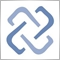0
6 135

MQL5 标准库已经补充了大量的来自 R 语言的数学函数。此外, 与 R 语言的初始版本相比, 处理速度提升了 3 到 7 倍。与此同时, 修正了 R 语言中已发现的某些函数的实现错误。

### 目录

1. 用于计算数组元素统计特性的函数

2. 使用统计分布的函数

2.1. 正态分布

2.2. 对数正态分布

2.3. β 分布

2.4. 非中心 β 分布

2.5. γ 分布

2.6. 卡方分布

2.7. 非中心卡方分布

2.8. 指数分布

2.9. F-分布

2.10. 非中心 F-分布

2.11. t-分布

2.12. 非中心 t-分布

2.13. 逻辑斯谛分布

2.14. 柯西分布

2.15. 均匀分布

2.16. 威布尔分布

2.17. 二项式分布

2.18. 负二项式分布

2.19. 几何分布

2.20. 超几何分布

2.21. 泊松分布

3. 对应于 R 语言的统计函数列表

4. 函数使用例程

5. 计算速度比较

6. 已检测到的 R 语言中的计算误差

7. 参考文献

### 概论

R 语言 是统计处理和数据分析的最佳工具之一。

### 1. 用于计算数组元素统计特性的函数

1.1. MathMean

```double MathMean(
const double   &array[]         // [输入] 数据数组
);
```

1.2. MathVariance

```double MathVariance(
const double   &array[]         // [输入] 数据数组
);

```

1.3. MathSkewness

```double MathSkewness(
const double   &array[]         // [输入] 数据数组
);
```

1.4. MathKurtosis

```double MathKurtosis(
const double   &array[]         // [输入] 数据数组
);
```

1.5. MathMoments

```bool MathMoments(
const double   &array[],         // [输入] 数据数组
double         &mean,            // [输出] 均值变量 (第一力矩)
double         &variance,        // [输出] 方差变量 (第二力矩)
double         &skewness,        // [输出] 偏度变量 (第三力矩)
double         &kurtosis,        // [输出] 峰度变量 (第四力矩)
const int      start=0,          // [输入] 初始计算索引
const int      count=WHOLE_ARRAY // [输入] 用于计算的元素数量

);
```

1.6. MathMedian

```double MathMedian(
double         &array[]         // [输入] 数据数组
);
```

1.7. MathStandardDeviation

```double MathStandardDeviation(
const double   &array[]         // [输入] 数据数组
);
```

1.8. MathAverageDeviation

```double MathAverageDeviation(
const double   &array[]         // [输入] 数据数组
);
```

### 2. 统计分布

MQL5 的统计库包含 5 个用于处理统计分布的函数:

1. 计算概率密度 (MathProbabilityDensityX() 函数);
2. 计算概率 (MathCumulativeDistributionX() 函数);
概率分布函数等于随机变量落在范围内 (-inf; x) 的概率。
3. 计算分布四分位数 (MathQuantileX() 函数);
对于给定的分布参数, 分布的四分位数 x 对应于一个落在具有指定概率的 (-int, x) 范围内的随机值。
4. 生成具有指定分布的随机数 (MathRandomX() 函数);
5. 计算分布的理论力矩 (MathMomentsX() 函数);

2.1. 正态分布

2.1.1. MathProbabilityDensityNormal

```double MathProbabilityDensityNormal(
const double   x,           // [输入] 随机变量值
const double   mu,          // [输入] 分布参数均值 (期望值)
const double   sigma,       // [输入] 分布参数的 sigma (均方根)
const bool     log_mode,    // [输入] 计算对数值的标志, 如果 log_mode = true, 则返回概率密度的自然对数
int            &error_code  // [输出] 错误代码变量
);
```

```double MathProbabilityDensityNormal(
const double   x,           // [输入] 随机变量值
const double   mu,          // [输入] 分布参数均值 (期望值)
const double   sigma,       // [输入] 分布参数的 sigma (均方根)
int            &error_code  // [输出] 错误代码变量
);
```

```bool MathProbabilityDensityNormal(
const double   &x[],        // [输入] 随机变量值的数组
const double   mu,          // [输入] 分布参数均值 (期望值)
const double   sigma,       // [输入] 分布参数的 sigma (均方根)
const bool     log_mode,    // [输入] 计算对数值的标志, 如果 log_mode = true, 则计算概率密度的自然对数
double         &result[]    // [输出] 概率密度值的数组
);
```

```bool MathProbabilityDensityNormal(
const double   &x[],        // [输入] 随机变量值的数组
const double   mu,          // [输入] 分布参数均值 (期望值)
const double   sigma,       // [输入] 分布参数的 sigma (均方根)
double         &result[]    // [输出] 概率密度值的数组
);
```

2.1.2. MathCumulativeDistributionNormal

```double MathCumulativeDistributionNormal(
const double   x,           // [输入] 随机变量值
const double   mu,          // [输入] 期望值
const double   sigma,       // [输入] 均方根偏离
const bool     tail,        // [输入] 计算标志, 如果 true, 则计算不超过随机变量 x 的概率
const bool     log_mode,    // [输入] 计算数值对数的标志, 如果 log_mode=true, 则计算概率的自然对数
int            &error_code  // [输出] 错误代码变量
);
```

```double MathCumulativeDistributionNormal(
const double   x,           // [输入] 随机变量值
const double   mu,          // [输入] 期望值
const double   sigma,       // [输入] 均方根偏离
int            &error_code  // [输出] 错误代码变量
);
```

```bool MathCumulativeDistributionNormal(
const double   &x[],        // [输入] 随机变量值的数组
const double   mu,          // [输入] 期望值
const double   sigma,       // [输入] 均方根偏离
const bool     tail,        // [输入] 计算标志, 如果 true, 则计算不超过随机变量 x 的概率
const bool     log_mode,    // [输入] 计算数值对数的标志, 如果 log_mode=true, 则计算概率的自然对数
double         &result[]    // [输出] 概率函数密度值的数组
);
```

```bool MathCumulativeDistributionNormal(
const double   &x[],        // [输入] 随机变量值的数组
const double   mu,          // [输入] 期望值
const double   sigma,       // [输入] 均方根偏离
double         &result[]    // [输出] 概率函数密度值的数组
);
```

2.1.3. MathQuantileNormal

```double MathQuantileNormal(
const double   probability, // [输入] 随机变量概率值
const double   mu,          // [输入] 期望值
const double   sigma,       // [输入] 均方根偏离
const bool     tail,        // [输入] 计算标志, 如果 false, 则执行 1.0-概率的计算
const bool     log_mode,    // [输入] 计算标志, 如果 log_mode=true, 执行 Exp(probability) 计算
int            &error_code  // [输出] 错误代码变量
);
```

```double MathQuantileNormal(
const double   probability, // [输入] 随机变量概率值
const double   mu,          // [输入] 期望值
const double   sigma,       // [输入] 均方根偏离
int            &error_code  // [输出] 错误代码变量
);
```

```bool MathQuantileNormal(
const double   &probability[],// [输入] 随机变量概率值数组
const double   mu,            // [输入] 期望值
const double   sigma,         // [输入] 均方根偏离
const bool     tail,          // [输入] 计算标志, 如果 false, 则执行 1.0-概率的计算
const bool     log_mode,      // [输入] 计算标志, 如果 log_mode=true, 执行 Exp(probability) 计算
double         &result[]      // [输出] 具有分位数值的数组
);
```

```bool MathQuantileNormal(
const double   &probability[],// [输入] 随机变量概率值数组
const double   mu,            // [输入] 期望值
const double   sigma,         // [输入] 均方根偏离
double         &result[]      // [输出] 具有分位数值的数组
);
```

2.1.4. MathRandomNormal

```double MathRandomNormal(
const double   mu,          // [输入] 期望值
const double   sigma,       // [输入] 均方根偏离
int            &error_code  // [输出] 错误代码变量
);

```

```bool MathRandomNormal(
const double   mu,          // [输入] 期望值
const double   sigma,       // [输入] 均方根偏离
const int      data_count,  // [输入] 所需数据数量
double         &result[]    // [输出] 伪随机变量值数组
);

```

2.1.5. MathMomentsNormal

```bool MathMomentsNormal(
const double   mu,          // [输入] 期望值
const double   sigma,       // [输入] 均方根偏离
double         &mean,       // [输出] 均值变量 (第一力矩)
double         &variance,   // [输出] 方差变量 (第二力矩)
double         &skewness,   // [输出] 偏度变量 (第三力矩)
double         &kurtosis,   // [输出] 峰度变量 (第四力矩)
int            &error_code  // [输出] 错误代码变量
);
```

2.2. 对数正态分布

2.2.1. MathProbabilityDensityLognormal

```double MathProbabilityDensityLognormal(
const double   x,           // [输入] 随机变量值
const double   mu,          // [输入] 期望值的对数 (对数均值)
const double   sigma,       // [输入] 均方根偏离的对数 (对数标准偏离)
const bool     log_mode,    // [输入] 计算对数值的标志, 如果 log_mode = true, 则返回概率密度的自然对数
int            &error_code  // [输出] 错误代码变量
);
```

```double MathProbabilityDensityLognormal(
const double   x,           // [输入] 随机变量值
const double   mu,          // [输入] 期望值的对数 (对数均值)
const double   sigma,       // [输入] 均方根偏离的对数 (对数标准偏离)
int            &error_code  // [输出] 错误代码变量
);
```

```bool MathProbabilityDensityLognormal(
const double   &x[],        // [输入] 随机变量值数组
const double   mu,          // [输入] 期望值的对数 (对数均值)
const double   sigma,       // [输入] 均方根偏离的对数 (对数标准偏离)
const bool     log_mode,    // [输入] 计算对数值的标志, 如果 log_mode = true, 则计算概率密度的自然对数
double         &result[]    // [输出] 概率密度值的数组
);
```

```bool MathProbabilityDensityLognormal(
const double   &x[],        // [输入] 随机变量值数组
const double   mu,          // [输入] 期望值的对数 (对数均值)
const double   sigma,       // [输入] 均方根偏离的对数 (对数标准偏离)
double         &result[]    // [输出] 概率密度值的数组
);
```

2.2.2. MathCumulativeDistributionLognormal

```double MathCumulativeDistributionLognormal(
const double   x,           // [输入] 随机变量值
const double   mu,          // [输入] 期望值的对数 (对数均值)
const double   sigma,       // [输入] 均方根偏离的对数 (对数标准偏离)
const bool     tail,        // [输入] 计算标志, 如果 true, 则计算不超过随机变量 x 的概率
const bool     log_mode,    // [输入] 计算数值对数的标志, 如果 log_mode=true, 则计算概率的自然对数
int            &error_code  // [输出] 错误代码变量
);
```

```double MathCumulativeDistributionLognormal(
const double   x,           // [输入] 随机变量值
const double   mu,          // [输入] 期望值的对数 (对数均值)
const double   sigma,       // [输入] 均方根偏离的对数 (对数标准偏离)
int            &error_code  // [输出] 错误代码变量
);
```

```bool MathCumulativeDistributionLognormal(
const double   &x[],        // [输入] 随机变量值的数组
const double   mu,          // [输入] 期望值的对数 (对数均值)
const double   sigma,       // [输入] 均方根偏离的对数 (对数标准偏离)
const bool     tail,        // [输入] 计算标志, 如果 true, 则计算不超过随机变量 x 的概率
const bool     log_mode,    // [输入] 计算数值对数的标志, 如果 log_mode=true, 则计算概率的自然对数
double         &result[]    // [输出] 概率函数密度值的数组
);
```

```bool MathCumulativeDistributionLognormal(
const double   &x[],        // [输入] 随机变量值的数组
const double   mu,          // [输入] 期望值的对数 (对数均值)
const double   sigma,       // [输入] 均方根偏离的对数 (对数标准偏离)
double         &result[]    // [输出] 概率函数密度值的数组
);
```

2.2.3. MathQuantileLognormal

```double MathQuantileLognormal(
const double   probability, // [输入] 随机变量发生概率值
const double   mu,          // [输入] 期望值的对数 (对数均值)
const double   sigma,       // [输入] 均方根偏离的对数 (对数标准偏离)
const bool     tail,        // [输入] 计算标志, 如果 false, 则执行 1.0-概率的计算
const bool     log_mode,    // [输入] 计算标志, 如果 log_mode=true, 执行 Exp(probability) 计算
int            &error_code  // [输出] 错误代码变量
);
```

```double MathQuantileLognormal(
const double   probability, // [输入] 随机变量发生概率值
const double   mu,          // [输入] 期望值的对数 (对数均值)
const double   sigma,       // [输入] 均方根偏离的对数 (对数标准偏离)
int            &error_code  // [输出] 错误代码变量
);
```

```bool MathQuantileLognormal(
const double   &probability[], // [输入] 随机变量概率值数组
const double   mu,             // [输入] 期望值的对数 (对数均值)
const double   sigma,          // [输入] 均方根偏离的对数 (对数标准偏离)
const bool     tail,           // [输入] 计算标志, 如果 false, 则执行 1.0-概率的计算
const bool     log_mode,       // [输入] 计算标志, 如果 log_mode=true, 执行 Exp(probability) 计算
double         &result[]       // [输出] 分位数值数组
);
```

```bool MathQuantileLognormal(
const double   &probability[], // [输入] 随机变量概率值数组
const double   mu,             // [输入] 期望值的对数 (对数均值)
const double   sigma,          // [输入] 均方根偏离的对数 (对数标准偏离)
double         &result[]       // [输出] 分位数值数组
);
```

2.2.4. MathRandomLognormal

```double MathRandomLognormal(
const double   mu,          // [输入] 期望值的对数 (对数均值)
const double   sigma,       // [输入] 均方根偏离的对数 (对数标准偏离)
int            &error_code  // [输出] 错误代码变量
);
```

```bool MathRandomLognormal(
const double   mu,          // [输入] 期望值的对数 (对数均值)
const double   sigma,       // [输入] 均方根偏离的对数 (对数标准偏离)
const int      data_count,  // [输入] 所需数据数量
double         &result[]    // [输出] 伪随机变量值数组
);
```

2.2.5. MathMomentsLognormal

```bool MathMomentsLognormal(
const double   mu,          // [输入] 期望值的对数 (对数均值)
const double   sigma,       // [输入] 均方根偏离的对数 (对数标准偏离)
double         &mean,       // [输出] 均值变量 (第一力矩)
double         &variance,   // [输出] 方差变量 (第二力矩)
double         &skewness,   // [输出] 偏度变量 (第三力矩)
double         &kurtosis,   // [输出] 峰度变量 (第四力矩)
int            &error_code  // [输出] 错误代码变量
);
```

2.3. β 分布

2.3.1. MathProbabilityDensityBeta

```double MathProbabilityDensityBeta(
const double   x,           // [输入] 随机变量值
const double   a,           // [输入] β 分布的第一个参数 (shape1)
const double   b,           // [输入] β 分布的第二个参数 (shape2)
const bool     log_mode,    // [输入] 计算对数值的标志, 如果 log_mode = true, 则返回概率密度的自然对数
int            &error_code  // [输出] 错误代码变量
);
```

```double MathProbabilityDensityBeta(
const double   x,           // [输入] 随机变量值
const double   a,           // [输入] β 分布的第一个参数 (shape1)
const double   b,           // [输入] β 分布的第二个参数 (shape2)
int            &error_code  // [输出] 错误代码变量
);
```

```bool MathProbabilityDensityBeta(
const double   &x[],        // [输入] 随机变量值数组
const double   a,           // [输入] β 分布的第一个参数 (shape1)
const double   b,           // [输入] β 分布的第二个参数 (shape2)
const bool     log_mode,    // [输入] 计算对数值的标志, 如果 log_mode = true, 则计算概率密度的自然对数
double         &result[]    // [输出] 概率密度值的数组
);
```

```bool MathProbabilityDensityBeta(
const double   &x[],        // [输入] 随机变量值数组
const double   a,           // [输入] β 分布的第一个参数 (shape1)
const double   b,           // [输入] β 分布的第二个参数 (shape2)
double         &result[]    // [输出] 概率密度值的数组
);
```

2.3.2. MathCumulativeDistributionlBeta

```double MathCumulativeDistributionBeta(
const double   x,           // [输入] 随机变量值
const double   a,           // [输入] β 分布的第一个参数 (shape1)
const double   b,           // [输入] β 分布的第二个参数 (shape2)
const bool     tail,        // [输入] 计算标志, 如果 true, 则计算不超过随机变量 x 的概率
const bool     log_mode,    // [输入] 计算数值对数的标志, 如果 log_mode=true, 则计算概率的自然对数
int            &error_code  // [输出] 错误代码变量
);
```

```double MathCumulativeDistributionBeta(
const double   x,           // [输入] 随机变量值
const double   a,           // [输入] β 分布的第一个参数 (shape1)
const double   b,           // [输入] β 分布的第二个参数 (shape2)
int            &error_code  // [输出] 错误代码变量
);
```

```bool MathCumulativeDistributionBeta(
const double   &x[],        // [输入] 随机变量值的数组
const double   a,           // [输入] β 分布的第一个参数 (shape1)
const double   b,           // [输入] β 分布的第二个参数 (shape2)
const bool     tail,        // [输入] 计算标志, 如果 true, 则计算不超过随机变量 x 的概率
const bool     log_mode,    // [输入] 计算数值对数的标志, 如果 log_mode=true, 则计算概率的自然对数
double         &result[]    // [输出] 概率函数密度值的数组
);
```

```bool MathCumulativeDistributionBeta(
const double   &x[],        // [输入] 随机变量值的数组
const double   a,           // [输入] β 分布的第一个参数 (shape1)
const double   b,           // [输入] β 分布的第二个参数 (shape2)
double         &result[]    // [输出] 概率函数密度值的数组
);
```

2.3.3. MathQuantileBeta

```double MathQuantileBeta(
const double   probability,   // [输入] 随机变量发生概率值
const double   a,             // [输入] β 分布的第一个参数 (shape1)
const double   b,             // [输入] β 分布的第二个参数 (shape2)
const bool     tail,          // [输入] 计算标志, 如果 false, 则执行 1.0-概率的计算
const bool     log_mode,      // [输入] 计算标志, 如果 log_mode=true, 执行 Exp(probability) 计算
int            &error_code    // [输出] 错误代码变量
);
```

```double MathQuantileBeta(
const double   probability,   // [输入] 随机变量发生概率值
const double   a,             // [输入] β 分布的第一个参数 (shape1)
const double   b,             // [输入] β 分布的第二个参数 (shape2)
int            &error_code    // [输出] 错误代码变量
);
```

```bool MathQuantileBeta(
const double   &probability[],// [输入] 随机变量概率值数组
const double   a,             // [输入] β 分布的第一个参数 (shape1)
const double   b,             // [输入] β 分布的第二个参数 (shape2)
const bool     tail,          // [输入] 计算标志, 如果 false, 则执行 1.0-概率的计算
const bool     log_mode,      // [输入] 计算标志, 如果 log_mode=true, 执行 Exp(probability) 计算
double         &result[]      // [输出] 具有分位数值的数组
);
```

```bool MathQuantileBeta(
const double   &probability[],// [输入] 随机变量概率值数组
const double   a,             // [输入] β 分布的第一个参数 (shape1)
const double   b,             // [输入] β 分布的第二个参数 (shape2)
double         &result[]      // [输出] 具有分位数值的数组
);
```

2.3.4. MathRandomBeta

```double MathRandomBeta(
const double   a,           // [输入] β 分布的第一个参数 (shape1)
const double   b,           // [输入] β 分布的第二个参数 (shape2)
int            &error_code  // [输出] 错误代码变量
);
```

```bool MathRandomBeta(
const double   a,           // [输入] β 分布的第一个参数 (shape1)
const double   b,           // [输入] β 分布的第二个参数 (shape2)
const int      data_count,  // [输入] 所需数据数量
double         &result[]    // [输出] 伪随机变量值数组
);
```

2.3.5. MathMomentsBeta

```bool MathMomentsBeta(
const double   a,           // [输入] β 分布的第一个参数 (shape1)
const double   b,           // [输入] β 分布的第二个参数 (shape2)
double         &mean,       // [输出] 均值变量 (第一力矩)
double         &variance,   // [输出] 方差变量 (第二力矩)
double         &skewness,   // [输出] 偏度变量 (第三力矩)
double         &kurtosis,   // [输出] 峰度变量 (第四力矩)
int            &error_code  // [输出] 错误代码变量
);
```

2.4. 非中心 β 分布

2.4.1. MathProbabilityDensityNoncentralBeta

```double MathProbabilityDensityNoncentralBeta(
const double   x,           // [输入] 随机变量值
const double   a,           // [输入] β 分布的第一个参数 (shape1)
const double   b,           // [输入] β 分布的第二个参数 (shape2)
const double   lambda,      // [输入] 非中心参数
const bool     log_mode,    // [输入] 计算对数值的标志, 如果 log_mode = true, 则返回概率密度的自然对数
int            &error_code  // [输出] 错误代码变量
);
```

```double MathProbabilityDensityNoncentralBeta(
const double   x,           // [输入] 随机变量值
const double   a,           // [输入] β 分布的第一个参数 (shape1)
const double   b,           // [输入] β 分布的第二个参数 (shape2)
const double   lambda,      // [输入] 非中心参数
int            &error_code  // [输出] 错误代码变量
);
```

```bool MathProbabilityDensityNoncentralBeta(
const double   &x[],        // [输入] 随机变量值的数组
const double   a,           // [输入] β 分布的第一个参数 (shape1)
const double   b,           // [输入] β 分布的第二个参数 (shape2)
const double   lambda,      // [输入] 非中心参数
const bool     log_mode,    // [输入] 计算对数值的标志, 如果 log_mode = true, 则返回概率密度的自然对数
double         &result[]    // [输出] 概率密度值的数组
);
```

```bool MathProbabilityDensityNoncentralBeta(
const double   &x[],        // [输入] 随机变量值的数组
const double   a,           // [输入] β 分布的第一个参数 (shape1)
const double   b,           // [输入] β 分布的第二个参数 (shape2)
const double   lambda,      // [输入] 非中心参数
double         &result[]    // [输出] 概率密度值的数组
);
```

2.4.2. MathCumulativeDistributionNoncentralBeta

```double MathCumulativeDistributionNoncentralBeta(
const double   x,           // [输入] 随机变量值
const double   a,           // [输入] β 分布的第一个参数 (shape1)
const double   b,           // [输入] β 分布的第二个参数 (shape2)
const double   lambda,      // [输入] 非中心参数
const bool     tail,        // [输入] 计算标志, 如果 true, 则计算不超过随机变量 x 的概率
const bool     log_mode,    // [输入] 计算数值对数的标志, 如果 log_mode=true, 则计算概率的自然对数
int            &error_code  // [输出] 错误代码变量
);
```

```double MathCumulativeDistributionNoncentralBeta(
const double   x,           // [输入] 随机变量值
const double   a,           // [输入] β 分布的第一个参数 (shape1)
const double   b,           // [输入] β 分布的第二个参数 (shape2)
const double   lambda,      // [输入] 非中心参数
int            &error_code  // [输出] 错误代码变量
);
```

```bool MathCumulativeDistributionNoncentralBeta(
const double   &x[],        // [输入] 随机变量值的数组
const double   a,           // [输入] β 分布的第一个参数 (shape1)
const double   b,           // [输入] β 分布的第二个参数 (shape2)
const double   lambda,      // [输入] 非中心参数
const bool     tail,        // [输入] 计算标志, 如果 true, 则计算不超过随机变量 x 的概率
const bool     log_mode,    // [输入] 计算数值对数的标志, 如果 log_mode=true, 则计算概率的自然对数
double         &result[]    // [输出] 概率函数密度值的数组
);
```

```bool MathCumulativeDistributionNoncentralBeta(
const double   &x[],        // [输入] 随机变量值的数组
const double   a,           // [输入] β 分布的第一个参数 (shape1)
const double   b,           // [输入] β 分布的第二个参数 (shape2)
const double   lambda,      // [输入] 非中心参数
double         &result[]    // [输出] 概率函数密度值的数组
);
```

2.4.3. MathQuantileNoncentralBeta

```double MathQuantileNoncentralBeta(
const double   probability,   // [输入] 随机变量发生概率
const double   a,             // [输入] β 分布的第一个参数 (shape1)
const double   b,             // [输入] β 分布的第二个参数 (shape2)
const double   lambda,        // [输入] 非中心参数
const bool     tail,          // [输入] 计算标志, 如果 false, 则执行 1.0-概率的计算
const bool     log_mode,      // [输入] 计算标志, 如果 log_mode=true, 执行 Exp(probability) 计算
int            &error_code    // [输出] 错误代码变量
);
```

```double MathQuantileNoncentralBeta(
const double   probability,   // [输入] 随机变量发生概率
const double   a,             // [输入] β 分布的第一个参数 (shape1)
const double   b,             // [输入] β 分布的第二个参数 (shape2)
const double   lambda,        // [输入] 非中心参数
int            &error_code    // [输出] 错误代码变量
);
```

```bool MathQuantileNoncentralBeta(
const double   &probability[],// [输入] 随机变量概率值数组
const double   a,             // [输入] β 分布的第一个参数 (shape1)
const double   b,             // [输入] β 分布的第二个参数 (shape2)
const double   lambda,        // [输入] 非中心参数
const bool     tail,          // [输入] 计算标志, 如果 false, 则执行 1.0-概率的计算
const bool     log_mode,      // [输入] 计算标志, 如果 log_mode=true, 执行 Exp(probability) 计算
double         &result[]      // [输出] 具有分位数值的数组
);
```

```bool MathQuantileNoncentralBeta(
const double   &probability[],// [输入] 随机变量概率值数组
const double   a,             // [输入] β 分布的第一个参数 (shape1)
const double   b,             // [输入] β 分布的第二个参数 (shape2)
const double   lambda,        // [输入] 非中心参数
double         &result[]      // [输出] 具有分位数值的数组
);
```

2.4.4. MathRandomNoncentralBeta

```double MathRandomNoncentralBeta(
const double   a,           // [输入] β 分布的第一个参数 (shape1)
const double   b,           // [输入] β 分布的第二个参数 (shape2)
const double   lambda,      // [输入] 非中心参数
int            &error_code  // [输出] 错误代码变量
);
```

```bool MathRandomNoncentralBeta(
const double   a,           // [输入] β 分布的第一个参数 (shape1)
const double   b,           // [输入] β 分布的第二个参数 (shape2)
const double   lambda,      // [输入] 非中心参数
const int      data_count,  // [输入] 所需数据数量
double         &result[]    // [输出] 伪随机变量值数组
);
```

2.4.5. MathMomentsNoncentralBeta

```double MathMomentsNoncentralBeta(
const double   a,           // [输入] β 分布的第一个参数 (shape1)
const double   b,           // [输入] β 分布的第二个参数 (shape2)
const double   lambda,      // [输入] 非中心参数
double         &mean,       // [输出] 均值变量 (第一力矩)
double         &variance,   // [输出] 方差变量 (第二力矩)
double         &skewness,   // [输出] 偏度变量 (第三力矩)
double         &kurtosis,   // [输出] 峰度变量 (第四力矩)
int            &error_code  // [输出] 错误代码变量
);
```

2.5. γ 分布

2.5.1. MathProbabilityDensityGamma

```double MathProbabilityDensityGamma(
const double   x,           // [输入] 随机变量值
const double   a,           // [输入] 第一个 分布参数 (shape)
const double   b,           // [输入] 第二个分布参数 (scale)
const bool     log_mode,    // [输入] 计算对数值的标志, 如果 log_mode = true, 则计算概率密度的自然对数
int            &error_code  // [输出] 错误代码变量
);
```

```double MathProbabilityDensityGamma(
const double   x,           // [输入] 随机变量值
const double   a,           // [输入] 第一个 分布参数 (shape)
const double   b,           // [输入] 第二个分布参数 (scale)
int            &error_code  // [输出] 错误代码变量
);
```

```bool MathProbabilityDensityGamma(
const double   &x[],        // [输入] 随机变量值的数组
const double   a,           // [输入] 第一个 分布参数 (shape)
const double   b,           // [输入] 第二个分布参数 (scale)
const bool     log_mode,    // [输入] 计算对数值的标志, 如果 log_mode = true, 则计算概率密度的自然对数
double         &result[]    // [输出] 概率密度值的数组
);
```

```bool MathProbabilityDensityGamma(
const double   &x[],        // [输入] 随机变量值的数组
const double   a,           // [输入] 第一个 分布参数 (shape)
const double   b,           // [输入] 第二个分布参数 (scale)
double         &result[]    // [输出] 概率密度值的数组
);
```

2.5.2. MathCumulativeDistributionGamma

```double MathCumulativeDistributionGamma(
const double   x,           // [输入] 随机变量值
const double   a,           // [输入] 第一个 分布参数 (shape)
const double   b,           // [输入] 第二个分布参数 (scale)
const bool     tail,        // [输入] 计算标志, 如果 true, 则计算不超过随机变量 x 的概率
const bool     log_mode,    // [输入] 计算数值对数的标志, 如果 log_mode=true, 则计算概率的自然对数
int            &error_code  // [输出] 错误代码变量
);
```

```double MathCumulativeDistributionGamma(
const double   x,           // [输入] 随机变量值
const double   a,           // [输入] 第一个 分布参数 (shape)
const double   b,           // [输入] 第二个分布参数 (scale)
int            &error_code  // [输出] 错误代码变量
);
```

```bool MathCumulativeDistributionGamma(
const double   &x[],        // [输入] 随机变量值的数组
const double   a,           // [输入] 第一个 分布参数 (shape)
const double   b,           // [输入] 第二个分布参数 (scale)
const bool     tail,        // [输入] 计算标志, 如果 true, 则计算不超过随机变量 x 的概率
const bool     log_mode,    // [输入] 计算数值对数的标志, 如果 log_mode=true, 则计算概率的自然对数
double         &result[]    // [输出] 概率函数密度值的数组
);
```

```bool MathCumulativeDistributionGamma(
const double   &x[],        // [输入] 随机变量值的数组
const double   a,           // [输入] 第一个 分布参数 (shape)
const double   b,           // [输入] 第二个分布参数 (scale)
double         &result[]    // [输出] 概率函数密度值的数组
);
```

2.5.3. MathQuantileGamma

```double MathQuantileGamma(
const double   probability,   // [输入] 随机变量发生概率
const double   a,             // [输入] 第一个 分布参数 (shape)
const double   b,             // [输入] 第二个分布参数 (scale)
const bool     tail,          // [输入] 计算标志, 如果 false, 则执行 1.0-概率的计算
const bool     log_mode,      // [输入] 计算标志, 如果 log_mode=true, 执行 Exp(probability) 计算
int            &error_code    // [输出] 错误代码变量
);
```

```double MathQuantileGamma(
const double   probability,   // [输入] 随机变量发生概率
const double   a,             // [输入] 第一个 分布参数 (shape)
const double   b,             // [输入] 第二个分布参数 (scale)
int            &error_code    // [输出] 错误代码变量
);
```

```bool MathQuantileGamma(
const double   &probability[],// [输入] 随机变量概率值数组
const double   a,             // [输入] 第一个 分布参数 (shape)
const double   b,             // [输入] 第二个分布参数 (scale)
const bool     tail,          // [输入] 计算标志, 如果 false, 则执行 1.0-概率的计算
const bool     log_mode,      // [输入] 计算标志, 如果 log_mode=true, 执行 Exp(probability) 计算
double         &result[]      // [输出] 具有分位数值的数组
);
```

```bool MathQuantileGamma(
const double   &probability[],// [输入] 随机变量概率值数组
const double   a,             // [输入] 第一个 分布参数 (shape)
const double   b,             // [输入] 第二个分布参数 (scale)
double         &result[]      // [输出] 具有分位数值的数组
);
```

2.5.4. MathRandomGamma

```double MathRandomGamma(
const double   a,           // [输入] 第一个 分布参数 (shape)
const double   b,           // [输入] 第二个分布参数 (scale)
int            &error_code  // [输出] 错误代码变量
);
```

```bool MathRandomGamma(
const double   a,           // [输入] 第一个 分布参数 (shape)
const double   b,           // [输入] 第二个分布参数 (scale)
const int      data_count,  // [输入] 所需数据数量
double         &result[]    // [输出] 伪随机变量值数组
);
```

2.5.5. MathMomentsGamma

```bool MathMomentsGamma(
const double   a,           // [输入] 第一个 分布参数 (shape)
const double   b,           // [输入] 第二个分布参数 (scale)
double         &mean,       // [输出] 均值变量 (第一力矩)
double         &variance,   // [输出] 方差变量 (第二力矩)
double         &skewness,   // [输出] 偏度变量 (第三力矩)
double         &kurtosis,   // [输出] 峰度变量 (第四力矩)
int            &error_code  // [输出] 错误代码变量
);
```

2.6. 卡方分布

2.6.1. MathProbabilityDensityChiSquare

```double MathProbabilityDensityChiSquare(
const double   x,           // [输入] 随机变量值
const double   nu,          // [输入] 分布参数 (自由度数值)
const bool     log_mode,    // [输入] 计算对数值的标志, 如果 log_mode = true, 则返回概率密度的自然对数
int            &error_code  // [输出] 错误代码变量
);
```

```double MathProbabilityDensityChiSquare(
const double   x,           // [输入] 随机变量值
const double   nu,          // [输入] 分布参数 (自由度数值)
int            &error_code  // [输出] 错误代码变量
);
```

```bool MathProbabilityDensityChiSquare(
const double   &x[],        // [输入] 随机变量值的数组
const double   nu,          // [输入] 分布参数 (自由度数值)
const bool     log_mode,    // [输入] 计算对数值的标志, 如果 log_mode = true, 则返回概率密度的自然对数
double         &result[]    // [输出] 概率密度值的数组
);
```

```bool MathProbabilityDensityChiSquare(
const double   &x[],        // [输入] 随机变量值的数组
const double   nu,          // [输入] 分布参数 (自由度数值)
double         &result[]    // [输出] 概率密度值的数组
);
```

2.6.2. MathCumulativeDistributionChiSquare

```double MathCumulativeDistributionChiSquare(
const double   x,           // [输入] 随机变量值
const double   nu,          // [输入] 分布参数 (自由度数值)
const bool     tail,        // [输入] 计算标志, 如果 true, 则计算不超过随机变量 x 的概率
const bool     log_mode,    // [输入] 计算数值对数的标志, 如果 log_mode=true, 则计算概率的自然对数
int            &error_code  // [输出] 错误代码变量
);
```

```double MathCumulativeDistributionChiSquare(
const double   x,           // [输入] 随机变量值
const double   nu,          // [输入] 分布参数 (自由度数值)
int            &error_code  // [输出] 错误代码变量
);
```

```bool MathCumulativeDistributionChiSquare(
const double   &x[],        // [输入] 随机变量值的数组
const double   nu,          // [输入] 分布参数 (自由度数值)
const bool     tail,        // [输入] 计算标志, 如果 true, 则计算不超过随机变量 x 的概率
const bool     log_mode,    // [输入] 计算数值对数的标志, 如果 log_mode=true, 则计算概率的自然对数
double         &result[]    // [输出] 概率函数密度值的数组
);
```

```bool MathCumulativeDistributionChiSquare(
const double   &x[],        // [输入] 随机变量值的数组
const double   nu,          // [输入] 分布参数 (自由度数值)
double         &result[]    // [输出] 概率函数密度值的数组
);
```

2.6.3. MathQuantileChiSquare

```double MathQuantileChiSquare(
const double   probability,   // [输入] 随机变量发生概率
const double   nu,            // [输入] 分布参数 (自由度数值)
const bool     tail,          // [输入] 计算标志, 如果 false, 则执行 1.0-概率的计算
const bool     log_mode,      // [输入] 计算标志, 如果 log_mode=true, 执行 Exp(probability) 计算
int            &error_code    // [输出] 错误代码变量
);
```

```double MathQuantileChiSquare(
const double   probability,   // [输入] 随机变量发生概率
const double   nu,            // [输入] 分布参数 (自由度数值)
int            &error_code    // [输出] 错误代码变量
);
```

```bool MathQuantileChiSquare(
const double   &probability[],// [输入] 随机变量概率值数组
const double   nu,            // [输入] 分布参数 (自由度数值)
const bool     tail,          // [输入] 计算标志, 如果 false, 则执行 1.0-概率的计算
const bool     log_mode,      // [输入] 计算标志, 如果 log_mode=true, 执行 Exp(probability) 计算
double         &result[]      // [输出] 具有分位数值的数组
);
```

```bool MathQuantileChiSquare(
const double   &probability[],// [输入] 随机变量概率值数组
const double   nu,            // [输入] 分布参数 (自由度数值)
double         &result[]      // [输出] 具有分位数值的数组
);
```

2.6.4. MathRandomChiSquare

```double MathRandomChiSquare(
const double   nu,          // [输入] 分布参数 (自由度数值)
int            &error_code  // [输出] 错误代码变量
);
```

```bool MathRandomChiSquare(
const double   nu,          // [输入] 分布参数 (自由度数值)
const int      data_count,  // [输入] 所需数据数量
double         &result[]    // [输出] 伪随机变量值数组
);
```

2.6.5. MathMomentsChiSquare

```bool MathMomentsChiSquare(
const double   nu,          // [输入] 分布参数 (自由度数值)
double         &mean,       // [输出] 均值变量 (第一力矩)
double         &variance,   // [输出] 方差变量 (第二力矩)
double         &skewness,   // [输出] 偏度变量 (第三力矩)
double         &kurtosis,   // [输出] 峰度变量 (第四力矩)
int            &error_code  // [输出] 错误代码变量
);
```

2.7. 非中心卡方分布

2.7.1. MathProbabilityDensityNoncentralChiSquare

```double MathProbabilityDensityNoncentralChiSquare(
const double   x,           // [输入] 随机变量值
const double   nu,          // [输入] 分布参数 (自由度数值)
const double   sigma,       // [输入] 非中心参数
const bool     log_mode,    // [输入] 计算对数值的标志, 如果 log_mode = true, 则返回概率密度的自然对数
int            &error_code  // [输出] 错误代码变量
);
```

```double MathProbabilityDensityNoncentralChiSquare(
const double   x,           // [输入] 随机变量值
const double   nu,          // [输入] 分布参数 (自由度数值)
const double   sigma,       // [输入] 非中心参数
int            &error_code  // [输出] 错误代码变量
);
```

```bool MathProbabilityDensityNoncentralChiSquare(
const double   &x[],        // [输入] 随机变量值的数组
const double   nu,          // [输入] 分布参数 (自由度数值)
const double   sigma,       // [输入] 非中心参数
const bool     log_mode,    // [输入] 计算对数值的标志, 如果 log_mode = true, 则返回概率密度的自然对数
double         &result[]    // [输出] 概率密度值的数组
);
```

```bool MathProbabilityDensityNoncentralChiSquare(
const double   &x[],        // [输入] 随机变量值的数组
const double   nu,          // [输入] 分布参数 (自由度数值)
const double   sigma,       // [输入] 非中心参数
double         &result[]    // [输出] 概率密度值的数组
);
```

2.7.2. MathCumulativeDistributionNoncentralChiSquare

```double MathCumulativeDistributionNoncentralChiSquare(
const double   x,           // [输入] 随机变量值
const double   nu,          // [输入] 分布参数 (自由度数值)
const double   sigma,       // [输入] 非中心参数
const bool     tail,        // [输入] 计算标志, 如果 true, 则计算不超过随机变量 x 的概率
const bool     log_mode,    // [输入] 计算数值对数的标志, 如果 log_mode=true, 则计算概率的自然对数
int            &error_code  // [输出] 错误代码变量
);
```

```double MathCumulativeDistributionNoncentralChiSquare(
const double   x,           // [输入] 随机变量值
const double   nu,          // [输入] 分布参数 (自由度数值)
const double   sigma,       // [输入] 非中心参数
int            &error_code  // [输出] 错误代码变量
);
```

```bool MathCumulativeDistributionNoncentralChiSquare(
const double   &x[],        // [输入] 随机变量值的数组
const double   nu,          // [输入] 分布参数 (自由度数值)
const double   sigma,       // [输入] 非中心参数
const bool     tail,        // [输入] 计算标志, 如果 true, 则计算不超过随机变量 x 的概率
const bool     log_mode,    // [输入] 计算数值对数的标志, 如果 log_mode=true, 则计算概率的自然对数
double         &result[]    // [输出] 概率函数密度值的数组
);
```

```bool MathCumulativeDistributionNoncentralChiSquare(
const double   &x[],        // [输入] 随机变量值的数组
const double   nu,          // [输入] 分布参数 (自由度数值)
const double   sigma,       // [输入] 非中心参数
double         &result[]    // [输出] 概率函数密度值的数组
);
```

2.7.3. MathQuantileNoncentralChiSquare

```double MathQuantileNoncentralChiSquare(
const double   probability,   // [输入] 随机变量发生概率值
const double   nu,            // [输入] 分布参数 (自由度数值)
const double   sigma,         // [输入] 非中心参数
const bool     tail,          // [输入] 计算标志, 如果 false, 则执行 1.0-概率的计算
const bool     log_mode,      // [输入] 计算标志, 如果 log_mode=true, 执行 Exp(probability) 计算
int            &error_code    // [输出] 错误代码变量
);
```

```double MathQuantileNoncentralChiSquare(
const double   probability,   // [输入] 随机变量发生概率值
const double   nu,            // [输入] 分布参数 (自由度数值)
const double   sigma,         // [输入] 非中心参数
int            &error_code    // [输出] 错误代码变量
);
```

```bool MathQuantileNoncentralChiSquare(
const double   &probability[],// [输入] 随机变量概率值数组
const double   nu,            // [输入] 分布参数 (自由度数值)
const double   sigma,         // [输入] 非中心参数
const bool     tail,          // [输入] 计算标志, 如果 false, 则执行 1.0-概率的计算
const bool     log_mode,      // [输入] 计算标志, 如果 log_mode=true, 执行 Exp(probability) 计算
double         &result[]      // [输出] 具有分位数值的数组
);
```

```bool MathQuantileNoncentralChiSquare(
const double   &probability[],// [输入] 随机变量概率值数组
const double   nu,            // [输入] 分布参数 (自由度数值)
const double   sigma,         // [输入] 非中心参数
double         &result[]      // [输出] 具有分位数值的数组
);
```

2.7.4. MathRandomNoncentralChiSquare

```double MathRandomNoncentralChiSquare(
const double   nu,          // [输入] 分布参数 (自由度数值)
const double   sigma,       // [输入] 非中心参数
int            &error_code  // [输出] 错误代码变量
);
```

```bool MathRandomNoncentralChiSquare(
const double   nu,          // [输入] 分布参数 (自由度数值)
const double   sigma,       // [输入] 非中心参数
const int      data_count,  // [输入] 所需数据数量
double         &result[]    // [输出] 伪随机变量值数组
);
```

2.7.5. MathMomentsNoncentralChiSquare

```bool MathMomentsNoncentralChiSquare(
const double   nu,          // [输入] 分布参数 (自由度数值)
const double   sigma,       // [输入] 非中心参数
double         &mean,       // [输出] 均值变量 (第一力矩)
double         &variance,   // [输出] 方差变量 (第二力矩)
double         &skewness,   // [输出] 偏度变量 (第三力矩)
double         &kurtosis,   // [输出] 峰度变量 (第四力矩)
int            &error_code  // [输出] 错误代码变量
);
```

2.8. 指数分布

2.8.1. MathProbabilityDensityExponential

```double MathProbabilityDensityExponential(
const double   x,           // [输入] 随机变量值
const double   mu,          // [输入] 分布参数 (期望值)
const bool     log_mode,    // [输入] 计算对数值的标志, 如果 log_mode = true, 则返回概率密度的自然对数
int            &error_code  // [输出] 错误代码变量
);
```

```double MathProbabilityDensityExponential(
const double   x,           // [输入] 随机变量值
const double   mu,          // [输入] 分布参数 (期望值)
int            &error_code  // [输出] 错误代码变量
);
```

```bool MathProbabilityDensityExponential(
const double   &x[],        // [输入] 随机变量值的数组
const double   mu,          // [输入] 分布参数 (期望值)
const bool     log_mode,    // [输入] 计算对数值的标志, 如果 log_mode = true, 则计算概率密度的自然对数
double         &result[]    // [输出] 概率密度值的数组
);
```

```bool MathProbabilityDensityExponential(
const double   &x[],        // [输入] 随机变量值的数组
const double   mu,          // [输入] 分布参数 (期望值)
double         &result[]    // [输出] 概率密度值的数组
);
```

2.8.2. MathCumulativeDistributionExponential

```double MathCumulativeDistributionExponential(
const double   x,           // [输入] 随机变量值
const double   mu,          // [输入] 分布参数 (期望值)
const bool     tail,        // [输入] 计算标志, 如果 true, 则计算不超过随机变量 x 的概率
const bool     log_mode,    // [输入] 计算数值对数的标志, 如果 log_mode=true, 则计算概率的自然对数
int            &error_code  // [输出] 错误代码变量
);

```

```double MathCumulativeDistributionExponential(
const double   x,           // [输入] 随机变量值
const double   mu,          // [输入] 分布参数 (期望值)
int            &error_code  // [输出] 错误代码变量
);

```

```bool MathCumulativeDistributionExponential(
const double   &x[],        // [输入] 随机变量值的数组
const double   mu,          // [输入] 分布参数 (期望值)
const bool     tail,        // [输入] 计算标志, 如果 true, 则计算不超过随机变量 x 的概率
const bool     log_mode,    // [输入] 计算数值对数的标志, 如果 log_mode=true, 则计算概率的自然对数
double         &result[]    // [输出] 概率函数密度值的数组
);

```

```bool MathCumulativeDistributionExponential(
const double   &x[],        // [输入] 随机变量值的数组
const double   mu,          // [输入] 分布参数 (期望值)
double         &result[]    // [输出] 概率函数密度值的数组
);

```

2.8.3. MathQuantileExponential

```double MathQuantileExponential(
const double   probability,   // [输入] 随机变量发生概率
const double   mu,            // [输入] 分布参数 (期望值)
const bool     tail,          // [输入] 计算标志, 如果 false, 则执行 1.0-概率的计算
const bool     log_mode,      // [输入] 计算标志, 如果 log_mode=true, 执行 Exp(probability) 计算
int            &error_code    // [输出] 错误代码变量
);
```

```double MathQuantileExponential(
const double   probability,   // [输入] 随机变量发生概率
const double   mu,            // [输入] 分布参数 (期望值)
int            &error_code    // [输出] 错误代码变量
);
```

```bool MathQuantileExponential(
const double   &probability[],// [输入] 随机变量概率值数组
const double   mu,            // [输入] 分布参数 (期望值)
const bool     tail,          // [输入] 计算标志, 如果 false, 则执行 1.0-概率的计算
const bool     log_mode,      // [输入] 计算标志, 如果 log_mode=true, 执行 Exp(probability) 计算
double         &result[]      // [输出] 具有分位数值的数组
);
```

```bool MathQuantileExponential(
const double   &probability[],// [输入] 随机变量概率值数组
const double   mu,            // [输入] 分布参数 (期望值)
double         &result[]      // [输出] 具有分位数值的数组
);
```

2.8.4. MathRandomExponential

```double MathRandomExponential(
const double   mu,          // [输入] 分布参数 (期望值)
int            &error_code  // [输出] 错误代码变量
);
```

```bool MathRandomExponential(
const double   mu,          // [输入] 分布参数 (期望值)
const int      data_count,  // [输入] 所需数据数量
double         &result[]    // [输出] 伪随机变量值数组
);
```

2.8.5. MathMomentsExponential

```bool MathMomentsExponential(
const double   mu,          // [输入] 分布参数 (期望值)
double         &mean,       // [输出] 均值变量 (第一力矩)
double         &variance,   // [输出] 方差变量 (第二力矩)
double         &skewness,   // [输出] 偏度变量 (第三力矩)
double         &kurtosis,   // [输出] 峰度变量 (第四力矩)
int            &error_code  // [输出] 错误代码变量
);
```

2.9. F-分布

2.9.1. MathProbabilityDensityF

```double MathProbabilityDensityF(
const double   x,           // [输入] 随机变量值
const double   nu1,         // [输入] 第一个分布参数 (自由度数值)
const double   nu2,         // [输入] 第二个分布参数 (自由度数值)
const bool     log_mode,    // [输入] 计算对数值的标志, 如果 log_mode = true, 则返回概率密度的自然对数
int            &error_code  // [输出] 错误代码变量
);
```

```double MathProbabilityDensityF(
const double   x,           // [输入] 随机变量值
const double   nu1,         // [输入] 第一个分布参数 (自由度数值)
const double   nu2,         // [输入] 第二个分布参数 (自由度数值)
int            &error_code  // [输出] 错误代码变量
);
```

```bool MathProbabilityDensityF(
const double   &x[],        // [输入] 随机变量值的数组
const double   nu1,         // [输入] 第一个分布参数 (自由度数值)
const double   nu2,         // [输入] 第二个分布参数 (自由度数值)
const bool     log_mode,    // [输入] 计算对数值的标志, 如果 log_mode = true, 则返回概率密度的自然对数
double         &result[]    // [输出] 概率密度值的数组
);
```

```bool MathProbabilityDensityF(
const double   &x[],        // [输入] 随机变量值的数组
const double   nu1,         // [输入] 第一个分布参数 (自由度数值)
const double   nu2,         // [输入] 第二个分布参数 (自由度数值)
double         &result[]    // [输出] 概率密度值的数组
);
```

2.9.2. MathCumulativeDistributionF

```double MathCumulativeDistributionF(
const double   x,           // [输入] 随机变量值
const double   nu1,         // [输入] 第一个分布参数 (自由度数值)
const double   nu2,         // [输入] 第二个分布参数 (自由度数值)
const bool     tail,        // [输入] 计算标志, 如果 true, 则计算不超过随机变量 x 的概率
const bool     log_mode,    // [输入] 计算数值对数的标志, 如果 log_mode=true, 则计算概率的自然对数
int            &error_code  // [输出] 错误代码变量
);
```

```double MathCumulativeDistributionF(
const double   x,           // [输入] 随机变量值
const double   nu1,         // [输入] 第一个分布参数 (自由度数值)
const double   nu2,         // [输入] 第二个分布参数 (自由度数值)
int            &error_code  // [输出] 错误代码变量
);
```

```bool MathCumulativeDistributionF(
const double   &x[],        // [输入] 随机变量值的数组
const double   nu1,         // [输入] 第一个分布参数 (自由度数值)
const double   nu2,         // [输入] 第二个分布参数 (自由度数值)
const bool     tail,        // [输入] 计算标志, 如果 true, 则计算不超过随机变量 x 的概率
const bool     log_mode,    // [输入] 计算数值对数的标志, 如果 log_mode=true, 则计算概率的自然对数
double         &result[]    // [输出] 概率函数密度值的数组
);
```

```bool MathCumulativeDistributionF(
const double   &x[],        // [输入] 随机变量值的数组
const double   nu1,         // [输入] 第一个分布参数 (自由度数值)
const double   nu2,         // [输入] 第二个分布参数 (自由度数值)
double         &result[]    // [输出] 概率函数密度值的数组
);
```

2.9.3. MathQuantileF

```double MathQuantileF(
const double   probability,   // [输入] 随机变量发生概率
const double   nu1,           // [输入] 第一个分布参数 (自由度数值)
const double   nu2,           // [输入] 第二个分布参数 (自由度数值)
const bool     tail,          // [输入] 计算标志, 如果 false, 则执行 1.0-概率的计算
const bool     log_mode,      // [输入] 计算标志, 如果 log_mode=true, 执行 Exp(probability) 计算
int            &error_code    // [输出] 错误代码变量
);

```

```double MathQuantileF(
const double   probability,   // [输入] 随机变量发生概率
const double   nu1,           // [输入] 第一个分布参数 (自由度数值)
const double   nu2,           // [输入] 第二个分布参数 (自由度数值)
int            &error_code    // [输出] 错误代码变量
);

```

```bool MathQuantileF(
const double   &probability[],// [输入] 随机变量概率值数组
const double   nu1,           // [输入] 第一个分布参数 (自由度数值)
const double   nu2,           // [输入] 第二个分布参数 (自由度数值)
const bool     tail,          // [输入] 计算标志, 如果 false, 则执行 1.0-概率的计算
const bool     log_mode,      // [输入] 计算标志, 如果 log_mode=true, 执行 Exp(probability) 计算
double         &result[]      // [输出] 分位数值的数组
);

```

```bool MathQuantileF(
const double   &probability[],// [输入] 随机变量概率值数组
const double   nu1,           // [输入] 第一个分布参数 (自由度数值)
const double   nu2,           // [输入] 第二个分布参数 (自由度数值)
double         &result[]      // [输出] 分位数值的数组
);

```

2.9.4. MathRandomF

```double MathRandomF(
const double   nu1,         // [输入] 第一个分布参数 (自由度数值)
const double   nu2,         // [输入] 第二个分布参数 (自由度数值)
int            &error_code  // [输出] 错误代码变量
);

```

```bool MathRandomF(
const double   nu1,         // [输入] 第一个分布参数 (自由度数值)
const double   nu2,         // [输入] 第二个分布参数 (自由度数值)
const int      data_count,  // [输入] 所需数据数量
double         &result[]    // [输出] 伪随机变量值数组
);

```

2.9.5. MathMomentsF

```bool MathMomentsF(
const double   nu1,         // [输入] 第一个分布参数 (自由度数值)
const double   nu2,         // [输入] 第二个分布参数 (自由度数值)
double         &mean,       // [输出] 均值变量 (第一力矩)
double         &variance,   // [输出] 方差变量 (第二力矩)
double         &skewness,   // [输出] 偏度变量 (第三力矩)
double         &kurtosis,   // [输出] 峰度变量 (第四力矩)
int            &error_code  // [输出] 错误代码变量
);
```

2.10. 非中心 F-分布

2.10.1. MathProbabilityDensityNoncentralF

```double MathProbabilityDensityNoncentralF(
const double   x,           // [输入] 随机变量值
const double   nu1,         // [输入] 第一个分布参数 (自由度数值)
const double   nu2,         // [输入] 第二个分布参数 (自由度数值)
const double   sigma,       // [输入] 非中心参数
const bool     log_mode,    // [输入] 计算对数值的标志, 如果 log_mode = true, 则返回概率密度的自然对数
int            &error_code  // [输出] 错误代码变量
);
```

```double MathProbabilityDensityNoncentralF(
const double   x,           // [输入] 随机变量值
const double   nu1,         // [输入] 第一个分布参数 (自由度数值)
const double   nu2,         // [输入] 第二个分布参数 (自由度数值)
const double   sigma,       // [输入] 非中心参数
int            &error_code  // [输出] 错误代码变量
);
```

```double MathProbabilityDensityNoncentralF(
const double   &x[],        // [输入] 随机变量值的数组
const double   nu1,         // [输入] 第一个分布参数 (自由度数值)
const double   nu2,         // [输入] 第二个分布参数 (自由度数值)
const double   sigma,       // [输入] 非中心参数
const bool     log_mode,    // [输入] 计算对数值的标志, 如果 log_mode = true, 则返回概率密度的自然对数
double         &result[]    // [输出] 概率密度值的数组
);
```

```double MathProbabilityDensityNoncentralF(
const double   &x[],        // [输入] 随机变量值的数组
const double   nu1,         // [输入] 第一个分布参数 (自由度数值)
const double   nu2,         // [输入] 第二个分布参数 (自由度数值)
const double   sigma,       // [输入] 非中心参数
double         &result[]    // [输出] 概率密度值的数组
);
```

2.10.2. MathCumulativeDistributionlNoncentralF

```double MathCumulativeDistributionNoncentralF(
const double   x,           // [输入] 随机变量值
const double   nu1,         // [输入] 第一个分布参数 (自由度数值)
const double   nu2,         // [输入] 第二个分布参数 (自由度数值)
const double   sigma,       // [输入] 非中心参数
const bool     tail,        // [输入] 计算标志, 如果 true, 则计算不超过随机变量 x 的概率
const bool     log_mode,    // [输入] 计算数值对数的标志, 如果 log_mode=true, 则计算概率的自然对数
int            &error_code  // [输出] 错误代码变量
);
```

```double MathCumulativeDistributionNoncentralF(
const double   x,           // [输入] 随机变量值
const double   nu1,         // [输入] 第一个分布参数 (自由度数值)
const double   nu2,         // [输入] 第二个分布参数 (自由度数值)
const double   sigma,       // [输入] 非中心参数
int            &error_code  // [输出] 错误代码变量
);
```

```bool MathCumulativeDistributionNoncentralF(
const double   &x[],        // [输入] 随机变量值的数组
const double   nu1,         // [输入] 第一个分布参数 (自由度数值)
const double   nu2,         // [输入] 第二个分布参数 (自由度数值)
const double   sigma,       // [输入] 非中心参数
const bool     tail,        // [输入] 计算标志, 如果 true, 则计算不超过随机变量 x 的概率
const bool     log_mode,    // [输入] 计算数值对数的标志, 如果 log_mode=true, 则计算概率的自然对数
double         &result[]    // [输出] 概率函数密度值的数组
);
```

```bool MathCumulativeDistributionNoncentralF(
const double   &x[],        // [输入] 随机变量值的数组
const double   nu1,         // [输入] 第一个分布参数 (自由度数值)
const double   nu2,         // [输入] 第二个分布参数 (自由度数值)
const double   sigma,       // [输入] 非中心参数
double         &result[]    // [输出] 概率函数密度值的数组
);
```

2.10.3. MathQuantileNoncentralF

```double MathQuantileNoncentralF(
const double   probability,   // [输入] 随机变量发生概率
const double   nu1,           // [输入] 第一个分布参数 (自由度数值)
const double   nu2,           // [输入] 第二个分布参数 (自由度数值)
const double   sigma,         // [输入] 非中心参数
const bool     tail,          // [输入] 计算标志, 如果 false, 则执行 1.0-概率的计算
const bool     log_mode,      // [输入] 计算标志, 如果 log_mode=true, 执行 Exp(probability) 计算
int            &error_code    // [输出] 错误代码变量
);

```

```double MathQuantileNoncentralF(
const double   probability,   // [输入] 随机变量发生概率
const double   nu1,           // [输入] 第一个分布参数 (自由度数值)
const double   nu2,           // [输入] 第二个分布参数 (自由度数值)
const double   sigma,         // [输入] 非中心参数
int            &error_code    // [输出] 错误代码变量
);

```

```bool MathQuantileNoncentralF(
const double   &probability[],// [输入] 随机变量概率值数组
const double   nu1,           // [输入] 第一个分布参数 (自由度数值)
const double   nu2,           // [输入] 第二个分布参数 (自由度数值)
const double   sigma,         // [输入] 非中心参数
const bool     tail,          // [输入] 计算标志, 如果 false, 则执行 1.0-概率的计算
const bool     log_mode,      // [输入] 计算标志, 如果 log_mode=true, 执行 Exp(probability) 计算
double         &result[]      // [输出] 具有分位数值的数组
);

```

```bool MathQuantileNoncentralF(
const double   &probability[],// [输入] 随机变量概率值数组
const double   nu1,           // [输入] 第一个分布参数 (自由度数值)
const double   nu2,           // [输入] 第二个分布参数 (自由度数值)
const double   sigma,         // [输入] 非中心参数
double         &result[]      // [输出] 具有分位数值的数组
);

```

2.10.4. MathRandomNoncentralF

```double MathRandomNoncentralF(
const double   nu1,           // [输入] 第一个分布参数 (自由度数值)
const double   nu2,           // [输入] 第二个分布参数 (自由度数值)
const double   sigma,         // [输入] 非中心参数
int            &error_code    // [输出] 错误代码变量
);
```

```bool MathRandomNoncentralF(
const double   nu1,           // [输入] 第一个分布参数 (自由度数值)
const double   nu2,           // [输入] 第二个分布参数 (自由度数值)
const double   sigma,         // [输入] 非中心参数
double         &result[]      // [输出] 具有分位数值的数组
);
```

2.10.5. MathMomentsNoncentralF

```bool MathMomentsNoncentralF(
const double   nu1,         // [输入] 第一个分布参数 (自由度数值)
const double   nu2,         // [输入] 第二个分布参数 (自由度数值)
const double   sigma,       // [输入] 非中心参数
double         &mean,       // [输出] 均值变量 (第一力矩)
double         &variance,   // [输出] 方差变量 (第二力矩)
double         &skewness,   // [输出] 偏度变量 (第三力矩)
double         &kurtosis,   // [输出] 峰度变量 (第四力矩)
int            &error_code  // [输出] 错误代码变量
e.)
```

2.11. t-分布

2.11.1. MathProbabilityDensityT

```double MathProbabilityDensityT(
const double   x,             // [输入] 随机变量值
const double   nu,            // [输入] 分布参数 (自由度数值)
const bool     log_mode,      // [输入] 计算对数值的标志, 如果 log_mode = true, 则返回概率密度的自然对数
int            &error_code    // [输出] 错误代码变量
);
```

```double MathProbabilityDensityT(
const double   x,             // [输入] 随机变量值
const double   nu,            // [输入] 分布参数 (自由度数值)
int            &error_code    // [输出] 错误代码变量
);
```

```bool MathProbabilityDensityT(
const double   &x[],          // [输入] 随机变量值的数组
const double   nu,            // [输入] 分布参数 (自由度数值)
const bool     log_mode,      // [输入] 计算对数值的标志, 如果 log_mode = true, 则计算概率密度的自然对数
double         &result[]      // [输出] 概率密度值的数组
);
```

```bool MathProbabilityDensityT(
const double   &x[],          // [输入] 随机变量值的数组
const double   nu,            // [输入] 分布参数 (自由度数值)
double         &result[]      // [输出] 概率密度值的数组
);
```

2.11.2. MathCumulativeDistributionT

```double MathCumulativeDistributionT(
const double   x,           // [输入] 随机变量值
const double   nu,          // [输入] 分布参数 (自由度数值)
const bool     tail,        // [输入] 计算标志, 如果 true, 则计算不超过随机变量 x 的概率
const bool     log_mode,    // [输入] 计算数值对数的标志, 如果 log_mode=true, 则计算概率的自然对数
int            &error_code  // [输出] 错误代码变量
);
```

```double MathCumulativeDistributionT(
const double   x,           // [输入] 随机变量值
const double   nu,          // [输入] 分布参数 (自由度数值)
int            &error_code  // [输出] 错误代码变量
);
```

```bool MathCumulativeDistributionT(
const double   &x[],        // [输入] 随机变量值的数组
const double   nu,          // [输入] 分布参数 (自由度数值)
const bool     tail,        // [输入] 计算标志, 如果 true, 则计算不超过随机变量 x 的概率
const bool     log_mode,    // [输入] 计算数值对数的标志, 如果 log_mode=true, 则计算概率的自然对数
double         &result[]    // [输出] 概率函数密度值的数组
);
```

```bool MathCumulativeDistributionT(
const double   &x[],        // [输入] 随机变量值的数组
const double   nu,          // [输入] 分布参数 (自由度数值)
double         &result[]    // [输出] 概率函数密度值的数组
);
```

2.11.3. MathQuantileT

```double MathQuantileT(
const double   probability,   // [输入] 随机变量发生概率
const double   nu,            // [输入] 分布参数 (自由度数值)
const bool     tail,          // [输入] 计算标志, 如果 false, 则执行 1.0-概率的计算
const bool     log_mode,      // [输入] 计算标志, 如果 log_mode=true, 执行 Exp(probability) 计算
int            &error_code    // [输出] 错误代码变量
);
```

```double MathQuantileT(
const double   probability,   // [输入] 随机变量发生概率值
const double   nu,            // [输入] 分布参数 (自由度数值)
int            &error_code    // [输出] 错误代码变量
);
```

```bool MathQuantileT(
const double   &probability[],// [输入] 随机变量概率值数组
const double   nu,            // [输入] 分布参数 (自由度数值)
const bool     tail,          // [输入] 计算标志, 如果 false, 则执行 1.0-概率的计算
const bool     log_mode,      // [输入] 计算标志, 如果 log_mode=true, 执行 Exp(probability) 计算
double         &result[]      // [输出] 具有分位数值的数组
);
```

```bool MathQuantileT(
const double   &probability[],// [输入] 随机变量概率值数组
const double   nu,            // [输入] 分布参数 (自由度数值)
double         &result[]      // [输出] 具有分位数值的数组
);
```

2.11.4. MathRandomT

```double MathRandomT(
const double   nu,          // [输入] 分布参数 (自由度数值)
int            &error_code  // [输出] 错误代码变量
);
```

```bool MathRandomT(
const double   nu,          // [输入] 分布参数 (自由度数值)
const int      data_count,  // [输入] 所需数据数量
double         &result[]    // [输出] 伪随机变量值数组
);
```

2.11.5. MathMomentsT

```double MathMomentsT(
const double   nu,          // [输入] 分布参数 (自由度数值)
double         &mean,       // [输出] 均值变量 (第一力矩)
double         &variance,   // [输出] 方差变量 (第二力矩)
double         &skewness,   // [输出] 偏度变量 (第三力矩)
double         &kurtosis,   // [输出] 峰度变量 (第四力矩)
int            &error_code  // [输出] 错误代码变量
);
```

2.12. 非中心 t-分布

2.12.1. MathProbabilityDensityNoncentralT

```double MathProbabilityDensityNoncentralT(
const double   x,           // [输入] 随机变量值
const double   nu,          // [输入] 分布参数 (自由度数值)
const double   delta,       // [输入] 非中心参数
const bool     log_mode,    // [输入] 计算对数值的标志, 如果 log_mode = true, 则返回概率密度的自然对数
int            &error_code  // [输出] 错误代码变量
);
```

```double MathProbabilityDensityNoncentralT(
const double   x,           // [输入] 随机变量值
const double   nu,          // [输入] 分布参数 (自由度数值)
const double   delta,       // [输入] 非中心参数
int            &error_code  // [输出] 错误代码变量
);
```

```bool MathProbabilityDensityNoncentralT(
const double   &x[],        // [输入] 随机变量值的数组
const double   nu,          // [输入] 分布参数 (自由度数值)
const double   delta,       // [输入] 非中心参数
const bool     log_mode,    // [输入] 计算对数值的标志, 如果 log_mode = true, 则计算概率密度的自然对数
double         &result[]    // [输出] 概率密度值的数组
);
```

```bool MathProbabilityDensityNoncentralT(
const double   &x[],        // [输入] 随机变量值的数组
const double   nu,          // [输入] 分布参数 (自由度数值)
const double   delta,       // [输入] 非中心参数
double         &result[]    // [输出] 概率密度值的数组
);
```

2.12.2. MathCumulativeDistributionlNoncentralT

```double MathCumulativeDistributionNoncentralT(
const double   x,           // [输入] 随机变量值
const double   nu,          // [输入] 分布参数 (自由度数值)
const double   delta,       // [输入] 非中心参数
const bool     tail,        // [输入] 计算标志, 如果 true, 则计算不超过随机变量 x 的概率
const bool     log_mode,    // [输入] 计算数值对数的标志, 如果 log_mode=true, 则计算概率的自然对数
int            &error_code  // [输出] 错误代码变量
);
```

```double MathCumulativeDistributionNoncentralT(
const double   x,           // [输入] 随机变量值
const double   nu,          // [输入] 分布参数 (自由度数值)
const double   delta,       // [输入] 非中心参数
int            &error_code  // [输出] 错误代码变量
);
```

```bool MathCumulativeDistributionNoncentralT(
const double   &x[],        // [输入] 随机变量值的数组
const double   nu,          // [输入] 分布参数 (自由度数值)
const double   delta,       // [输入] 非中心参数
const bool     tail,        // [输入] 计算标志, 如果 true, 则计算不超过随机变量 x 的概率
const bool     log_mode,    // [输入] 计算数值对数的标志, 如果 log_mode=true, 则计算概率的自然对数
double         &result[]    // [输出] 概率函数密度值的数组
);
```

```bool MathCumulativeDistributionNoncentralT(
const double   &x[],        // [输入] 随机变量值的数组
const double   nu,          // [输入] 分布参数 (自由度数值)
const double   delta,       // [输入] 非中心参数
double         &result[]    // [输出] 概率函数密度值的数组
);
```

2.12.3. MathQuantileNoncentralT

```double MathQuantileNoncentralT(
const double   probability,   // |输入] 随机变量发生概率值
const double   nu,            // [输入] 分布参数 (自由度数值)
const double   delta,         // [输入] 非中心参数
const bool     tail,          // [输入] 计算标志, 如果 false, 则执行 1.0-概率的计算
const bool     log_mode,      // [输入] 计算标志, 如果 log_mode=true, 执行 Exp(probability) 计算
int            &error_code    // [输出] 错误代码变量
);
```

```double MathQuantileNoncentralT(
const double   probability,   // [输入] 随机变量发生概率值
const double   nu,            // [输入] 分布参数 (自由度数值)
const double   delta,         // [输入] 非中心参数
int            &error_code    // [输出] 错误代码变量
);
```

```bool MathQuantileNoncentralT(
const double   &probability[],// [输入] 随机变量概率值数组
const double   nu,            // [输入] 分布参数 (自由度数值)
const double   delta,         // [输入] 非中心参数
const bool     tail,          // [输入] 计算标志, 如果 false, 则执行 1.0-概率的计算
const bool     log_mode,      // [输入] 计算标志, 如果 log_mode=true, 执行 Exp(probability) 计算
double         &result[]      // [输出] 具有分位数值的数组
);
```

```bool MathQuantileNoncentralT(
const double   &probability[],// [输入] 随机变量概率值数组
const double   nu,            // [输入] 分布参数 (自由度数值)
const double   delta,         // [输入] 非中心参数
double         &result[]      // [输出] 具有分位数值的数组
);
```

2.12.4. MathRandomNoncentralT

```double MathRandomNoncentralT(
const double   nu,            // [输入] 分布参数 (自由度数值)
const double   delta,         // [输入] 非中心参数
int            &error_code    // [输出] 错误代码变量
);
```

```bool MathRandomNoncentralT(
const double   nu,            // [输入] 分布参数 (自由度数值)
const double   delta,         // [输入] 非中心参数
const int      data_count,    // [输入] 所需数据数量
double         &result[]      // [输出] 伪随机变量值数组
);
```

2.12.5. MathMomentsNoncentralT

```double MathMomentsNoncentralT(
const double   nu,          // [输入] 分布参数 (自由度数值)
const double   delta,       // [输入] 非中心参数
double         &mean,       // [输出] 均值变量 (第一力矩)
double         &variance,   // [输出] 方差变量 (第二力矩)
double         &skewness,   // [输出] 偏度变量 (第三力矩)
double         &kurtosis,   // [输出] 峰度变量 (第四力矩)
int            &error_code  // [输出] 错误代码变量
);

```

2.13. 逻辑斯谛分布

2.13.1. MathProbabilityDensityLogistic

```double MathProbabilityDensityLogistic(
const double   x,           // [输入] 随机变量值
const double   mu,          // [输入] 分布参数 mean
const double   sigma,       // [输入] 分布参数 scale
const bool     log_mode,    // [输入] 计算对数值的标志, 如果 log_mode = true, 则返回概率密度的自然对数
int            &error_code  // [输出] 错误代码变量
);
```

```double MathProbabilityDensityLogistic(
const double   x,           // [输入] 随机变量值
const double   mu,          // [输入] 分布参数 mean
const double   sigma,       // [输入] 分布参数 scale
int            &error_code  // [输出] 错误代码变量
);
```

```bool MathProbabilityDensityLogistic(
const double   &x[],        // [输入] 随机变量值的数组
const double   mu,          // [输入] 分布参数 mean
const double   sigma,       // [输入] 分布参数 scale
const bool     log_mode,    // [输入] 计算对数值的标志, 如果 log_mode = true, 则计算概率密度的自然对数
double         &result[]    // [输出] 概率密度值的数组
);
```

```bool MathProbabilityDensityLogistic(
const double   &x[],        // [输入] 随机变量值的数组
const double   mu,          // [输入] 分布参数 mean
const double   sigma,       // [输入] 分布参数 scale
double         &result[]    // [输出] 概率密度值的数组
);
```

2.13.2. MathCumulativeDistributionlLogistic

```double MathCumulativeDistributionLogistic(
const double   x,           // [输入] 随机变量值
const double   mu,          // [输入] 分布参数 mean
const double   sigma,       // [输入] 分布参数 scale
const bool     tail,        // [输入] 计算标志, 如果 true, 则计算不超过随机变量 x 的概率
const bool     log_mode,    // [输入] 计算数值对数的标志, 如果 log_mode=true, 则计算概率的自然对数
int            &error_code  // [输出] 错误代码变量
);
```

```double MathCumulativeDistributionLogistic(
const double   x,           // [输入] 随机变量值
const double   mu,          // [输入] 分布参数 mean
const double   sigma,       // [输入] 分布参数 scale
int            &error_code  // [输出] 错误代码变量
);
```

```bool MathCumulativeDistributionLogistic(
const double   &x[],        // [输入] 随机变量值的数组
const double   mu,          // [输入] 分布参数 mean
const double   sigma,       // [输入] 分布参数 scale
const bool     tail,        // [输入] 计算标志, 如果 true, 则计算不超过随机变量 x 的概率
const bool     log_mode,    // [输入] 计算数值对数的标志, 如果 log_mode=true, 则计算概率的自然对数
double         &result[]    // [输出] 概率函数密度值的数组
);
```

```bool MathCumulativeDistributionLogistic(
const double   &x[],        // [输入] 随机变量值的数组
const double   mu,          // [输入] 分布参数 mean
const double   sigma,       // [输入] 分布参数 scale
double         &result[]    // [输出] 概率函数密度值的数组
);
```

2.13.3. MathQuantileLogistic

```double MathQuantileLogistic(
const double   probability,   // [输入] 随机变量发生概率值
const double   mu,            // [输入] 分布参数 mean
const double   sigma,         // [输入] 分布参数 scale
const bool     tail,          // [输入] 计算标志, 如果 false, 则执行 1.0-概率的计算
const bool     log_mode,      // [输入] 计算标志, 如果 log_mode=true, 执行 Exp(probability) 计算
int            &error_code    // [输出] 错误代码变量
);
```

```double MathQuantileLogistic(
const double   probability,   // [输入] 随机变量发生概率值
const double   mu,            // [输入] 分布参数 mean
const double   sigma,         // [输入] 分布参数 scale
int            &error_code    // [输出] 错误代码变量
);
```

```bool MathQuantileLogistic(
const double   &probability[],// [输入] 随机变量概率值数组
const double   mu,            // [输入] 分布参数 mean
const double   sigma,         // [输入] 分布参数 scale
const bool     tail,          // [输入] 计算标志, 如果 false, 则执行 1.0-概率的计算
const bool     log_mode,      // [输入] 计算标志, 如果 log_mode=true, 执行 Exp(probability) 计算
double         &result[]      // [输出] 具有分位数值的数组
);
```

```bool MathQuantileLogistic(
const double   &probability[],// [输入] 随机变量概率值数组
const double   mu,            // [输入] 分布参数 mean
const double   sigma,         // [输入] 分布参数 scale
double         &result[]      // [输出] 具有分位数值的数组
);
```

2.13.4. MathRandomLogistic

```double MathRandomLogistic(
const double   mu,          // [输入] 分布参数 mean
const double   sigma,       // [输入] 分布参数 scale
int            &error_code  // [输出] 错误代码变量
);
```

```bool MathRandomLogistic(
const double   mu,          // [输入] 分布参数 mean
const double   sigma,       // [输入] 分布参数 scale
const int      data_count,  // [输入] 所需数据数量
double         &result[]    // [输出] 伪随机变量值数组
);
```

2.13.5. MathMomentsLogistic

```bool MathMomentsLogistic(
const double   mu,          // [输入] 分布参数 mean
const double   sigma,       // [输入] 分布参数 scale
double         &mean,       // [输出] 均值变量 (第一力矩)
double         &variance,   // [输出] 方差变量 (第二力矩)
double         &skewness,   // [输出] 偏度变量 (第三力矩)
double         &kurtosis,   // [输出] 峰度变量 (第四力矩)
int            &error_code  // [输出] 错误代码变量
);
```

2.14. 柯西分布

2.14.1. MathProbabilityDensityCauchy

```double MathProbabilityDensityCauchy(
const double   x,           // [输入] 随机变量值
const double   a,           // [输入] 分布参数 mean
const double   b,           // [输入] 分布参数 scale
const bool     log_mode,    // [输入] 计算对数值的标志, 如果 log_mode = true, 则返回概率密度的自然对数
int            &error_code  // [输出] 错误代码变量
);
```

```double MathProbabilityDensityCauchy(
const double   x,           // [输入] 随机变量值
const double   a,           // [输入] 分布参数 mean
const double   b,           // [输入] 分布参数 scale
int            &error_code  // [输出] 错误代码变量
);
```

```bool MathProbabilityDensityCauchy(
const double   &x[],        // [输入] 随机变量值的数组
const double   a,           // [输入] 分布参数 mean
const double   b,           // [输入] 分布参数 scale
const bool     log_mode,    // [输入] 计算对数值的标志, 如果 log_mode = true, 则计算概率密度的自然对数
double         &result[]    // [输出] 概率密度值的数组
);
```

```bool MathProbabilityDensityCauchy(
const double   &x[],        // [输入] 随机变量值的数组
const double   a,           // [输入] 分布参数 mean
const double   b,           // [输入] 分布参数 scale
double         &result[]    // [输出] 概率密度值的数组
);
```

2.14.2. MathCumulativeDistributionCauchy

```double MathCumulativeDistributionCauchy(
const double   x,           // [输入] 随机变量值
const double   a,           // [输入] 分布参数 mean
const double   b,           // [输入] 分布参数 scale
const bool     tail,        // [输入] 计算标志, 如果 true, 则计算不超过随机变量 x 的概率
const bool     log_mode,    // [输入] 计算数值对数的标志, 如果 log_mode=true, 则计算概率的自然对数
int            &error_code  // [输出] 错误代码变量
);
```

```double MathCumulativeDistributionCauchy(
const double   x,           // [输入] 随机变量值
const double   a,           // [输入] 分布参数 mean
const double   b,           // [输入] 分布参数 scale
int            &error_code  // [输出] 错误代码变量
);
```

```bool MathCumulativeDistributionCauchy(
const double   &x[],        // [输入] 随机变量值的数组
const double   a,           // [输入] 分布参数 mean
const double   b,           // [输入] 分布参数 scale
const bool     tail,        // [输入] 计算标志, 如果 true, 则计算不超过随机变量 x 的概率
const bool     log_mode,    // [输入] 计算数值对数的标志, 如果 log_mode=true, 则计算概率的自然对数
double         &result[]    // [输出] 概率密度值的数组
);
```

```bool MathCumulativeDistributionCauchy(
const double   &x[],        // [输入] 随机变量值的数组
const double   a,           // [输入] 分布参数 mean
const double   b,           // [输入] 分布参数 scale
double         &result[]    // [输出] 概率密度值的数组
);
```

2.14.3. MathQuantileCauchy

```double MathQuantileCauchy(
const double   probability,   // [输入] 随机变量发生概率值
const double   a,             // [输入] 分布参数 mean
const double   b,             // [输入] 分布参数 scale
const bool     tail,          // [输入] 计算标志, 如果 false, 则执行 1.0-概率的计算
const bool     log_mode,      // [输入] 计算标志, 如果 log_mode=true, 执行 Exp(probability) 计算
int            &error_code    // [输出] 错误代码变量
);

```

```double MathQuantileCauchy(
const double   probability,   // [输入] 随机变量发生概率值
const double   a,             // [输入] 分布参数 mean
const double   b,             // [输入] 分布参数 scale
int            &error_code    // [输出] 错误代码变量
);

```

```bool MathQuantileCauchy(
const double   &probability[],// [输入] 随机变量概率值数组
const double   a,             // [输入] 分布参数 mean
const double   b,             // [输入] 分布参数 scale
const bool     tail,          // [输入] 计算标志, 如果 false, 则执行 1.0-概率的计算
const bool     log_mode,      // [输入] 计算标志, 如果 log_mode=true, 执行 Exp(probability) 计算
double         &result[]      // [输出] 具有分位数值的数组
);

```

```bool MathQuantileCauchy(
const double   &probability[],// [输入] 随机变量概率值数组
const double   a,             // [输入] 分布参数 mean
const double   b,             // [输入] 分布参数 scale
double         &result[]      // [输出] 具有分位数值的数组
);

```

2.14.4. MathRandomCauchy

```double MathRandomCauchy(
const double   a,           // [输入] 分布参数 mean
const double   b,           // [输入] 分布参数 scale
int            &error_code  // [输出] 错误代码变量
);
```

```double MathRandomCauchy(
const double   a,           // [输入] 分布参数 mean
const double   b,           // [输入] 分布参数 scale
const int      data_count,  // [输入] 所需数据数量
double         &result[]    // [输出] 伪随机变量值数组
);
```

2.14.5. MathMomentsCauchy

```bool MathMomentsCauchy(
const double   a,           // [输入] 分布参数 mean
const double   b,           // [输入] 分布参数 scale
double         &mean,       // [输出] 均值变量 (第一力矩)
double         &variance,   // [输出] 方差变量 (第二力矩)
double         &skewness,   // [输出] 偏度变量 (第三力矩)
double         &kurtosis,   // [输出] 峰度变量 (第四力矩)
int            &error_code  // [输出] 错误代码变量
);

```

2.15. 均匀分布

2.15.1. MathProbabilityDensityUniform

```double MathProbabilityDensityUniform(
const double   x,           // [输入] 随机变量值
const double   a,           // [输入] 分布参数 a (下限)
const double   b,           // [输入] 分布参数 b (上限)
const bool     log_mode,    // [输入] 计算对数值的标志, 如果 log_mode = true, 则返回概率密度的自然对数
int            &error_code  // [输出] 错误代码变量
);
```

```double MathProbabilityDensityUniform(
const double   x,           // [输入] 随机变量值
const double   a,           // [输入] 分布参数 a (下限)
const double   b,           // [输入] 分布参数 b (上限)
int            &error_code  // [输出] 错误代码变量
);
```

```bool MathProbabilityDensityUniform(
const double   &x[],        // [输入] 随机变量值的数组
const double   a,           // [输入] 分布参数 a (下限)
const double   b,           // [输入] 分布参数 b (上限)
const bool     log_mode,    // [输入] 计算对数值的标志, 如果 log_mode = true, 则返回概率密度的自然对数
double         &result[]    // [输出] 概率密度值的数组
);
```

```bool MathProbabilityDensityUniform(
const double   &x[],        // [输入] 随机变量值的数组
const double   a,           // [输入] 分布参数 a (下限)
const double   b,           // [输入] 分布参数 b (上限)
double         &result[]    // [输出] 概率密度值的数组
);
```

2.15.2. MathCumulativeDistributionUniform

```double MathCumulativeDistributionUniform(
const double   x,           // [输入] 随机变量值
const double   a,           // [输入] 分布参数 a (下限)
const double   b,           // [输入] 分布参数 b (上限)
const bool     tail,        // [输入] 计算标志, 如果 true, 则计算不超过随机变量 x 的概率
const bool     log_mode,    // [输入] 计算数值对数的标志, 如果 log_mode=true, 则计算概率的自然对数
int            &error_code  // [输出] 错误代码变量
);
```

```double MathCumulativeDistributionUniform(
const double   x,           // [输入] 随机变量值
const double   a,           // [输入] 分布参数 a (下限)
const double   b,           // [输入] 分布参数 b (上限)
int            &error_code  // [输出] 错误代码变量
);
```

```bool MathCumulativeDistributionUniform(
const double   &x[],        // [输入] 随机变量值的数组
const double   a,           // [输入] 分布参数 a (下限)
const double   b,           // [输入] 分布参数 b (上限)
const bool     tail,        // [输入] 计算标志, 如果 true, 则计算不超过随机变量 x 的概率
const bool     log_mode,    // [输入] 计算数值对数的标志, 如果 log_mode=true, 则计算概率的自然对数
double         &result[]    // [输出] 概率密度值的数组
);
```

```bool MathCumulativeDistributionUniform(
const double   &x[],        // [输入] 随机变量值的数组
const double   a,           // [输入] 分布参数 a (下限)
const double   b,           // [输入] 分布参数 b (上限)
double         &result[]    // [输出] 概率密度值的数组
);
```

2.15.3. MathQuantileUniform

```double MathQuantileUniform(
const double   probability,   // [输入] 随机变量发生概率值
const double   a,             // [输入] 分布参数 a (下边界)
const double   b,             // [输入] 分布参数 b (上边界)
const bool     tail,          // [输入] 计算标志, 如果 false, 则执行 1.0-概率的计算
const bool     log_mode,      // [输入] 计算标志, 如果 log_mode=true, 执行 Exp(probability) 计算
int            &error_code    // [输出] 错误代码变量
);
```

```double MathQuantileUniform(
const double   probability,   // [输入] 随机变量发生概率
const double   a,             // [输入] 分布参数 a (下边界)
const double   b,             // [输入] 分布参数 b (上边界)
int            &error_code    // [输出] 错误代码变量
);
```

```bool MathQuantileUniform(
const double   &probability[],// [输入] 随机变量概率值数组
const double   a,             // [输入] 分布参数 a (下边界)
const double   b,             // [输入] 分布参数 b (上边界)
const bool     tail,          // [输入] 计算标志, 如果 false, 则执行 1.0-概率的计算
const bool     log_mode,      // [输入] 计算标志, 如果 log_mode=true, 执行 Exp(probability) 计算
double         &result[]      // [输出] 具有分位数值的数组
);
```

```bool MathQuantileUniform(
const double   &probability[],// [输入] 随机变量概率值数组
const double   a,             // [输入] 分布参数 a (下边界)
const double   b,             // [输入] 分布参数 b (上边界)
double         &result[]      // [输出] 具有分位数值的数组
);
```

2.15.4. MathRandomUniform

```double MathRandomUniform(
const double   a,           // [输入] 分布参数 a (下限)
const double   b,           // [输入] 分布参数 b (上限)
int            &error_code  // [输出] 错误代码变量
);
```

```bool MathRandomUniform(
const double   a,           // [输入] 分布参数 a (下限)
const double   b,           // [输入] 分布参数 b (上限)
const int      data_count,  // [输入] 所需数据数量
double         &result[]    // [输出] 伪随机变量值数组
);
```

2.15.5. MathMomentsUniform

```bool MathMomentsUniform(
const double   a,           // [输入] 分布参数 a (下限)
const double   b,           // [输入] 分布参数 b (上限)
double         &mean,       // [输出] 均值变量 (第一力矩)
double         &variance,   // [输出] 方差变量 (第二力矩)
double         &skewness,   // [输出] 偏度变量 (第三力矩)
double         &kurtosis,   // [输出] 峰度变量 (第四力矩)
int            &error_code  // [输出] 错误代码变量
);
```

2.16. 威布尔分布

2.16.1. MathProbabilityDensityWeibull

```double MathProbabilityDensityWeibull(
const double   x,           // [输入] 随机变量值
const double   a,           // [输入] 分布参数 (shape)
const double   b,           // [输入] 分布参数 (scale)
const bool     log_mode,    // [输入] 计算对数值的标志, 如果 log_mode = true, 则返回概率密度的自然对数
int            &error_code  // [输出] 错误代码变量
);
```

```double MathProbabilityDensityWeibull(
const double   x,           // [输入] 随机变量值
const double   a,           // [输入] 分布参数 (shape)
const double   b,           // [输入] 分布参数 (scale)
int            &error_code  // [输出] 错误代码变量
);
```

```bool MathProbabilityDensityWeibull(
const double   &x[],        // [输入] 随机变量值的数组
const double   a,           // [输入] 分布参数 (shape)
const double   b,           // [输入] 分布参数 (scale)
const bool     log_mode,    // [输入] 计算对数值的标志, 如果 log_mode = true, 则返回概率密度的自然对数
double         &result[]    // [输出] 概率密度值的数组
);
```

```bool MathProbabilityDensityWeibull(
const double   &x[],        // [输入] 随机变量值的数组
const double   a,           // [输入] 分布参数 (shape)
const double   b,           // [输入] 分布参数 (scale)
double         &result[]    // [输出] 概率密度值的数组
);
```

2.16.2. MathCumulativeDistributionWeibull

```double MathCumulativeDistributionWeibull(
const double   x,           // [输入] 随机变量值
const double   a,           // [输入] 分布参数 (shape)
const double   b,           // [输入] 分布参数 (scale)
const bool     tail,        // [输入] 计算标志, 如果 true, 则计算不超过随机变量 x 的概率
const bool     log_mode,    // [输入] 计算数值对数的标志, 如果 log_mode=true, 则计算概率的自然对数
int            &error_code  // [输出] 错误代码变量
);
```

```double MathCumulativeDistributionWeibull(
const double   x,           // [输入] 随机变量值
const double   a,           // [输入] 分布参数 (shape)
const double   b,           // [输入] 分布参数 (scale)
int            &error_code  // [输出] 错误代码变量
);
```

```bool MathCumulativeDistributionWeibull(
const double   &x[],        // [输入] 随机变量值的数组
const double   a,           // [输入] 分布参数 (shape)
const double   b,           // [输入] 分布参数 (scale)
const bool     tail,        // [输入] 计算标志, 如果 true, 则计算不超过随机变量 x 的概率
const bool     log_mode,    // [输入] 计算数值对数的标志, 如果 log_mode=true, 则计算概率的自然对数
double         &result[]    // [输出] 概率密度值的数组
);
```

```bool MathCumulativeDistributionWeibull(
const double   &x[],        // [输入] 随机变量值的数组
const double   a,           // [输入] 分布参数 (shape)
const double   b,           // [输入] 分布参数 (scale)
double         &result[]    // [输出] 概率密度值的数组
);
```

2.16.3. MathQuantileWeibull

```double MathQuantileWeibull(
const double   probability,   // [输入] 随机变量发生概率
const double   a,             // [输入] 分布参数  (shape)
const double   b,             // [输入] 分布参数  (scale)
const bool     tail,          // [输入] 计算标志, 如果 false, 则执行 1.0-概率的计算
const bool     log_mode,      // [输入] 计算标志, 如果 log_mode=true, 执行 Exp(probability) 计算
int            &error_code    // [输出] 错误代码变量
);
```

```double MathQuantileWeibull(
const double   probability,   // [输入] 随机变量发生概率
const double   a,             // [输入] 分布参数  (shape)
const double   b,             // [输入] 分布参数 (scale)
int            &error_code    // [输出] 错误代码变量
);
```

```bool MathQuantileWeibull(
const double   &probability[],// [输入] 随机变量概率值数组
const double   a,             // [输入] 分布参数  (shape)
const double   b,             // [输入] 分布参数  (scale)
const bool     tail,          // [输入] 计算标志, 如果 false, 则执行 1.0-概率的计算
const bool     log_mode,      // [输入] 计算标志, 如果 log_mode=true, 执行 Exp(probability) 计算
double         &result[]      // [输出] 具有分位数值的数组
);
```

```bool MathQuantileWeibull(
const double   &probability[],// [输入] 随机变量概率值数组
const double   a,             // [输入] 分布参数  (shape)
const double   b,             // [输入] 分布参数  (scale)
double         &result[]      // [输出] 具有分位数值的数组
);
```

2.16.4. MathRandomWeibull

```double MathRandomWeibull(
const double   a,           // [输入] 分布参数 (shape)
const double   b,           // [输入] 分布参数 (scale)
int            &error_code  // [输出] 错误代码变量
);
```

```bool MathRandomWeibull(
const double   a,           // [输入] 分布参数 (shape)
const double   b,           // [输入] 分布参数 (scale)
const int      data_count,  // [输入] 所需数据数量
double         &result[]    // [输出] 伪随机变量值数组
);
```

2.16.5. MathMomentsWeibull

```bool MathMomentsWeibull(
const double   a,           // [输入] 分布参数 (shape)
const double   b,           // [输入] 分布参数 (scale)
double         &mean,       // [输出] 均值变量 (第一力矩)
double         &variance,   // [输出] 方差变量 (第二力矩)
double         &skewness,   // [输出] 偏度变量 (第三力矩)
double         &kurtosis,   // [输出] 峰度变量 (第四力矩)
int            &error_code  // [输出] 错误代码变量
);
```

2.17. 二项式分布

2.17.1. MathProbabilityDensityBinomial

```double MathProbabilityDensityBinomial(
const double   x,           // [输入] 随机变量值 (整数)
const double   n,           // [输入] 分布参数 (测试次数)
const double   p,           // [输入] 分布参数 (每次测试的成功概率)
const bool     log_mode,    // [输入] 计算对数值的标志, 如果 log_mode = true, 则返回概率密度的自然对数
int            &error_code  // [输出] 错误代码变量
);
```

```double MathProbabilityDensityBinomial(
const double   x,           // [输入] 随机变量值 (整数)
const double   n,           // [输入] 分布参数 (测试次数)
const double   p,           // [输入] 分布参数 (每次测试的成功概率)
int            &error_code  // [输出] 错误代码变量
);
```

```bool MathProbabilityDensityBinomial(
const double   &x[],        // [输入] 随机变量值的数组
const double   n,           // [输入] 分布参数 (测试次数)
const double   p,           // [输入] 分布参数 (每次测试的成功概率)
const bool     log_mode,    // [输入] 计算对数值的标志, 如果 log_mode = true, 则返回概率密度的自然对数
double         &result[]    // [输出] 概率密度值的数组
);
```

```bool MathProbabilityDensityBinomial(
const double   &x[],        // [输入] 随机变量值的数组
const double   n,           // [输入] 分布参数 (测试次数)
const double   p,           // [输入] 分布参数 (每次测试的成功概率)
double         &result[]    // [输出] 概率密度值的数组
);
```

2.17.2. MathCumulativeDistributionBinomial

```double MathCumulativeDistributionBinomial(
const double   x,           // [输入] 随机变量值 (整数)
const double   n,           // [输入] 分布参数 (测试次数)
const double   p,           // [输入] 分布参数 (每次测试的成功概率)
const bool     tail,        // [输入] 计算标志, 如果 true, 则计算不超过随机变量 x 的概率
const bool     log_mode,    // [输入] 计算数值对数的标志, 如果 log_mode=true, 则计算概率的自然对数
int            &error_code  // [输出] 错误代码变量
);

```

```double MathCumulativeDistributionBinomial(
const double   x,           // [输入] 随机变量值 (整数)
const double   n,           // [输入] 分布参数 (测试次数)
const double   p,           // [输入] 分布参数 (每次测试的成功概率)
int            &error_code  // [输出] 错误代码变量
);

```

```bool MathCumulativeDistributionBinomial(
const double   &x[],        // [输入] 随机变量值的数组
const double   n,           // [输入] 分布参数 (测试次数)
const double   p,           // [输入] 分布参数 (每次测试的成功概率)
const bool     tail,        // [输入] 计算标志, 如果 true, 则计算不超过随机变量 x 的概率
const bool     log_mode,    // [输入] 计算数值对数的标志, 如果 log_mode=true, 则计算概率的自然对数
double         &result[]    // [输出] 概率密度值的数组
);

```

```bool MathCumulativeDistributionBinomial(
const double   &x[],        // [输入] 随机变量值的数组
const double   n,           // [输入] 分布参数 (测试次数)
const double   p,           // [输入] 分布参数 (每次测试的成功概率)
double         &result[]    // [输出] 概率密度值的数组
);

```

2.17.3. MathQuantileBinomial

```double MathQuantileBinomial(
const double   probability,   // [输入] 随机变量发生概率
const double   n,             // [输入] 分布参数 (测试次数)
const double   p,             // [输入] 分布参数 (每次测试的成功概率)
const bool     tail,          // [输入] 计算标志, 如果 false, 则执行 1.0-概率的计算
const bool     log_mode,      // [输入] 计算标志, 如果 log_mode=true, 执行 Exp(probability) 计算
int            &error_code    // [输出] 错误代码变量
);

```

```double MathQuantileBinomial(
const double   probability,   // [输入] 随机变量发生概率
const double   n,             // [输入] 分布参数 (测试次数)
const double   p,             // [输入] 分布参数 (每次测试的成功概率)
int            &error_code    // [输出] 错误代码变量
);

```

```bool MathQuantileBinomial(
const double   &probability[],// [输入] 随机变量概率值数组
const double   n,             // [输入] 分布参数 (测试次数)
const double   p,             // [输入] 分布参数 (每次测试的成功概率)
const bool     tail,          // [输入] 计算标志, 如果 false, 则执行 1.0-概率的计算
const bool     log_mode,      // [输入] 计算标志, 如果 log_mode=true, 执行 Exp(probability) 计算
double         &result[]      // [输出] 具有分位数值的数组
);

```

```bool MathQuantileBinomial(
const double   &probability[],// [输入] 随机变量概率值数组
const double   n,             // [输入] 分布参数 (测试次数)
const double   p,             // [输入] 分布参数 (每次测试的成功概率)
double         &result[]      // [输出] 具有分位数值的数组
);

```

2.17.4. MathRandomBinomial

```double MathRandomBinomial(
const double   n,           // [输入] 分布参数 (测试次数)
const double   p,           // [输入] 分布参数 (每次测试的成功概率)
int            &error_code  // [输出] 错误代码变量
);

```

```bool MathRandomBinomial(
const double   n,           // [输入] 分布参数 (测试次数)
const double   p,           // [输入] 分布参数 (每次测试的成功概率)
const int      data_count,  // [输入] 所需数据数量
double         &result[]    // [输出] 伪随机变量值数组
);

```

2.17.5. MathMomentsBinomial

```bool MathMomentsBinomial(
const double   n,           // [输入] 测试次数
const double   p,           // [输入] 每次测试的成功概率
double         &mean,       // [输出] 均值变量 (第一力矩)
double         &variance,   // [输出] 方差变量 (第二力矩)
double         &skewness,   // [输出] 偏度变量 (第三力矩)
double         &kurtosis,   // [输出] 峰度变量 (第四力矩)
int            &error_code  // [输出] 错误代码变量
);

```

2.18. 负二项式分布

2.18.1. MathProbabilityDensityNegativeBinomial

```double MathProbabilityDensityNegativeBinomial(
const double   x,           // [输入] 随机变量值 (整数)
const double   r,           // [输入] 成功测试数
double         p,           // [输入] 成功的概率
const bool     log_mode,    // [输入] 计算对数值的标志, 如果 log_mode = true, 则返回概率密度的自然对数
int            &error_code  // [输出] 错误代码变量
);
```

```double MathProbabilityDensityNegativeBinomial(
const double   x,           // [输入] 随机变量值 (整数)
const double   r,           // [输入] 成功测试数
double         p,           // [输入] 成功的概率
int            &error_code  // [输出] 错误代码变量
);
```

```bool MathProbabilityDensityNegativeBinomial(
const double   &x[],        // [输入] 随机变量值的数组
const double   r,           // [输入] 成功测试数
double         p,           // [输入] 成功的概率
const bool     log_mode,    // [输入] 计算对数值的标志, 如果 log_mode = true, 则返回概率密度的自然对数
double         &result[]    // [输出] 概率密度值的数组
);
```

```bool MathProbabilityDensityNegativeBinomial(
const double   &x[],        // [输入] 随机变量值的数组
const double   r,           // [输入] 成功测试数
double         p,           // [输入] 成功的概率
double         &result[]    // [输出] 概率密度值的数组
);
```

2.18.2. MathCumulativeDistributionNegativeBinomial

```double MathCumulativeDistributionNegativeBinomial(
const double   x,           // [输入] 随机变量值 (整数)
const double   r,           // [输入] 成功测试数
double         p,           // [输入] 成功的概率
const bool     tail,        // [输入] 计算标志, 如果 true, 则计算不超过随机变量 x 的概率
const bool     log_mode,    // [输入] 计算数值对数的标志, 如果 log_mode=true, 则计算概率的自然对数
int            &error_code  // [输出] 错误代码变量
);
```

```double MathCumulativeDistributionNegativeBinomial(
const double   x,           // [输入] 随机变量值 (整数)
const double   r,           // [输入] 成功测试数
double         p,           // [输入] 成功的概率
int            &error_code  // [输出] 错误代码变量
);
```

```bool MathCumulativeDistributionNegativeBinomial(
const double   &x[],        // [输入] 随机变量值的数组
const double   r,           // [输入] 成功测试数
double         p,           // [输入] 成功的概率
const bool     tail,        // [输入] 计算标志, 如果 true, 则计算不超过随机变量 x 的概率
const bool     log_mode,    // [输入] 计算数值对数的标志, 如果 log_mode=true, 则计算概率的自然对数
double         &result[]    // [输出] 概率函数密度值的数组
);
```

```bool MathCumulativeDistributionNegativeBinomial(
const double   &x[],        // [输入] 随机变量值的数组
const double   r,           // [输入] 成功测试数
double         p,           // [输入] 成功的概率
double         &result[]    // [输出] 概率函数密度值的数组
);
```

2.18.3. MathQuantileNegativeBinomial

```double MathQuantileNegativeBinomial(
const double   probability,   // [输入] 随机变量发生概率值
const double   r,             // [输入] 测试成功次数
double         p,             // [输入] 成功概率
const bool     tail,          // [输入] 计算标志, 如果 false, 则执行 1.0-概率的计算
const bool     log_mode,      // [输入] 计算标志, 如果 log_mode=true, 执行 Exp(probability) 计算
int            &error_code    // [输出] 错误代码变量
);
```

```double MathQuantileNegativeBinomial(
const double   probability,   // [输入] 随机变量发生概率值
const double   r,             // [输入] 测试成功次数
double         p,             // [输入] 成功概率
int            &error_code    // [输出] 错误代码变量
);
```

```bool MathQuantileNegativeBinomial(
const double   &probability[],// [输入] 随机变量概率值数组
const double   r,             // [输入] 测试成功次数
double         p,             // [输入] 成功概率
const bool     tail,          // [输入] 计算标志, 如果 false, 则执行 1.0-概率的计算
const bool     log_mode,      // [输入] 计算标志, 如果 log_mode=true, 执行 Exp(probability) 计算
double         &result[]      // [输出] 具有分位数值的数组
);
```

```bool MathQuantileNegativeBinomial(
const double   &probability[],// [输入] 随机变量概率值数组
const double   r,             // [输入] 测试成功次数
double         p,             // [输入] 成功概率
double         &result[]      // [输出] 具有分位数值的数组
);
```

2.18.4. MathRandomNegativeBinomial

```double MathRandomNegativeBinomial(
const double   r,           // [输入] 成功测试数
double         p,           // [输入] 成功的概率
int            &error_code  // [输出] 错误代码变量
);
```

```bool MathRandomNegativeBinomial(
const double   r,           // [输入] 成功测试数
double         p,           // [输入] 成功的概率
const int      data_count,  // [输入] 所需数据数量
double         &result[]    // [输出] 伪随机变量值数组
);
```

2.18.5. MathMomentsNegativeBinomial

```bool MathMomentsNegativeBinomial(
const double   r,           // [输入] 成功测试数
double         p,           // [输入] 成功的概率
double         &mean,       // [输出] 均值变量 (第一力矩)
double         &variance,   // [输出] 方差变量 (第二力矩)
double         &skewness,   // [输出] 偏度变量 (第三力矩)
double         &kurtosis,   // [输出] 峰度变量 (第四力矩)
int            &error_code  // [输出] 错误代码变量
);
```

2.19. 几何分布

2.19.1. MathProbabilityDensityGeometric

```double MathProbabilityDensityGeometric(
const double   x,           // [输入] 随机变量值
const double   p,           // [输入] 分布参数 (一次测试中的事件发生概率)
const bool     log_mode,    // [输入] 计算对数值的标志, 如果 log_mode = true, 则返回概率密度的自然对数
int            &error_code  // [输出] 错误代码变量
);
```

```double MathProbabilityDensityGeometric(
const double   x,           // [输入] 随机变量值
const double   p,           // [输入] 分布参数 (一次测试中的事件发生概率)
int            &error_code  // [输出] 错误代码变量
);
```

```bool MathProbabilityDensityGeometric(
const double   &x[],        // [输入] 随机变量值的数组
const double   p,           // [输入] 分布参数 (一次测试中的事件发生概率)
const bool     log_mode,    // [输入] 计算对数值的标志, 如果 log_mode = true, 则返回概率密度的自然对数
double         &result[]    // [输出] 概率密度值的数组
);
```

```bool MathProbabilityDensityGeometric(
const double   &x[],        // [输入] 随机变量值的数组
const double   p,           // [输入] 分布参数 (一次测试中的事件发生概率)
double         &result[]    // [输出] 概率密度值的数组
);
```

2.19.2. MathCumulativeDistributionGeometric

```double MathCumulativeDistributionGeometric(
const double   x,           // [输入] 随机变量值
const double   p,           // [输入] 分布参数 (一次测试中的事件发生概率)
const bool     tail,        // [输入] 计算标志, 如果 false, 则执行 1.0-概率的计算
const bool     log_mode,    // [输入] 计算标志, 如果 log_mode=true, 执行 Exp(probability) 计算
int            &error_code  // [输出] 错误代码变量
);
```

```double MathCumulativeDistributionGeometric(
const double   x,           // [输入] 随机变量值
const double   p,           // [输入] 分布参数 (一次测试中的事件发生概率)
int            &error_code  // [输出] 错误代码变量
);
```

```bool MathCumulativeDistributionGeometric(
const double   &x[],        // [输入] 随机变量值的数组
const double   p,           // [输入] 分布参数 (一次测试中的事件发生概率)
const bool     tail,        // [输入] 计算标志, 如果 false, 则执行 1.0-概率的计算
const bool     log_mode,    // [输入] 计算标志, 如果 log_mode=true, 执行 Exp(probability) 计算
double         &result[]    // [输出] 概率密度值的数组
);
```

```bool MathCumulativeDistributionGeometric(
const double   &x[],        // [输入] 随机变量值的数组
const double   p,           // [输入] 分布参数 (一次测试中的事件发生概率)
double         &result[]    // [输出] 概率密度值的数组
);
```

2.19.3. MathQuantileGeometric

```double MathQuantileGeometric(
const double   probability,   // [输入] 随机变量发生概率值
const double   p,             // [输入] 分布参数 (一次测试中的事件发生概率)
const bool     tail,          // [输入] 计算标志, 如果 false, 则执行 1.0-概率的计算
const bool     log_mode,      // [输入] 计算标志, 如果 log_mode=true, 执行 Exp(probability) 计算
int            &error_code    // [输出] 错误代码变量
);
```

```double MathQuantileGeometric(
const double   probability,   // [输入] 随机变量发生概率值
const double   p,             // [输入] 分布参数 (一次测试中的事件发生概率)
int            &error_code    // [输出] 错误代码变量
);
```

```bool MathQuantileGeometric(
const double   &probability[],// [输入] 随机变量概率值数组
const double   p,             // [输入] 分布参数 (一次测试中的事件发生概率)
const bool     tail,          // [输入] 计算标志, 如果 false, 则执行 1.0-概率的计算
const bool     log_mode,      // [输入] 计算标志, 如果 log_mode=true, 执行 Exp(probability) 计算
double         &result[]      // [输出] 具有分位数值的数组
);
```

```bool MathQuantileGeometric(
const double   &probability[],// [输入] 随机变量概率值数组
const double   p,             // [输入] 分布参数 (一次测试中的事件发生概率)
double         &result[]      // [输出] 具有分位数值的数组
);
```

2.19.4. MathRandomGeometric

```double MathRandomGeometric(
const double   p,           // [输入] 分布参数 (一次测试中的事件发生概率)
int            &error_code  // [输出] 错误代码变量
);
```

```bool MathRandomGeometric(
const double   p,           // [输入] 分布参数 (一次测试中的事件发生概率)
const int      data_count,  // [输入] 所需数据数量
double         &result[]    // [输出] 伪随机变量值数组
);
```

2.19.5. MathMomentsGeometric

```bool MathMomentsGeometric(
const double   p,           // [输入] 分布参数 (一次测试中的事件发生概率)
double         &mean,       // [输出] 均值变量 (第一力矩)
double         &variance,   // [输出] 方差变量 (第二力矩)
double         &skewness,   // [输出] 偏度变量 (第三力矩)
double         &kurtosis,   // [输出] 峰度变量 (第四力矩)
int            &error_code  // [输出] 错误代码变量
);
```

2.20. 超几何分布

2.20.1. MathProbabilityDensityHypergeometric

```double MathProbabilityDensityHypergeometric(
const double   x,           // [输入] 随机变量值 (整数)
const double   m,           // [输入] 对象总数 (整数)
const double   k,           // [输入] 具有所需特性的对象数量 (整数)
const double   n,           // [输入] 绘制对象数量(整数)
const bool     log_mode,    // [输入] 计算对数值的标志, 如果 log_mode = true, 则返回概率密度的自然对数
int            &error_code  // [输出] 错误代码变量
);
```

```double MathProbabilityDensityHypergeometric(
const double   x,           // [输入] 随机变量值 (整数)
const double   m,           // [输入] 对象总数 (整数)
const double   k,           // [输入] 具有所需特性的对象数量 (整数)
const double   n,           // [输入] 绘制对象数量(整数)
int            &error_code  // [输出] 错误代码变量
);
```

```bool MathProbabilityDensityHypergeometric(
const double   &x[],        // [输入] 随机变量值的数组
const double   m,           // [输入] 对象总数 (整数)
const double   k,           // [输入] 具有所需特性的对象数量 (整数)
const double   n,           // [输入] 绘制对象数量(整数)
const bool     log_mode,    // [输入] 计算对数值的标志, 如果 log_mode = true, 则返回概率密度的自然对数
double         &result[]    // [输出] 概率函数密度值的数组
);
```

```bool MathProbabilityDensityHypergeometric(
const double   &x[],        // [输入] 随机变量值的数组
const double   m,           // [输入] 对象总数 (整数)
const double   k,           // [输入] 具有所需特性的对象数量 (整数)
const double   n,           // [输入] 绘制对象数量(整数)
double         &result[]    // [输出] 概率函数密度值的数组
);
```

2.20.2. MathCumulativeDistributionHypergeometric

```double MathCumulativeDistributionHypergeometric(
const double   x,           // [输入] 随机变量值 (整数)
const double   m,           // [输入] 对象总数 (整数)
const double   k,           // [输入] 具有所需特性的对象数量 (整数)
const double   n,           // [输入] 绘制对象数量(整数)
const bool     tail,        // [输入] 计算标志, 如果 false, 则执行 1.0-概率的计算
const bool     log_mode,    // [输入] 计算标志, 如果 log_mode=true, 执行 Exp(probability) 计算
int            &error_code  // [输出] 错误代码变量
);
```

```double MathCumulativeDistributionHypergeometric(
const double   x,           // [输入] 随机变量值 (整数)
const double   m,           // [输入] 对象总数 (整数)
const double   k,           // [输入] 具有所需特性的对象数量 (整数)
const double   n,           // [输入] 绘制对象数量(整数)
int            &error_code  // [输出] 错误代码变量
);
```

```bool MathCumulativeDistributionHypergeometric(
const double   &x[],        // [输入] 随机变量值的数组
const double   m,           // [输入] 对象总数 (整数)
const double   k,           // [输入] 具有所需特性的对象数量 (整数)
const double   n,           // [输入] 绘制对象数量(整数)
const bool     tail,        // [输入] 计算标志, 如果 false, 则执行 1.0-概率的计算
const bool     log_mode,    // [输入] 计算标志, 如果 log_mode=true, 执行 Exp(probability) 计算
double         &result[]    // [输出] 分布函数值的数组
);
```

```bool MathCumulativeDistributionHypergeometric(
const double   &x[],        // [输入] 随机变量值的数组
const double   m,           // [输入] 对象总数 (整数)
const double   k,           // [输入] 具有所需特性的对象数量 (整数)
const double   n,           // [输入] 绘制对象数量(整数)
double         &result[]    // [输出] 分布函数值数组
);
```

2.20.3. MathQuantileHypergeometric

```double MathQuantileHypergeometric(
const double   probability,   // [输入] 随机变量发生概率值
const double   m,             // [输入] 对象总数 (整数)
const double   k,             // [输入] 具有所需特性的对象数量 (整数)
const double   n,             // [输入] 绘制对象数量 (整数)
const bool     tail,          // [输入] 计算标志, 如果 false, 则执行 1.0-概率的计算
const bool     log_mode,      // [输入] 计算标志, 如果 log_mode=true, 执行 Exp(probability) 计算
int            &error_code    // [输出] 错误代码变量
);

```

```double MathQuantileHypergeometric(
const double   probability,   // [输入] 随机变量发生概率值
const double   m,             // [输入] 对象总数 (整数)
const double   k,             // [输入] 具有所需特性的对象数量 (整数)
const double   n,             // [输入] 绘制对象数量 (整数)
int            &error_code    // [输出] 错误代码变量
);

```

```bool MathQuantileHypergeometric(
const double   &probability[],// [输入] 随机变量概率值数组
const double   m,             // [输入] 对象总数 (整数)
const double   k,             // [输入] 具有所需特性的对象数量 (整数)
const double   n,             // [输入] 绘制对象数量 (整数)
const bool     tail,          // [输入] 计算标志, 如果 false, 则执行 1.0-概率的计算
const bool     log_mode,      // [输入] 计算标志, 如果 log_mode=true, 执行 Exp(probability) 计算
double         &result[]      // [输出] 具有分位数值的数组
);

```

```bool MathQuantileHypergeometric(
const double   &probability[],// [输入] 随机变量概率值数组
const double   m,             // [输入] 对象总数 (整数)
const double   k,             // [输入] 具有所需特性的对象数量 (整数)
const double   n,             // [输入] 绘制对象数量 (整数)
double         &result[]      // [输出] 具有分位数值的数组
);

```

2.20.4. MathRandomHypergeometric

```double MathRandomHypergeometric(
const double   m,           // [输入] 对象总数 (整数)
const double   k,           // [输入] 具有所需特性的对象数量 (整数)
const double   n,           // [输入] 绘制对象数量(整数)
int            &error_code  // [输出] 错误代码变量
);
```

```bool MathRandomHypergeometric(
const double   m,           // [输入] 对象总数 (整数)
const double   k,           // [输入] 具有所需特性的对象数量 (整数)
const double   n,           // [输入] 绘制对象数量(整数)
const int      data_count,  // [输入] 所需数据数量
double         &result[]    // [输出] 伪随机变量值数组
);
```

2.20.5. MathMomentsHypergeometric

```bool MathMomentsHypergeometric(
const double   m,           // [输入] 对象总数 (整数)
const double   k,           // [输入] 具有所需特性的对象数量 (整数)
const double   n,           // [输入] 绘制对象数量(整数)
double         &mean,       // [输出] 均值变量 (第一力矩)
double         &variance,   // [输出] 方差变量 (第二力矩)
double         &skewness,   // [输出] 偏度变量 (第三力矩)
double         &kurtosis,   // [输出] 峰度变量 (第四力矩)
int            &error_code  // [输出] 错误代码变量
);
```

2.21. 泊松分布

2.21.1. MathProbabilityDensityPoisson

```double MathProbabilityDensityPoisson(
const double   x,           // [输入] 随机变量值
const double   lambda,      // [输入] 分布参数 (mean)
const bool     log_mode,    // [输入] 计算对数值的标志, 如果 log_mode = true, 则返回概率密度的自然对数
int            &error_code  // [输出] 错误代码变量
);
```

```double MathProbabilityDensityPoisson(
const double   x,           // [输入] 随机变量值
const double   lambda,      // [输入] 分布参数 (mean)
int            &error_code  // [输出] 错误代码变量
);
```

```bool MathProbabilityDensityPoisson(
const double   &x[],        // [输入] 随机变量值的数组
const double   lambda,      // [输入] 分布参数 (mean)
const bool     log_mode,    // [输入] 计算对数值的标志, 如果 log_mode = true, 则返回概率密度的自然对数
double         &result[]    // [输出] 概率密度值的数组
);
```

```bool MathProbabilityDensityPoisson(
const double   &x[],        // [输入] 随机变量值的数组
const double   lambda,      // [输入] 分布参数 (mean)
double         &result[]    // [输出] 概率密度值的数组
);
```

2.21.2. MathCumulativeDistributionPoisson

```double MathCumulativeDistributionPoisson(
const double   x,           // [输入] 随机变量值
const double   lambda,      // [输入] 分布参数 (mean)
const bool     tail,        // [输入] 计算标志, 如果 true, 则计算不超过随机变量 x 的概率
const bool     log_mode,    // [输入] 计算数值对数的标志, 如果 log_mode=true, 则计算概率的自然对数
int            &error_code  // [输出] 错误代码变量
);
```

```double MathCumulativeDistributionPoisson(
const double   x,           // [输入] 随机变量值
const double   lambda,      // [输入] 分布参数 (mean)
int            &error_code  // [输出] 错误代码变量
);
```

```bool MathCumulativeDistributionPoisson(
const double   &x[],        // [输入] 随机变量值的数组
const double   lambda,      // [输入] 分布参数 (mean)
const bool     tail,        // [输入] 计算标志, 如果 true, 则计算不超过随机变量 x 的概率
const bool     log_mode,    // [输入] 计算数值对数的标志, 如果 log_mode=true, 则计算概率的自然对数
double         &result[]    // [输出] 概率函数密度值的数组
);
```

```bool MathCumulativeDistributionPoisson(
const double   &x[],        // [输入] 随机变量值的数组
const double   lambda,      // [输入] 分布参数 (mean)
double         &result[]    // [输出] 概率函数密度值的数组
);
```

2.21.3. MathQuantilePoisson

```double MathQuantilePoisson(
const double   probability,   // [输入] 随机变量发生概率值
const double   lambda,        // [输入] 分布参数 (mean)
const bool     tail,          // [输入] 计算标志, 如果 false, 则执行 1.0-概率的计算
const bool     log_mode,      // [输入] 计算标志, 如果 log_mode=true, 执行 Exp(probability) 计算
int            &error_code    // [输出] 错误代码变量
);
```

```double MathQuantilePoisson(
const double   probability,   // [输入] 随机变量发生概率值
const double   lambda,        // [输入] 分布参数 (mean)
int            &error_code    // [输出] 错误代码变量
);
```

```double MathQuantilePoisson(
const double   &probability[],// [输入] 随机变量概率值数组
const double   lambda,        // [输入] 分布参数 (mean)
const bool     tail,          // [输入] 计算标志, 如果 false, 则执行 1.0-概率的计算
const bool     log_mode,      // [输入] 计算标志, 如果 log_mode=true, 执行 Exp(probability) 计算
double         &result[]      // [输出] 具有分位数值的数组
);
```

```double MathQuantilePoisson(
const double   &probability[],// [输入] 随机变量概率值数组
const double   lambda,        // [输入] 分布参数 (mean)
double         &result[]      // [输出] 具有分位数值的数组
);
```

2.21.4. MathRandomPoisson

```double MathRandomPoisson(
const double   lambda,      // [输入] 分布参数 (mean)
int            &error_code  // [输出] 错误代码变量
);
```

```double MathRandomPoisson(
const double   lambda,      // [输入] 分布参数 (mean)
int            &error_code  // [输出] 错误代码变量
);
```

2.21.5. MathMomentsPoisson

```bool MathMomentsPoisson(
const double   lambda,      // [输入] 分布参数 (均值)
double         &mean,       // [输出] 均值变量 (第一力矩)
double         &variance,   // [输出] 方差变量 (第二力矩)
double         &skewness,   // [输出] 偏度变量 (第三力矩)
double         &kurtosis,   // [输出] 峰度变量 (第四力矩)
int            &error_code  // [输出] 错误代码变量
);
```

### 3. 对应于 R 语言的统计函数列表

1
均值
`MathMean`
mean
2
方差
`MathVariance`
var
3
偏度
`MathSkewness`
skewness
60
峰度
`MathKurtosis`
kurtosis
5
中间值
`MathMedian`
median
6
标准偏差
`MathStandardDeviation`
sd
7
平均偏差
`MathAverageDeviation`

1 正态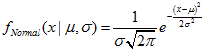```MathProbabilityDensityNormal
MathCumulativeDistributionNormal
MathQuantileNormal
MathRandomNormal

```
```dnorm
pnorm
qnorm
rnorm
```
2 β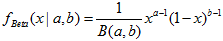```MathProbabilityDensityBeta
MathCumulativeDistributionBeta
MathQuantileBeta
MathRandomBeta

```
```dbeta
pbeta
qbeta
rbeta
```
3 二项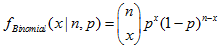```MathProbabilityDensityBinomial
MathCumulativeDistributionBinomial
MathQuantileBinomial
MathRandomBinomial

```
```dbinom
pbinom
qbinom
rbinom
```
60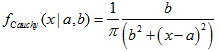```MathProbabilityDensityCauchy
MathCumulativeDistributionCauchy
MathQuantileCauchy
MathRandomCauchy

```
```dcauchy
pcauchy
qcauchy
rcauchy
```
5 卡方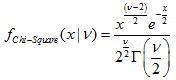```MathProbabilityDensityChiSquare
MathCumulativeDistributionChiSquare
MathQuantileChiSquare
MathRandomChiSquare

```
```dchisq
pchisq
qchisq
rchisq
```
6 指数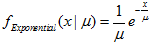```MathProbabilityDensityExponential
MathCumulativeDistributionExponential
MathQuantileExponential
MathRandomExponential

```
```dexp
pexp
qexp
rexp
```
7 F-分布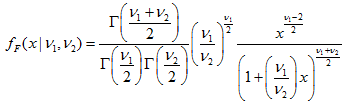```MathProbabilityDensityF
MathCumulativeDistributionF
MathQuantileF
MathRandomF

```
```df
pf
qf
rf
```
8 γ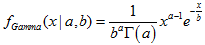```MathProbabilityDensityGamma
MathCumulativeDistributionGamma
MathQuantileGamma
MathRandomGamma

```
```dgamma
pgamma
qgamma
rgamma
```
9 几何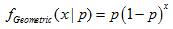```MathProbabilityDensityGeometric
MathCumulativeDistributionGeometric
MathQuantileGeometric
MathRandomGeometric

```
```dgeom
pgeom
qgeom
rgeom
```
10 超几何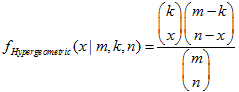```MathProbabilityDensityHypergeometric
MathCumulativeDistributionHypergeometric
MathQuantileHypergeometric
MathRandomHypergeometric

```
```dhyper
phyper
qhyper
rhyper
```
11

```MathProbabilityDensityLogistic
MathCumulativeDistributionLogistic
MathQuantileLogistic
MathRandomLogistic

```
```dlogis
plogis
qlogis
rlogis
```
12 对数正态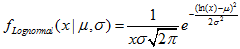```MathProbabilityDensityLognormal
MathCumulativeDistributionLognormal
MathQuantileLognormal
MathRandomLognormal

```
```dlnorm
plnorm
qlnorm
rlnorm
```
13 负二项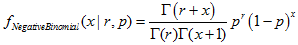```MathProbabilityDensityNegativeBinomial
MathCumulativeDistributionNegativeBinomial
MathQuantileNegativeBinomial
MathRandomNegativeBinomial

```
```dnbinom
pnbinom
qnbinom
rnbinom
```
14 非中心 β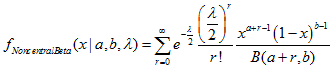```MathProbabilityDensityNoncentralBeta
MathCumulativeDistributionNoncentralBeta
MathQuantileNoncentralBeta
MathRandomNoncentralBeta

```
```dbeta
pbeta
qbeta
rbeta
```
15 非中心卡方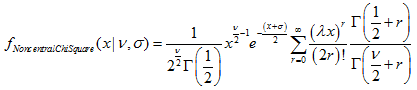```MathProbabilityDensityNoncentralChiSquare
MathCumulativeDistributionNoncentralChiSquare
MathQuantileNoncentralChiSquare
MathRandomNoncentralChiSquare

```
```dchisq
pchisq
qchisq
rchisq
```
16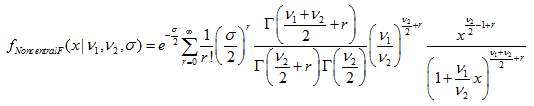```MathProbabilityDensityNoncentralF()
MathCumulativeDistributionNoncentralF()
MathQuantileNoncentralF()
MathRandomNoncentralF()

```
```df
pf
qf
rf
```
17 非中心 t-分布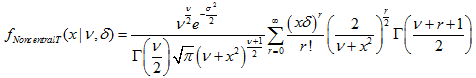```MathProbabilityDensityNoncentralT
MathCumulativeDistributionNoncentralT
MathQuantileNoncentralT
MathRandomNoncentralT

```
```dt
pt
qt
rt
```
18 泊松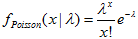```MathProbabilityDensityPoisson
MathCumulativeDistributionPoisson
MathQuantilePoisson
MathRandomPoisson

```
```dpois
ppois
qpois
rpois
```
19 t-分布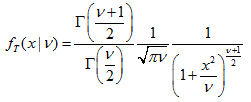```MathProbabilityDensityT
MathCumulativeDistributionT
MathQuantileT
MathRandomT

```
```dt
pt
qt
rt
```
20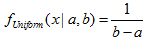```MathProbabilityDensityUniform
MathCumulativeDistributionUniform
MathQuantileUniform
MathRandomUniform

```
```dunif
punif
qunif
runif
```
21 威布尔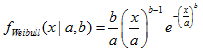```MathProbabilityDensityWeibull
MathCumulativeDistributionWeibull
MathQuantileWeibull
MathRandomWeibull

```
```dweibull
pweibull
qweibull
rweibull
```

MQL5 统计库还提供了用来计算数组的数学函数, 它们列于下表 3。

MQL5
R
生成数值序列
bool MathSequence(const double from,const double to,const double step,double &result[])
bool MathSequenceByCount(const double from,const double to,const int count,double &result[])
bool MathSequence(const int from,const int to,const int step,int &result[])
bool MathSequenceByCount(const int from,const int to,const int count,int &result[])
seq()
生成重复序列
bool MathReplicate(const double &array[],const int count,double &result[])
bool MathReplicate(const int &array[],const int count,int &result[])
rep()
生成逆向元素的数组
bool MathReverse(const double &array[],double &result[])
bool MathReverse(const int &array[],int &result[])
rev(x)
比较数组, 如果所有元素匹配, 则返回 true
bool MathIdentical(const double &array1[],const double &array2[])
bool MathIdentical(const int &array1[],const int &array2[])
identical()
生成含有唯一值的数组
bool MathUnique(const double &array[],double &result[])
bool MathUnique(const int &array[],int &result[])
unique()
根据排序数组元素的顺序生成具有排列的整数数组
bool MathOrder(const double &array[],int &result[])
bool MathOrder(const int &array[],int &result[])
order()
从数组元素生成随机样本。参数 replace=true 允许使用原始序列替换的元素执行随机抽样。数组 probabilities[] 定义了对元素进行抽样的概率。
bool MathSample(const double &array[],const int count,double &result[])
bool MathSample(const double &array[],const int count,const bool replace,double &result[])
bool MathSample(const double &array[],double &probabilities[],const int count,double &result[])
bool MathSample(const double &array[],double &probabilities[],const int count,const bool replace,double &result[])
bool MathSample(const int &array[],const int count,int &result[])
bool MathSample(const int &array[],const int count,const bool replace,int &result[])
bool MathSample(const int &array[],double &probabilities[],const int count,int &result[])
bool MathSample(const int &array[],double &probabilities[],const int count,const bool replace,int &result[])
sample()

返回数组元素合计
double MathSum(const double &array[])
sum()
返回数组元素的乘积
double MathProduct(const double &array[])
prod()
生成累加和的数组
bool MathCumulativeSum(const double &array[],double &result[])
bool MathCumulativeSum(double &array[])
cumsum()
生成累加乘积的数组
bool MathCumulativeProduct(const double &array[],double &result[])
bool MathCumulativeProduct(double &array[])
cumprod()
生成最小值累加数组
bool MathCumulativeMin(const double &array[],double &result[])
bool MathCumulativeMin(double &array[])
cummin()
生成最大值累加数组
bool MathCumulativeMax(const double &array[],double &result[])
bool MathCumulativeMax(double &array[])
cummax()
生成 y[i]=x[i+lag]-x[i] 元素差值数组
bool MathDifference(const double &array[],const int lag,double &result[])
bool MathDifference(const double &array[],const int lag,const int differences,double &result[])
bool MathDifference(const int &array[],const int lag,int &result[])
bool MathDifference(const int &array[],const int lag,const int differences,int &result[])
diff()
返回数组元素最小值
double MathMin(const double &array[])
min()
返回数组元素最大值
double MathMax(const double &array[])
max()
计算数组元素的最小值和最大值
bool MathRange(const double &array[],double &min,double &max)
range()
计算数组元素的平均值
double MathMean(const double &array[])
mean()
计算数组元素的标准偏差
double MathStandardDeviation(const double &array[])
sd()
计算数组元素的中值
double MathMedian(double &array[])
median()
计算数组元素的排位
bool MathRank(const int &array[],double &rank[])
bool MathRank(const double &array[],double &rank[])
rank()
计算Pearson', pearman 和 Kendall 的相关系数
bool MathCorrelationPearson(const double &array1[],const double &array2[],double &r)
bool MathCorrelationPearson(const int &array1[],const int &array2[],double &r)
bool MathCorrelationSpearman(const double &array1[],const double &array2[],double &r)
bool MathCorrelationSpearman(const int &array1[],const int &array2[],double &r)
bool MathCorrelationKendall(const double &array1[],const double &array2[],double &tau)
bool MathCorrelationKendall(const int &array1[],const int &array2[],double &tau)
corr()
计算与指定概率相对应的样本分位数
bool MathQuantile(const double &array[],const double &probs[],double &quantile[])
qunatile()
计算数组元素 Tukey 的五个数字合计 (最小值, 下铰链, 中值, 上铰链, 最大值)
bool MathTukeySummary(const double &array[],const bool removeNAN,double &minimum,double &lower_hinge,double &median,double &upper_hinge,double &maximum)
fivenum()
计算数组元素的对数 (自然数和给定底数)
bool MathLog(const double &array[],double &result[])
bool MathLog(const double &array[],const double base,double &result[])
bool MathLog(double &array[])
bool MathLog(double &array[],const double base)
log()
计算数组元素的以 2 为底的对数
bool MathLog2(const double &array[],double &result[])
bool MathLog2(double &array[])
log2()
计算数组元素的以10 为底的对数
bool MathLog10(const double &array[],double &result[])
bool MathLog10(double &array[])
log10()
计算数组元素的 log(1 + x) 函数值
bool MathLog1p(const double &array[], double &result[])
bool MathLog1p(double &array[])
log1p()
计算数组元素的 exp(x) 函数值
bool MathExp(const double &array[], double &result[])
bool MathExp(double &array[])
exp()
计算数组元素的 ex(x)-1 函数值
bool MathExpm1(const double &array[], double &result[])
bool MathExpm1(double &array[])
expm1()
计算数组元素的 sin(x) 函数值
bool MathSin(const double &array[], double &result[])
bool MathSin(double &array[])
sin()
计算数组元素的 cos(x) 函数值
bool MathCos(const double &array[], double &result[])
bool MathCos(double &array[])
cos()
计算数组元素的 tan(x) 函数值
bool MathTan(const double &array[], double &result[])
bool MathTan(double &array[])
tan()
计算数组元素的 arcsin(x) 函数值
bool MathArcsin(const double &array[], double &result[])
bool MathArcsin(double &array[])
arcsin()
计算数组元素的 arccos(x) 函数值
bool MathArccos(const double &array[], double &result[])
bool MathArccos(double &array[])
arccos()
计算数组元素的 arctan(x) 函数值
bool MathArctan(const double &array[], double &result[])
bool MathArctan(double &array[])
arctan()
计算数组元素的 arctan(y/x) 函数值
bool MathArctan2(const double &array1[], const double &array2[], double &result[])
arctan2()
计算数组元素的 sin(pi*x) 函数值
bool MathSinPi(const double &array[], double &result[])
bool MathSinPi(double &array[])
sinpi()
计算数组元素的 cos(pi*x) 函数值
bool MathCosPi(const double &array[], double &result[])
bool MathCosPi(const double &array[])
cospi()
计算数组元素的 tan(pi*x) 函数值
bool MathTanPi(const double &array[], double &result[])
bool MathTanPi(double &array[])
tanpi()
计算数组元素的绝对值
bool MathAbs(const double &array[], double &result[])
bool MathAbs(double &array[])
abs()
计算数组元素的平方根
bool MathSqrt(const double &array[], double &result[])
bool MathSqrt(double &array[])
sqrt()
返回最接近数组元素的较大整数
bool MathCeil(const double &array[], double &result[])
bool MathCeil(double &array[])
ceil()
返回最接近数组元素的较小整数
bool MathFloor(const double &array[], double &result[])
bool MathFloor(double &array[])
floor()
计算数组元素的整数部分
bool MathTrunc(const double &array[], const int digits, double &result[])
bool MathTrunc(double &array[])
trunc()
计算数组元素的舍入值
bool MathRound(const double &array[], const int digits, double &result[])
bool MathRound(double &array[],int digits)
round()
对于数组元素, 计算指定尾数位数的四舍五入值
bool MathSignif(const double &array[], const int digits, double &result[])
bool MathSignif(double &array[], const int digits)
signinf()
计算数组元素的 sinh(x) 函数值
bool MathSinh(const double &array[],double &result[])
bool MathSinh(double &array[])
sinh()
计算数组元素的 cosh(x) 函数值
bool MathCosh(const double &array[],double &result[])
bool MathCosh(double &array[])
cosh()
计算数组元素的 tanh(x) 函数值
bool MathTanh(const double &array[],double &result[])
bool MathTanh(double &array[])
tanh()
计算数组元素的 arcsinh(x) 函数值
bool MathArcsinh(const double &array[],double &result[])
bool MathArcsinh(double &array[])
asinh()
计算数组元素的 arccosh(x) 函数值
bool MathArccosh(const double &array[],double &result[])
bool MathArccosh(double &array[])
acosh()
计算数组元素的 arctanh(x) 函数值
bool MathArctanh(const double &array[],double &result[])
bool MathArctanh(double &array[])
atanh()
计算数组元素的按位取非运算结果
bool MathBitwiseNot(const int &array[],int &result[])
bool MathBitwiseNot(int &array[])
bitwNot()
计算数组元素的逻辑与运算结果
bool MathBitwiseAnd(const int &array1[],const int &array2[],int &result[])
bitwAnd()
计算数组元素的逻辑或运算结果
bool MathBitwiseOr(const int &array1[],const int &array2[],int &result[])
bitwOr()
计算数组元素的逻辑异或运算结果
bool MathBitwiseXor(const int &array1[],const int &array2[],int &result[])
bitwXor()
计算数组元素的按位左移运算结果
bool MathBitwiseShiftL(const int &array[],const int n,int &result[])
bool MathBitwiseShiftL(int &array[],const int n)
bitwShiftL()
计算数组元素的按位右移运算结果
bool MathBitwiseShiftR(const int &array[],const int n,int &result[])
bool MathBitwiseShiftR(int &array[],const int n)
bitwShiftR()

### 4. 函数使用例程

1. 根据参数为 mu 和 sigma 的正态定律分布, 计算落于 [mu-sigma,mu+sigma] 范围内的随机变量概率。
2. 根据参数为 mu 和 sigma 的正态定律分布, 搜索随机变量 x 的数值范围, 其与 mu 对称并且对应于 95％的置信率。
3. 生成 1000000 个随机数, 根据参数为 mu 和 sigma 的正态定律分布, 计算所得值的直方图, 前 4 个力矩, 并与理论值进行比较。

```//+------------------------------------------------------------------+
//|                                                NormalExample.mq5 |
//|                                 版权所有 2016, MetaQuotes 软件公司|
//|                                             https://www.mql5.com |
//+------------------------------------------------------------------+
#property copyright "版权所有 2016, MetaQuotes 软件公司"
#property version   "1.00"
//--- 包含用于计算正态分布的函数
#include <Math\Stat\Normal.mqh>
//+------------------------------------------------------------------+
//| 计算直方图                                                        |
//+------------------------------------------------------------------+
void CalculateHistogram(double &data[],const int ncells=200,const string filename="normal.csv")
{
if(ArraySize(data)<=0)
return;

int n=ArraySize(data);
//--- 在 'data' 数组里搜索最小值和最大值
double minv,maxv,range;
minv=data;
maxv=data;
for(int i=1; i<n; i++)
{
minv=MathMin(minv,data[i]);
maxv=MathMax(maxv,data[i]);
}
//--- 计算范围
range=maxv-minv;
//   Print("最小=",minv," 最大=",maxv," 范围=",range," 大小=",n);
if(range==0)
return;
//--- 直方图计算数组
double x[];
double y[];
//--- 设置直方图值
ArrayResize(x,ncells);
ArrayResize(y,ncells);
for(int i=0; i<ncells; i++)
{
x[i]=minv+i*range/(ncells-1);
y[i]=0;
}
//--- 计算直方图
for(int i=0; i<n; i++)
{
double v=(maxv-data[i])/range;
int ind=int((v*(ncells-1)));
y[ind]++;
}
//--- 检查文件名
if(filename=="")
return;
//--- 打开文件写入
ResetLastError();
int filehandle=FileOpen(filename,FILE_WRITE|FILE_TXT|FILE_ANSI);
//--- 数据写入文件
if(filehandle!=INVALID_HANDLE)
{
for(int i=0; i<ncells; i++)
{
string str=StringFormat("%6.20f;%6.20f",x[i],y[i]);
FileWrite(filehandle,str);
}
FileClose(filehandle);
PrintFormat("直方图保存于文件 %s",filename);
}
else
PrintFormat("调用 FileOpen 错误, 错误代码=%d",GetLastError());
}
//+------------------------------------------------------------------+
//| 脚本程序开始函数                                                  |
//+------------------------------------------------------------------+
void OnStart()
{
//--- 1. 计算随机变量概率,
//--- 根据参数为 mu 和 sigma 的正态定律分布, 落于 [mu-sigma,mu+sigma] 范围
//--- 设置分布参数
double mu=5.0;
double sigma=1.0;
//--- 设置间隔
double x1=mu-sigma;
double x2=mu+sigma;
//--- 概率计算变量
double cdf1,cdf2,probability;
//--- 错误代码变量
int error_code1,error_code2;
//--- 计算分布函数值
cdf1=MathCumulativeDistributionNormal(x1,mu,sigma,error_code1);
cdf2=MathCumulativeDistributionNormal(x2,mu,sigma,error_code2);
//--- 检查错误代码
if(error_code1==ERR_OK && error_code2==ERR_OK)
{
//--- 计算概率
probability=cdf2-cdf1;
//--- 输出结果
PrintFormat("x1=%5.8f, x2=%5.8f, 概率=%5.8f",x1,x2,probability);
}
//--- 2. 根据参数为 mu 和 sigma 的正态定律分布, 搜索随机变量范围值,
//--- 其与 mu 对称, 且对应于 95% 的置信率。
//--- 设置置信率
probability=0.95;
//--- 在间隔界限处设置概率
double p1=(1.0-probability)*0.5;
double p2=probability+(1.0-probability)*0.5;
//--- 计算间隔界限
x1=MathQuantileNormal(p1,mu,sigma,error_code1);
x2=MathQuantileNormal(p2,mu,sigma,error_code2);
//--- 检查错误代码
if(error_code1==ERR_OK && error_code2==ERR_OK)
{
//--- 输出结果
PrintFormat("x1=%5.8f, x2=%5.8f",x1,x2);
}
//--- 3. 根据参数为 mu 和 sigma 的正态定律分布, 生成 1000000 个随机数,
//--- 在所获数值上计算直方图, 前 4 个力矩并, 与理论值比较
//--- 设置数值数量并准备数组
int data_count=1000000;
double data[];
ArrayResize(data,data_count);
//--- 生成随机值并将它们保存到数组
for(int i=0; i<data_count; i++)
{
data[i]=MathRandomNormal(mu,sigma,error_code1);
}
//--- 设置初始值索引, 以及用于计算的数据量
int start=0;
int count=data_count;
//--- 计算所生成数值的前 4 个力矩
double mean=MathMean(data,start,count);
double variance=MathVariance(data,start,count);
double skewness=MathSkewness(data,start,count);
double kurtosis=MathKurtosis(data,start,count);
//--- 理论力矩的变量
double normal_mean=0;
double normal_variance=0;
double normal_skewness=0;
double normal_kurtosis=0;
//--- 显示计算的力矩值
PrintFormat("              均值=%.10f,         方差=%.10f         偏度=%.10f         峰度=%.10f",mean,variance,skewness,kurtosis);
//--- 计算力矩的理论值, 并将它们与所获值比较
if(MathMomentsNormal(mu,sigma,normal_mean,normal_variance,normal_skewness,normal_kurtosis,error_code1))
{
PrintFormat("正态均值=%.10f,  正态方差=%.10f  正态偏度=%.10f  正态峰度=%.10f",normal_mean,normal_variance,normal_skewness,normal_kurtosis);
PrintFormat("增量均值=%.4f, 增量方差=%.4f  增量偏度=%.4f  增量峰度=%.4f",mean-normal_mean,variance-normal_variance,skewness-normal_skewness,kurtosis-normal_kurtosis);
}
//--- 计算分布直方图, 并将其保存到 normal.csv 文件
int ncells=50;
CalculateHistogram(data,ncells,"normal.csv");
}

```

Script execution results: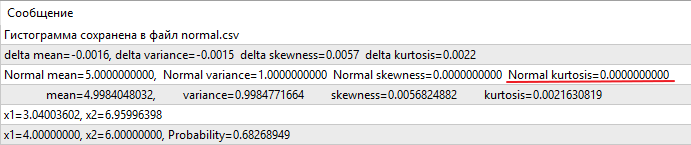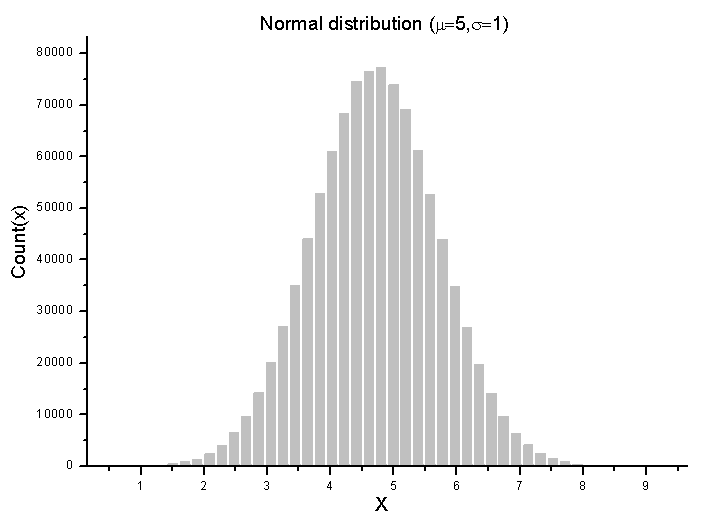### 5. 计算速度比较

MQL5 计算
PDF 时间 (µs)
R time for calculating
PDF 时间 (µs)
PDF
R/MQL5
MQL5 计算
CDF 时间 (µs)
R time for calculating
CDF 时间 (µs)
CDF
R/MQL5
MQL5 time for calculating

R time for calculating

Quantile
R/MQL5
MQL5 time for generating

R time for generating
random numbers (µs)
Random
R/MQL5
1
二项
4.39
11.663
2.657
13.65
25.316
1.855
50.18
66.845
1.332
318.73
1816.463
5.699
2
β
1.74
17.352
9.972
4.76
15.076
3.167
48.72
129.992
2.668
688.81
1723.45
2.502
3
γ
1.31
8.251
6.347
8.09
14.792
1.828
50.83
64.286
1.265
142.84
1281.707
8.973
60
柯西
0.45
1.423
3.162
1.33
15.078
11.34
1.37
2.845
2.077
224.19
588.517
2.625
5
指数
0.85
3.13
3.682
0.77
2.845
3.695
0.53
2.276
4.294
143.18
389.406
2.72
6
均匀
0.42
2.561
6.098
0.45
1.423
3.162
0.18
2.846
15.81
40.3
247.467
6.141
7
几何
2.3
5.121
2.227
2.12.
4.552
2.147
0.81
5.407
6.675
278
1078.045
3.879
8
超几何
1.85 11.095
5.997
0.9
8.819
9.799
0.75
9.957
13.28
302.55
880.356
2.91
9
逻辑斯谛
1.27
4.267
3.36
1.11
4.267
3.844
0.71
3.13
4.408
178.65
626.632
3.508
10
威布尔
2.99
5.69
1.903
2.74
4.268
1.558
2.64
6.828
2.586
536.37
1558.472
2.906
11
泊松
2.91
5.974
2.053
6.26
8.534
1.363
3.43
13.085
3.815
153.59
303.219
1.974
12
F
3.86
10.241
2.653
9.94
22.472
2.261
65.47
135.396
2.068
1249.22
1801.955
1.442
13
卡方
2.47
5.974
2.419
7.71
13.37
1.734
44.11
61.725
1.399
210.24
1235.059
5.875
14
非中心卡方
8.05
14.223
1.767
45.61
209.068
4.584
220.66
10342.96
46.873
744.45
1997.653
2.683
15
非中心 F
19.1
28.446
1.489
14.67
46.935
3.199
212.21
2561.991
12.073
1848.9
2912.141
1.575
16
非中心 β
16.3
26.739
1.64
10.48
43.237
4.126
153.66
2290.915
14.909
2686.82
2839.893
1.057
17
负二项
6.13
11.094
1.81
12.21
19.627
1.607
14.05
60.019
4.272
1130.39
1936.498
1.713
18
正态
1.15
4.267
3.71
0.81
3.983
4.917
0.7
2.277
3.253
293.7
696.321
2.371
19
对数正态
1.99
5.406
2.717
3.19
8.819
2.765
3.18
6.259
1.968
479.75
1269.761
2.647
20
T
2.32
11.663
5.027
8.01
19.059
2.379
50.23
58.596
1.167
951.58
1425.92
1.498
21
非中心 T
38.47
86.757
2.255
27.75
39.823
1.435
1339.51
1930.524
1.441
1550.27
1699.84
1.096
<PDF R/MQL5>
3.474     <CDF R/MQL5>
3.465
<Quantile R/MQL5>
7.03
<Random R/MQL5>
3.13

Table 4. R 语言和 MQL5 中的统计函数计算时间 (单位微秒)

R 语言取用最小时间值, 对于 MQL5 取用了平均值 (pdf_mean, cdf_mean, quantile_mean, random_mean)。

### 6. 已检测到的 R 语言中的计算误差

```> n <- 10
> k <- seq(0,1,by=1/n)
> nt_pdf<-dt(k, 10,8, log = FALSE)
> nt_cdf<-pt(k, 10,8, log = FALSE)
> nt_quantile<-qt(nt_cdf, 10,8, log = FALSE)
> nt_pdf
 4.927733e-15 1.130226e-14 2.641608e-14 6.281015e-14 1.516342e-13 3.708688e-13 9.166299e-13
 2.283319e-12 5.716198e-12 1.433893e-11 3.593699e-11
> nt_cdf
 6.220961e-16 1.388760e-15 3.166372e-15 7.362630e-15 1.742915e-14 4.191776e-14 1.021850e-13
 2.518433e-13 6.257956e-13 1.563360e-12 3.914610e-12
> k
 0.0 0.1 0.2 0.3 0.4 0.5 0.6 0.7 0.8 0.9 1.0
> nt_quantile
           -Inf -1.340781e+154 -1.340781e+154 -1.340781e+154 -1.340781e+154 -1.340781e+154
 -1.340781e+154   7.000000e-01   8.000000e-01   9.000000e-01   1.000000e+00```

MQL5 统计库使用文献  中提出的正确算法来计算概率, 这提供了准确的结果。

```> dgamma(0,0.5,1)
 Inf
> dchisq(0,df=0.5,ncp=1)
 Inf
> dchisq(0,df=0.5,ncp=0)
 Inf
```

```> pgamma(0.1,0.5,1)
 0.3452792
> pchisq(0.1,df=0.5,ncp=0)
 0.5165553
> pchisq(0.1,df=0.5,ncp=1)
 0.3194965```

Wolfram Alpha (数值) 和 Matlab 在点 point x=0 出的密度定义不一样: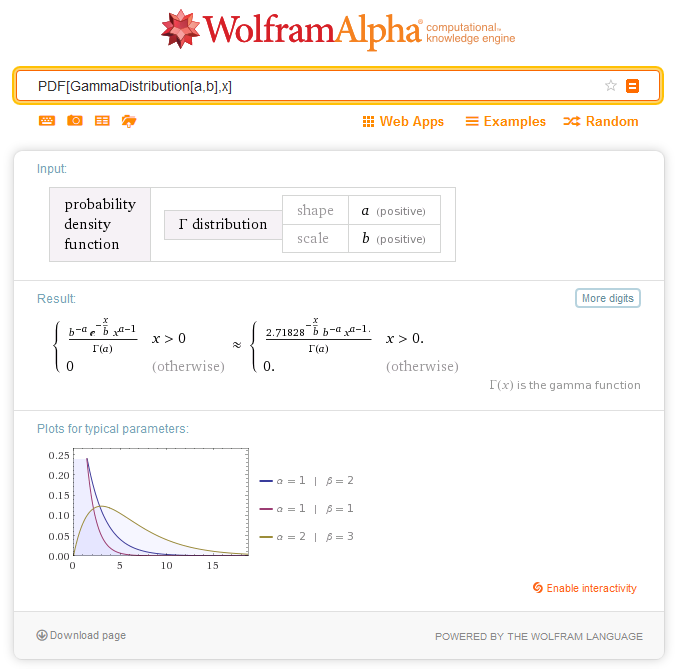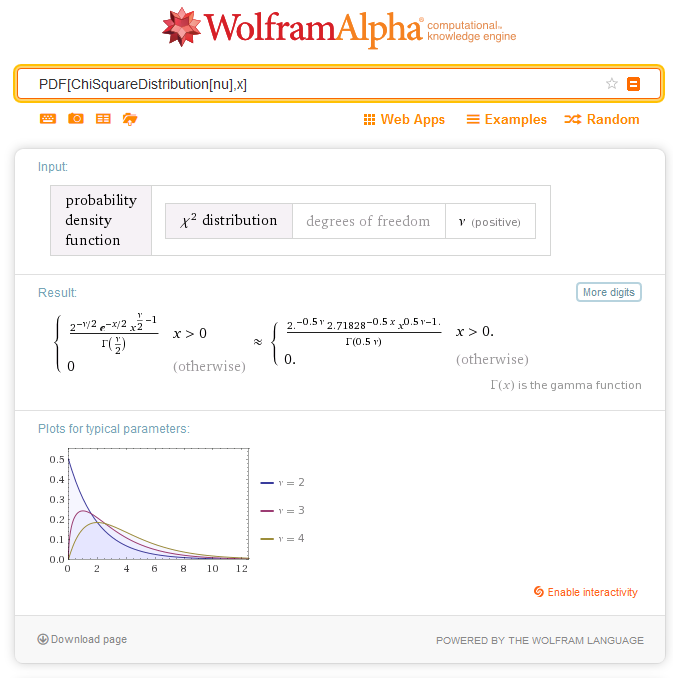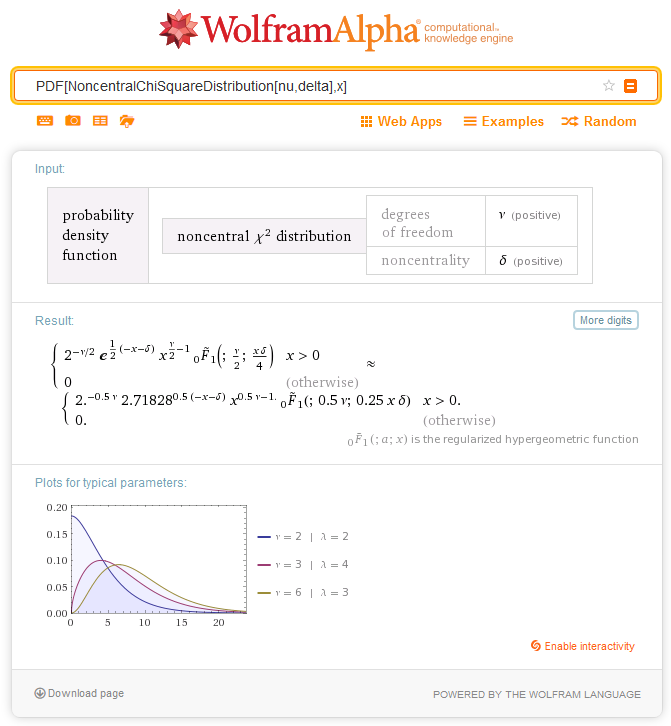### 参考文献

1. 用于统计计算的 R 项目
2. N. Balakrishnan, N. L. Johnson, S. Kotz  "Continuous Univariate Distributions: Volume 1." Wiley-Interscience, 1994.
3. N. Balakrishnan, N. L. Johnson, S. Kotz  "Continuous Univariate Distributions: Volume 2." Wiley-Interscience, 2000.
4. N. L. Johnson, S. Kotz, A. W. Kemp  "Univariate Discrete Distributions", Wiley-Interscience, 2005.
5. Forbes C., Evans M., Hastings N., Peacock B., "Statistical Distributions", 4th Edition, John Wiley and Sons, 2011.
6. Lenth, R.V., "Cumulative distribution function of the noncentral t distribution", Appled Statistics,  vol. 38 (1989), 185–189.
7. D. Benton, K. Krishnamoorthy, "Computing discrete mixtures of continuous distributions: noncentral chisquare, noncentral t and the distribution of the square of the sample multiple correlation coefficient",  Computational Statistics & Data Analysis, 43, (2003), 249-267

### 附录。统计函数计算时间的测量结果

R 语言脚本:

library(microbenchmark)
n <- 50
k <- seq(0,n,by=1)
binomial_pdf <- microbenchmark(pdf<-dbinom(k, 50, 0.6, log = FALSE))
binomial_cdf <- microbenchmark(cdf<-pbinom(k, 50, 0.6, log = FALSE))
binomial_quantile <- microbenchmark(quantile<-qbinom(cdf, 50, 0.6, log = FALSE))
binomial_random <- microbenchmark(random<-rbinom(10000, 50, 0.6))
print(binomial_pdf)
print(binomial_cdf)
print(binomial_quantile)
print(binomial_random)

n <- 50
k <- seq(0,1,by=1/n)
beta_pdf <- microbenchmark(pdf<-dbeta(k, 2, 4, log = FALSE))
beta_cdf <- microbenchmark(cdf<-pbeta(k, 2, 4, log = FALSE))
beta_quantile <- microbenchmark(quantile<-qbeta(cdf, 2, 4, log = FALSE))
beta_random <- microbenchmark(random<-rbeta(10000, 2, 4,))
print(beta_pdf)
print(beta_cdf)
print(beta_quantile)
print(beta_random)

n <- 50
k <- seq(0,1,by=1/n)
gamma_pdf <- microbenchmark(pdf<-dgamma(k, 1,1, log = FALSE))
gamma_cdf <- microbenchmark(cdf<-pgamma(k, 1,1, log = FALSE))
gamma_quantile <- microbenchmark(quantile<-qgamma(cdf, 1, 1, log = FALSE))
gamma_random <- microbenchmark(random<-rgamma(10000, 1,1))
print(gamma_pdf)
print(gamma_cdf)
print(gamma_quantile)
print(gamma_random)

n <- 50
k <- seq(0,1,by=1/n)
cauchy_pdf <- microbenchmark(pdf<-dcauchy(k, 2,1, log = FALSE))
cauchy_cdf <- microbenchmark(cdf<-pgamma(k, 2,1, log = FALSE))
cauchy_quantile <- microbenchmark(quantile<-qcauchy(cdf, 2, 1, log = FALSE))
cauchy_random <- microbenchmark(random<-rcauchy(10000, 2, 1))
print(cauchy_pdf)
print(cauchy_cdf)
print(cauchy_quantile)
print(cauchy_random)

n <- 50
k <- seq(0,1,by=1/n)
exponential_pdf <- microbenchmark(pdf<-dexp(k, 2, log = FALSE))
exponential_cdf <- microbenchmark(cdf<-pexp(k, 2, log = FALSE))
exponential_quantile <- microbenchmark(quantile<-qexp(cdf, 2, log = FALSE))
exponential_random <- microbenchmark(random<-rexp(10000, 2))
print(exponential_pdf)
print(exponential_cdf)
print(exponential_quantile)
print(exponential_random)

n <- 50
k <- seq(0,1,by=1/n)
uniform_pdf <- microbenchmark(pdf<-dunif(k, 0, 10, log = FALSE))
uniform_cdf <- microbenchmark(cdf<-punif(k, 0, 10, log = FALSE))
uniform_quantile <- microbenchmark(quantile<-qunif(cdf, 0, 10, log = FALSE))
uniform_random <- microbenchmark(random<-runif(10000, 0, 10))
print(uniform_pdf)
print(uniform_cdf)
print(uniform_quantile)
print(uniform_random)

n <- 50
k <- seq(0,n,by=1)
geometric_pdf <- microbenchmark(pdf<-dgeom(k, 0.3, log = FALSE))
geometric_cdf <- microbenchmark(cdf<-pgeom(k, 0.3, log = FALSE))
geometric_quantile <- microbenchmark(quantile<-qgeom(cdf, 0.3, log = FALSE))
geometric_random <- microbenchmark(random<-rgeom(10000, 0.3))
print(geometric_pdf)
print(geometric_cdf)
print(geometric_quantile)
print(geometric_random)

n <- 50
k <- seq(0,n,by=1)
hypergeometric_pdf <- microbenchmark(pdf<-dhyper(k, 12,38,11, log = FALSE))
hypergeometric_cdf <- microbenchmark(cdf<-phyper(k, 12,38,11, log = FALSE))
hypergeometric_quantile <- microbenchmark(quantile<-qhyper(cdf, 12,38,11, log = FALSE))
hypergeometric_random <- microbenchmark(random<-rhyper(10000, 12,38,11))
print(hypergeometric_pdf)
print(hypergeometric_cdf)
print(hypergeometric_quantile)
print(hypergeometric_random)

n <- 50
k <- seq(0,1,by=1/n)
logistic_pdf <- microbenchmark(pdf<-dlogis(k, 1,2, log = FALSE))
logistic_cdf <- microbenchmark(cdf<-plogis(k, 1,2, log = FALSE))
logistic_quantile <- microbenchmark(quantile<-qlogis(cdf, 1,2, log = FALSE))
logistic_random <- microbenchmark(random<-rlogis(10000, 1,2))
print(logistic_pdf)
print(logistic_cdf)
print(logistic_quantile)
print(logistic_random)

n <- 50
k <- seq(0,1,by=1/n)
weibull_pdf <- microbenchmark(pdf<-dweibull(k, 5,1, log = FALSE))
weibull_cdf <- microbenchmark(cdf<-pweibull(k, 5,1, log = FALSE))
weibull_quantile <- microbenchmark(quantile<-qweibull(cdf, 5,1, log = FALSE))
weibull_random <- microbenchmark(random<-rweibull(10000, 5,1))
print(weibull_pdf)
print(weibull_cdf)
print(weibull_quantile)
print(weibull_random)

n <- 50
k <- seq(0,n,by=1)
poisson_pdf <- microbenchmark(pdf<-dpois(k, 1, log = FALSE))
poisson_cdf <- microbenchmark(cdf<-ppois(k, 1, log = FALSE))
poisson_quantile <- microbenchmark(quantile<-qpois(cdf, 1, log = FALSE))
poisson_random <- microbenchmark(random<-rpois(10000, 1))
print(poisson_pdf)
print(poisson_cdf)
print(poisson_quantile)
print(poisson_random)

n <- 50
k <- seq(0,1,by=1/n)
f_pdf <- microbenchmark(pdf<-df(k, 10,20, log = FALSE))
f_cdf <- microbenchmark(cdf<-pf(k, 10,20, log = FALSE))
f_quantile <- microbenchmark(quantile<-qf(cdf, 10,20,log = FALSE))
f_random <- microbenchmark(random<-rf(10000, 10,20))
print(f_pdf)
print(f_cdf)
print(f_quantile)
print(f_random)

n <- 50
k <- seq(0,1,by=1/n)
chisquare_pdf <- microbenchmark(pdf<-dchisq(k, 2,log = FALSE))
chisquare_cdf <- microbenchmark(cdf<-pchisq(k, 2, log = FALSE))
chisquare_quantile <- microbenchmark(quantile<-qchisq(cdf, 2, log = FALSE))
chisquare_random <- microbenchmark(random<-rchisq(10000, 2))
print(chisquare_pdf)
print(chisquare_cdf)
print(chisquare_quantile)
print(chisquare_random)

n <- 50
k <- seq(0,1,by=1/n)
nchisquare_pdf <- microbenchmark(pdf<-dchisq(k, 2,1, log = FALSE))
nchisquare_cdf <- microbenchmark(cdf<-pchisq(k, 2,1,log = FALSE))
nchisquare_quantile <- microbenchmark(quantile<-qchisq(cdf, 2,1, log = FALSE))
nchisquare_random <- microbenchmark(random<-rchisq(10000, 2,1))
print(nchisquare_pdf)
print(nchisquare_cdf)
print(nchisquare_quantile)
print(nchisquare_random)

n <- 50
k <- seq(0,1,by=1/n)
nf_pdf <- microbenchmark(pdf<-df(k, 10,20,2, log = FALSE))
nf_cdf <- microbenchmark(cdf<-pf(k, 10,20,2, log = FALSE))
nf_quantile <- microbenchmark(quantile<-qf(cdf, 10,20,2, log = FALSE))
nf_random <- microbenchmark(random<-rf(10000, 10,20,2))
print(nf_pdf)
print(nf_cdf)
print(nf_quantile)
print(nf_random)

n <- 50
k <- seq(0,1,by=1/n)
nbeta_pdf <- microbenchmark(pdf<-dbeta(k, 2,4,1, log = FALSE))
nbeta_cdf <- microbenchmark(cdf<-pbeta(k, 2,4,1, log = FALSE))
nbeta_quantile <- microbenchmark(quantile<-qbeta(cdf, 2,4,1, log = FALSE))
nbeta_random <- microbenchmark(random<-rbeta(10000, 2,4,1))
print(nbeta_pdf)
print(nbeta_cdf)
print(nbeta_quantile)
print(nbeta_random)

n <- 50
k <- seq(0,n,by=1)
nbinom_pdf <- microbenchmark(pdf<-dnbinom(k, 2, 0.5, log = FALSE))
nbinom_cdf <- microbenchmark(cdf<-pnbinom(k, 2, 0.5, log = FALSE))
nbinom_quantile <- microbenchmark(quantile<-qnbinom(cdf, 2, 0.5, log = FALSE))
nbinom_random <- microbenchmark(random<-rnbinom(10000, 2, 0.5))
print(nbinom_pdf)
print(nbinom_cdf)
print(nbinom_quantile)
print(nbinom_random)

n <- 50
k <- seq(0,1,by=1/n)
normal_pdf <- microbenchmark(pdf<-dnorm(k, 1, 1, log = FALSE))
normal_cdf <- microbenchmark(cdf<-pnorm(k, 1, 1, log = FALSE))
normal_quantile <- microbenchmark(quantile<-qnorm(cdf, 1,1, log = FALSE))
normal_random <- microbenchmark(random<-rnorm(10000, 1,1))
print(normal_pdf)
print(normal_cdf)
print(normal_quantile)
print(normal_random)

n <- 50
k <- seq(0,1,by=1/n)
lognormal_pdf <- microbenchmark(pdf<-dlnorm(k, 0.5,0.6, log = FALSE))
lognormal_cdf <- microbenchmark(cdf<-plnorm(k, 0.5,0.6, log = FALSE))
lognormal_quantile <- microbenchmark(quantile<-qlnorm(cdf, 0.5,0.6, log = FALSE))
lognormal_random <- microbenchmark(random<-rlnorm(10000, 0.5,0.6))
print(lognormal_pdf)
print(lognormal_cdf)
print(lognormal_quantile)
print(lognormal_random)

n <- 50
k <- seq(0,1,by=1/n)
t_pdf <- microbenchmark(pdf<-dt(k, 8, log = FALSE))
t_cdf <- microbenchmark(cdf<-pt(k, 8, log = FALSE))
t_quantile <- microbenchmark(quantile<-qt(cdf, 8, log = FALSE))
t_random <- microbenchmark(random<-rt(10000, 8))
print(t_pdf)
print(t_cdf)
print(t_quantile)
print(t_random)

n <- 50
k <- seq(0,1,by=1/n)
nt_pdf <- microbenchmark(pdf<-dt(k, 10,1, log = FALSE))
nt_cdf <- microbenchmark(cdf<-pt(k, 10,1, log = FALSE))
nt_quantile <- microbenchmark(quantile<-qt(cdf, 10,1, log = FALSE))
nt_random <- microbenchmark(random<-rt(10000, 10,1))
print(nt_pdf)
print(nt_cdf)
print(nt_quantile)
print(nt_random)

Result:

```R version 3.2.5 (2016-04-14) -- "Very, Very Secure Dishes"
Copyright (C) 2016 The R Foundation for Statistical Computing
Platform: x86_64-w64-mingw32/x64 (64-bit)

R is free software and comes with ABSOLUTELY NO WARRANTY.
You are welcome to redistribute it under certain conditions.
Type 'license()' or 'licence()' for distribution details.

R is a collaborative project with many contributors.
'citation()' on how to cite R or R packages in publications.

Type 'demo()' for some demos, 'help()' for on-line help, or
'help.start()' for an HTML browser interface to help.
Type 'q()' to quit R.

> library(microbenchmark)
> n <- 50
> k <- seq(0,n,by=1)
> binomial_pdf <- microbenchmark(pdf<-dbinom(k, 50, 0.6, log = FALSE))
> binomial_cdf <- microbenchmark(cdf<-pbinom(k, 50, 0.6, log = FALSE))
> binomial_quantile <- microbenchmark(quantile<-qbinom(cdf, 50, 0.6, log = FALSE))
> binomial_random <- microbenchmark(random<-rbinom(10000, 50, 0.6))
> print(binomial_pdf)
Unit: microseconds
expr    min     lq     mean median     uq    max neval
pdf <- dbinom(k, 50, 0.6, log = FALSE) 11.663 11.948 13.37888 12.233 12.233 47.503   100
> print(binomial_cdf)
Unit: microseconds
expr    min     lq     mean median     uq    max neval
cdf <- pbinom(k, 50, 0.6, log = FALSE) 25.316 25.602 29.63195 25.886 35.557 93.868   100
> print(binomial_quantile)
Unit: microseconds
expr    min    lq     mean median     uq     max neval
quantile <- qbinom(cdf, 50, 0.6, log = FALSE) 66.845 67.13 72.09098 67.699 73.672 130.276   100
> print(binomial_random)
Unit: milliseconds
expr      min      lq     mean   median       uq      max neval
random <- rbinom(10000, 50, 0.6) 1.816463 1.89056 1.948185 1.929814 1.989262 2.308835   100
>
>
> n <- 50
> k <- seq(0,1,by=1/n)
> beta_pdf <- microbenchmark(pdf<-dbeta(k, 2, 4, log = FALSE))
> beta_cdf <- microbenchmark(cdf<-pbeta(k, 2, 4, log = FALSE))
> beta_quantile <- microbenchmark(quantile<-qbeta(cdf, 2, 4, log = FALSE))
> beta_random <- microbenchmark(random<-rbeta(10000, 2, 4,))
> print(beta_pdf)
Unit: microseconds
expr    min     lq     mean median     uq     max neval
pdf <- dbeta(k, 2, 4, log = FALSE) 17.352 17.637 19.99512 17.638 18.206 109.797   100
> print(beta_cdf)
Unit: microseconds
expr    min     lq     mean median      uq    max neval
cdf <- pbeta(k, 2, 4, log = FALSE) 15.076 15.361 16.83489 15.646 15.9295 75.379   100
> print(beta_quantile)
Unit: microseconds
expr     min      lq     mean   median     uq     max neval
quantile <- qbeta(cdf, 2, 4, log = FALSE) 129.992 130.277 140.8325 131.4145 143.93 201.672   100
> print(beta_random)
Unit: milliseconds
expr     min       lq     mean   median       uq      max neval
random <- rbeta(10000, 2, 4, ) 1.72345 1.794132 1.862292 1.836515 1.901226 2.823963   100
>
> n <- 50
> k <- seq(0,1,by=1/n)
> gamma_pdf <- microbenchmark(pdf<-dgamma(k, 1,1, log = FALSE))
> gamma_cdf <- microbenchmark(cdf<-pgamma(k, 1,1, log = FALSE))
> gamma_quantile <- microbenchmark(quantile<-qgamma(cdf, 1, 1, log = FALSE))
> gamma_random <- microbenchmark(random<-rgamma(10000, 1,1))
> print(gamma_pdf)
Unit: microseconds
expr   min     lq     mean median    uq     max neval
pdf <- dgamma(k, 1, 1, log = FALSE) 8.251 8.8195 10.92684  9.104 9.389 122.312   100
> print(gamma_cdf)
Unit: microseconds
expr    min     lq     mean median     uq     max neval
cdf <- pgamma(k, 1, 1, log = FALSE) 14.792 15.646 20.43306 20.055 21.334 106.099   100
> print(gamma_quantile)
Unit: microseconds
expr    min     lq     mean median    uq     max neval
quantile <- qgamma(cdf, 1, 1, log = FALSE) 64.286 64.854 70.09419 65.139 67.13 162.988   100
> print(gamma_random)
Unit: milliseconds
expr      min       lq     mean   median       uq      max neval
random <- rgamma(10000, 1, 1) 1.281707 1.330347 1.410961 1.362631 1.421226 2.322204   100
>
> n <- 50
> k <- seq(0,1,by=1/n)
> cauchy_pdf <- microbenchmark(pdf<-dcauchy(k, 2,1, log = FALSE))
> cauchy_cdf <- microbenchmark(cdf<-pgamma(k, 2,1, log = FALSE))
> cauchy_quantile <- microbenchmark(quantile<-qcauchy(cdf, 2, 1, log = FALSE))
> cauchy_random <- microbenchmark(random<-rcauchy(10000, 2, 1))
> print(cauchy_pdf)
Unit: microseconds
expr   min    lq   mean median    uq    max neval
pdf <- dcauchy(k, 2, 1, log = FALSE) 1.423 1.708 2.8431  1.709 2.278 67.415   100
> print(cauchy_cdf)
Unit: microseconds
expr    min     lq     mean median     uq    max neval
cdf <- pgamma(k, 2, 1, log = FALSE) 15.078 15.646 16.51914  15.93 16.215 33.281   100
> print(cauchy_quantile)
Unit: microseconds
expr   min   lq   mean median    uq    max neval
quantile <- qcauchy(cdf, 2, 1, log = FALSE) 2.845 3.13 3.8044  3.131 3.415 56.606   100
> print(cauchy_random)
Unit: microseconds
expr     min      lq     mean   median      uq      max neval
random <- rcauchy(10000, 2, 1) 588.517 615.823 663.8658 637.7255 674.845 1520.356   100
>
> n <- 50
> k <- seq(0,1,by=1/n)
> exponential_pdf <- microbenchmark(pdf<-dexp(k, 2, log = FALSE))
> exponential_cdf <- microbenchmark(cdf<-pexp(k, 2, log = FALSE))
> exponential_quantile <- microbenchmark(quantile<-qexp(cdf, 2, log = FALSE))
> exponential_random <- microbenchmark(random<-rexp(10000, 2))
> print(exponential_pdf)
Unit: microseconds
expr  min    lq    mean median    uq    max neval
pdf <- dexp(k, 2, log = FALSE) 3.13 3.414 4.08887  3.415 3.415 67.699   100
> print(exponential_cdf)
Unit: microseconds
expr   min   lq    mean median     uq    max neval
cdf <- pexp(k, 2, log = FALSE) 2.845 3.13 4.38756  3.414 3.5565 58.597   100
> print(exponential_quantile)
Unit: microseconds
expr   min    lq    mean median    uq    max neval
quantile <- qexp(cdf, 2, log = FALSE) 2.276 2.561 3.80729 2.5615 2.846 44.659   100
> print(exponential_random)
Unit: microseconds
expr     min       lq     mean   median       uq      max neval
random <- rexp(10000, 2) 389.406 408.8895 440.9583 418.4185 444.8715 1371.875   100
>
>
> n <- 50
> k <- seq(0,1,by=1/n)
> uniform_pdf <- microbenchmark(pdf<-dunif(k, 0, 10, log = FALSE))
> uniform_cdf <- microbenchmark(cdf<-punif(k, 0, 10, log = FALSE))
> uniform_quantile <- microbenchmark(quantile<-qunif(cdf, 0, 10, log = FALSE))
> uniform_random <- microbenchmark(random<-runif(10000, 0, 10))
> print(uniform_pdf)
Unit: microseconds
expr   min    lq    mean median    uq    max neval
pdf <- dunif(k, 0, 10, log = FALSE) 2.561 2.846 3.78734   3.13 3.131 66.277   100
> print(uniform_cdf)
Unit: microseconds
expr   min    lq    mean median    uq    max neval
cdf <- punif(k, 0, 10, log = FALSE) 1.423 1.708 2.41635  1.992 1.993 53.477   100
> print(uniform_quantile)
Unit: microseconds
expr   min    lq    mean median     uq    max neval
quantile <- qunif(cdf, 0, 10, log = FALSE) 2.846 3.131 5.13561  3.415 3.6995 82.774   100
> print(uniform_random)
Unit: microseconds
expr     min       lq     mean   median       uq      max neval
random <- runif(10000, 0, 10) 247.467 258.7035 317.1567 279.6095 357.2635 1267.769   100
>
>
> n <- 50
> k <- seq(0,n,by=1)
> geometric_pdf <- microbenchmark(pdf<-dgeom(k, 0.3, log = FALSE))
> geometric_cdf <- microbenchmark(cdf<-pgeom(k, 0.3, log = FALSE))
> geometric_quantile <- microbenchmark(quantile<-qgeom(cdf, 0.3, log = FALSE))
> geometric_random <- microbenchmark(random<-rgeom(10000, 0.3))
> print(geometric_pdf)
Unit: microseconds
expr   min    lq    mean median    uq    max neval
pdf <- dgeom(k, 0.3, log = FALSE) 5.121 5.122 6.14258  5.406 5.406 60.304   100
> print(geometric_cdf)
Unit: microseconds
expr   min    lq    mean median     uq    max neval
cdf <- pgeom(k, 0.3, log = FALSE) 4.552 4.836 5.50548  4.837 5.1215 46.081   100
> print(geometric_quantile)
Unit: microseconds
expr   min    lq    mean median    uq    max neval
quantile <- qgeom(cdf, 0.3, log = FALSE) 5.407 5.974 7.12107  5.975 6.259 71.681   100
> print(geometric_random)
Unit: milliseconds
expr      min       lq     mean   median       uq      max neval
random <- rgeom(10000, 0.3) 1.078045 1.127681 1.192608 1.156125 1.199361 1.604267   100
>
> n <- 50
> k <- seq(0,n,by=1)
> hypergeometric_pdf <- microbenchmark(pdf<-dhyper(k, 12,38,11, log = FALSE))
> hypergeometric_cdf <- microbenchmark(cdf<-phyper(k, 12,38,11, log = FALSE))
> hypergeometric_quantile <- microbenchmark(quantile<-qhyper(cdf, 12,38,11, log = FALSE))
> hypergeometric_random <- microbenchmark(random<-rhyper(10000, 12,38,11))
> print(hypergeometric_pdf)
Unit: microseconds
expr    min     lq     mean median     uq     max neval
pdf <- dhyper(k, 12, 38, 11, log = FALSE) 11.095 13.939 17.07667 14.224 14.935 101.548   100
> print(hypergeometric_cdf)
Unit: microseconds
expr   min    lq     mean median     uq   max neval
cdf <- phyper(k, 12, 38, 11, log = FALSE) 8.819 9.387 12.60515  9.672 12.517 62.01   100
> print(hypergeometric_quantile)
Unit: microseconds
expr   min      lq     mean median     uq    max neval
quantile <- qhyper(cdf, 12, 38, 11, log = FALSE) 9.957 10.3835 13.00618  10.81 11.948 64.286   100
> print(hypergeometric_random)
Unit: microseconds
expr     min       lq     mean   median       uq      max neval
random <- rhyper(10000, 12, 38, 11) 880.356 938.9515 993.8324 963.9835 996.8365 1375.289   100
>
> n <- 50
> k <- seq(0,1,by=1/n)
> logistic_pdf <- microbenchmark(pdf<-dlogis(k, 1,2, log = FALSE))
> logistic_cdf <- microbenchmark(cdf<-plogis(k, 1,2, log = FALSE))
> logistic_quantile <- microbenchmark(quantile<-qlogis(cdf, 1,2, log = FALSE))
> logistic_random <- microbenchmark(random<-rlogis(10000, 1,2))
> print(logistic_pdf)
Unit: microseconds
expr   min    lq    mean median    uq    max neval
pdf <- dlogis(k, 1, 2, log = FALSE) 4.267 4.552 5.94354  4.553 4.837 53.477   100
> print(logistic_cdf)
Unit: microseconds
expr   min    lq    mean median    uq    max neval
cdf <- plogis(k, 1, 2, log = FALSE) 4.267 4.552 5.96056  4.837 4.837 96.428   100
> print(logistic_quantile)
Unit: microseconds
expr  min    lq   mean median    uq    max neval
quantile <- qlogis(cdf, 1, 2, log = FALSE) 3.13 3.415 4.1742  3.415 3.699 56.036   100
> print(logistic_random)
Unit: microseconds
expr     min      lq     mean   median      uq      max neval
random <- rlogis(10000, 1, 2) 626.632 649.956 696.2128 675.2725 715.805 1589.191   100
>
> n <- 50
> k <- seq(0,1,by=1/n)
> weibull_pdf <- microbenchmark(pdf<-dweibull(k, 5,1, log = FALSE))
> weibull_cdf <- microbenchmark(cdf<-pweibull(k, 5,1, log = FALSE))
> weibull_quantile <- microbenchmark(quantile<-qweibull(cdf, 5,1, log = FALSE))
> weibull_random <- microbenchmark(random<-rweibull(10000, 5,1))
> print(weibull_pdf)
Unit: microseconds
expr  min    lq    mean median    uq    max neval
pdf <- dweibull(k, 5, 1, log = FALSE) 5.69 5.974 7.21775  5.975 6.259 85.619   100
> print(weibull_cdf)
Unit: microseconds
expr   min    lq    mean median    uq    max neval
cdf <- pweibull(k, 5, 1, log = FALSE) 4.268 4.552 7.83782 4.8365 7.966 90.455   100
> print(weibull_quantile)
Unit: microseconds
expr   min    lq    mean median    uq    max neval
quantile <- qweibull(cdf, 5, 1, log = FALSE) 6.828 7.112 7.96587  7.113 7.397 48.641   100
> print(weibull_random)
Unit: milliseconds
expr      min      lq     mean   median       uq      max neval
random <- rweibull(10000, 5, 1) 1.558472 1.59744 1.659753 1.632853 1.679502 2.616603   100
>
> n <- 50
> k <- seq(0,n,by=1)
> poisson_pdf <- microbenchmark(pdf<-dpois(k, 1, log = FALSE))
> poisson_cdf <- microbenchmark(cdf<-ppois(k, 1, log = FALSE))
> poisson_quantile <- microbenchmark(quantile<-qpois(cdf, 1, log = FALSE))
> poisson_random <- microbenchmark(random<-rpois(10000, 1))
> print(poisson_pdf)
Unit: microseconds
expr   min    lq    mean median    uq    max neval
pdf <- dpois(k, 1, log = FALSE) 5.974 6.543 7.30316  6.544 6.828 54.046   100
> print(poisson_cdf)
Unit: microseconds
expr   min    lq     mean median    uq    max neval
cdf <- ppois(k, 1, log = FALSE) 8.534 8.819 10.25846  9.104 9.388 64.286   100
> print(poisson_quantile)
Unit: microseconds
expr    min     lq     mean median     uq    max neval
quantile <- qpois(cdf, 1, log = FALSE) 13.085 13.086 14.37438  13.37 13.654 61.157   100
> print(poisson_random)
Unit: microseconds
expr     min       lq     mean  median      uq     max neval
random <- rpois(10000, 1) 303.219 314.8815 327.5787 324.552 341.335 373.477   100
>
> n <- 50
> k <- seq(0,1,by=1/n)
> f_pdf <- microbenchmark(pdf<-df(k, 10,20, log = FALSE))
> f_cdf <- microbenchmark(cdf<-pf(k, 10,20, log = FALSE))
> f_quantile <- microbenchmark(quantile<-qf(cdf, 10,20,log = FALSE))
> f_random <- microbenchmark(random<-rf(10000, 10,20))
> print(f_pdf)
Unit: microseconds
expr    min    lq     mean median     uq    max neval
pdf <- df(k, 10, 20, log = FALSE) 10.241 10.81 12.43159 10.811 11.095 71.112   100
> print(f_cdf)
Unit: microseconds
expr    min     lq     mean median      uq    max neval
cdf <- pf(k, 10, 20, log = FALSE) 22.472 22.757 25.66972 23.041 23.3265 86.189   100
> print(f_quantile)
Unit: microseconds
expr     min     lq     mean   median      uq     max neval
quantile <- qf(cdf, 10, 20, log = FALSE) 135.396 136.25 166.7057 157.7255 186.171 255.717   100
> print(f_random)
Unit: milliseconds
expr      min       lq     mean   median      uq      max neval
random <- rf(10000, 10, 20) 1.801955 1.889706 1.947645 1.929528 1.98272 2.725546   100
>
> n <- 50
> k <- seq(0,1,by=1/n)
> chisquare_pdf <- microbenchmark(pdf<-dchisq(k, 2,log = FALSE))
> chisquare_cdf <- microbenchmark(cdf<-pchisq(k, 2, log = FALSE))
> chisquare_quantile <- microbenchmark(quantile<-qchisq(cdf, 2, log = FALSE))
> chisquare_random <- microbenchmark(random<-rchisq(10000, 2))
> print(chisquare_pdf)
Unit: microseconds
expr   min    lq    mean median    uq    max neval
pdf <- dchisq(k, 2, log = FALSE) 5.974 6.259 7.06416 6.4015 6.544 52.339   100
> print(chisquare_cdf)
Unit: microseconds
expr   min     lq     mean median    uq    max neval
cdf <- pchisq(k, 2, log = FALSE) 13.37 13.655 15.46392 13.655 13.94 99.841   100
> print(chisquare_quantile)
Unit: microseconds
expr    min      lq     mean  median    uq     max neval
quantile <- qchisq(cdf, 2, log = FALSE) 61.725 62.2945 68.40176 62.7215 72.25 132.553   100
> print(chisquare_random)
Unit: milliseconds
expr      min      lq    mean   median       uq      max neval
random <- rchisq(10000, 2) 1.235059 1.29408 1.38993 1.333903 1.401743 2.205582   100
>
> n <- 50
> k <- seq(0,1,by=1/n)
> nchisquare_pdf <- microbenchmark(pdf<-dchisq(k, 2,1, log = FALSE))
> nchisquare_cdf <- microbenchmark(cdf<-pchisq(k, 2,1,log = FALSE))
> nchisquare_quantile <- microbenchmark(quantile<-qchisq(cdf, 2,1, log = FALSE))
> nchisquare_random <- microbenchmark(random<-rchisq(10000, 2,1))
> print(nchisquare_pdf)
Unit: microseconds
expr    min     lq    mean median      uq    max neval
pdf <- dchisq(k, 2, 1, log = FALSE) 14.223 14.509 17.3866 14.793 15.6455 37.548   100
> print(nchisquare_cdf)
Unit: microseconds
expr     min     lq     mean   median      uq     max neval
cdf <- pchisq(k, 2, 1, log = FALSE) 209.068 210.49 231.6357 223.1475 240.499 309.193   100
> print(nchisquare_quantile)
Unit: milliseconds
expr      min       lq     mean   median       uq      max neval
quantile <- qchisq(cdf, 2, 1, log = FALSE) 10.34296 10.65955 10.90239 10.83733 11.02563 12.31558   100
> print(nchisquare_random)
Unit: milliseconds
expr      min       lq     mean   median       uq      max neval
random <- rchisq(10000, 2, 1) 1.997653 2.073457 2.187417 2.114845 2.183111 3.134576   100
>
> n <- 50
> k <- seq(0,1,by=1/n)
> nf_pdf <- microbenchmark(pdf<-df(k, 10,20,2, log = FALSE))
> nf_cdf <- microbenchmark(cdf<-pf(k, 10,20,2, log = FALSE))
> nf_quantile <- microbenchmark(quantile<-qf(cdf, 10,20,2, log = FALSE))
> nf_random <- microbenchmark(random<-rf(10000, 10,20,2))
> print(nf_pdf)
Unit: microseconds
expr    min     lq     mean median     uq    max neval
pdf <- df(k, 10, 20, 2, log = FALSE) 28.446 30.153 34.12338 30.438 31.291 68.836   100
> print(nf_cdf)
Unit: microseconds
expr    min      lq     mean median     uq     max neval
cdf <- pf(k, 10, 20, 2, log = FALSE) 46.935 61.8685 64.53899 63.433 65.708 106.953   100
> print(nf_quantile)
Unit: milliseconds
expr      min       lq     mean  median       uq      max neval
quantile <- qf(cdf, 10, 20, 2, log = FALSE) 2.561991 2.640355 2.719223 2.68999 2.744461 3.737598   100
> print(nf_random)
Unit: milliseconds
expr      min       lq     mean   median       uq      max neval
random <- rf(10000, 10, 20, 2) 2.912141 2.989225 3.100073 3.035163 3.110825 4.062433   100
>
> n <- 50
> k <- seq(0,1,by=1/n)
> nbeta_pdf <- microbenchmark(pdf<-dbeta(k, 2,4,1, log = FALSE))
> nbeta_cdf <- microbenchmark(cdf<-pbeta(k, 2,4,1, log = FALSE))
> nbeta_quantile <- microbenchmark(quantile<-qbeta(cdf, 2,4,1, log = FALSE))
> nbeta_random <- microbenchmark(random<-rbeta(10000, 2,4,1))
> print(nbeta_pdf)
Unit: microseconds
expr    min     lq     mean median      uq    max neval
pdf <- dbeta(k, 2, 4, 1, log = FALSE) 26.739 27.308 31.31301 27.592 30.5795 56.891   100
> print(nbeta_cdf)
Unit: microseconds
expr    min     lq     mean median      uq     max neval
cdf <- pbeta(k, 2, 4, 1, log = FALSE) 43.237 43.806 55.11814 56.321 57.7435 167.255   100
> print(nbeta_quantile)
Unit: milliseconds
expr      min       lq     mean   median      uq      max neval
quantile <- qbeta(cdf, 2, 4, 1, log = FALSE) 2.290915 2.375111 2.440959 2.431146 2.49927 2.644764   100
> print(nbeta_random)
Unit: milliseconds
expr      min       lq     mean   median       uq      max neval
random <- rbeta(10000, 2, 4, 1) 2.839893 3.002737 3.143906 3.073848 3.150932 4.302789   100
>
> n <- 50
> k <- seq(0,n,by=1)
> nbinom_pdf <- microbenchmark(pdf<-dnbinom(k, 2, 0.5, log = FALSE))
> nbinom_cdf <- microbenchmark(cdf<-pnbinom(k, 2, 0.5, log = FALSE))
> nbinom_quantile <- microbenchmark(quantile<-qnbinom(cdf, 2, 0.5, log = FALSE))
> nbinom_random <- microbenchmark(random<-rnbinom(10000, 2, 0.5))
> print(nbinom_pdf)
Unit: microseconds
expr    min     lq     mean median     uq    max neval
pdf <- dnbinom(k, 2, 0.5, log = FALSE) 11.094 11.379 13.37031 11.664 11.948 78.508   100
> print(nbinom_cdf)
Unit: microseconds
expr    min     lq     mean median     uq     max neval
cdf <- pnbinom(k, 2, 0.5, log = FALSE) 19.627 19.913 22.94469 20.197 20.482 130.277   100
> print(nbinom_quantile)
Unit: microseconds
expr    min     lq     mean median     uq     max neval
quantile <- qnbinom(cdf, 2, 0.5, log = FALSE) 60.019 60.588 69.73866 61.442 74.099 122.028   100
> print(nbinom_random)
Unit: milliseconds
expr      min       lq     mean   median       uq      max neval
random <- rnbinom(10000, 2, 0.5) 1.936498 2.029226 2.086237 2.072035 2.125084 2.354061   100
>
> n <- 50
> k <- seq(0,1,by=1/n)
> normal_pdf <- microbenchmark(pdf<-dnorm(k, 1, 1, log = FALSE))
> normal_cdf <- microbenchmark(cdf<-pnorm(k, 1, 1, log = FALSE))
> normal_quantile <- microbenchmark(quantile<-qnorm(cdf, 1,1, log = FALSE))
> normal_random <- microbenchmark(random<-rnorm(10000, 1,1))
> print(normal_pdf)
Unit: microseconds
expr   min    lq   mean median    uq    max neval
pdf <- dnorm(k, 1, 1, log = FALSE) 4.267 4.552 5.7927  4.553 4.837 75.663   100
> print(normal_cdf)
Unit: microseconds
expr   min    lq    mean median    uq    max neval
cdf <- pnorm(k, 1, 1, log = FALSE) 3.983 4.269 5.94911  4.553 4.979 50.632   100
> print(normal_quantile)
Unit: microseconds
expr   min    lq    mean median    uq    max neval
quantile <- qnorm(cdf, 1, 1, log = FALSE) 2.277 2.561 3.42042  2.845 2.846 45.227   100
> print(normal_random)
Unit: microseconds
expr     min      lq     mean   median       uq     max neval
random <- rnorm(10000, 1, 1) 696.321 728.747 779.1994 749.7965 778.3835 1541.12   100
>
> n <- 50
> k <- seq(0,1,by=1/n)
> lognormal_pdf <- microbenchmark(pdf<-dlnorm(k, 0.5,0.6, log = FALSE))
> lognormal_cdf <- microbenchmark(cdf<-plnorm(k, 0.5,0.6, log = FALSE))
> lognormal_quantile <- microbenchmark(quantile<-qlnorm(cdf, 0.5,0.6, log = FALSE))
> lognormal_random <- microbenchmark(random<-rlnorm(10000, 0.5,0.6))
> print(lognormal_pdf)
Unit: microseconds
expr   min   lq    mean median    uq    max neval
pdf <- dlnorm(k, 0.5, 0.6, log = FALSE) 5.406 5.69 6.89638  5.975 6.259 50.917   100
> print(lognormal_cdf)
Unit: microseconds
expr   min    lq    mean median      uq    max neval
cdf <- plnorm(k, 0.5, 0.6, log = FALSE) 8.819 9.387 12.3463 9.3885 12.6595 71.681   100
> print(lognormal_quantile)
Unit: microseconds
expr   min    lq    mean median    uq    max neval
quantile <- qlnorm(cdf, 0.5, 0.6, log = FALSE) 6.259 6.544 7.38277  6.544 6.828 58.881   100
> print(lognormal_random)
Unit: milliseconds
expr      min       lq     mean   median       uq      max neval
random <- rlnorm(10000, 0.5, 0.6) 1.269761 1.329209 1.380386 1.362632 1.401743 2.247395   100
>
> n <- 50
> k <- seq(0,1,by=1/n)
> t_pdf <- microbenchmark(pdf<-dt(k, 8, log = FALSE))
> t_cdf <- microbenchmark(cdf<-pt(k, 8, log = FALSE))
> t_quantile <- microbenchmark(quantile<-qt(cdf, 8, log = FALSE))
> t_random <- microbenchmark(random<-rt(10000, 8))
> print(t_pdf)
Unit: microseconds
expr    min     lq     mean median      uq    max neval
pdf <- dt(k, 8, log = FALSE) 11.663 12.233 15.71413 12.517 16.0725 76.517   100
> print(t_cdf)
Unit: microseconds
expr    min      lq     mean median     uq     max neval
cdf <- pt(k, 8, log = FALSE) 19.059 20.6235 23.23485  21.05 21.619 127.717   100
> print(t_quantile)
Unit: microseconds
expr    min     lq     mean median    uq     max neval
quantile <- qt(cdf, 8, log = FALSE) 58.596 58.882 64.84339 59.166 62.01 151.611   100
> print(t_random)
Unit: milliseconds
expr     min       lq     mean  median       uq     max neval
random <- rt(10000, 8) 1.42592 1.480676 1.553132 1.51424 1.569565 2.44679   100
>
> n <- 50
> k <- seq(0,1,by=1/n)
> nt_pdf <- microbenchmark(pdf<-dt(k, 10,1, log = FALSE))
> nt_cdf <- microbenchmark(cdf<-pt(k, 10,1, log = FALSE))
> nt_quantile <- microbenchmark(quantile<-qt(cdf, 10,1, log = FALSE))
> nt_random <- microbenchmark(random<-rt(10000, 10,1))
> print(nt_pdf)
Unit: microseconds
expr    min     lq     mean median      uq     max neval
pdf <- dt(k, 10, 1, log = FALSE) 86.757 88.037 92.88671 89.317 91.4505 130.276   100
> print(nt_cdf)
Unit: microseconds
expr    min      lq     mean  median     uq    max neval
cdf <- pt(k, 10, 1, log = FALSE) 39.823 40.2505 44.51695 40.6765 49.352 70.829   100
> print(nt_quantile)
Unit: milliseconds
expr      min       lq     mean median       uq      max neval
quantile <- qt(cdf, 10, 1, log = FALSE) 1.930524 1.981866 2.038755 2.0224 2.079715 2.594987   100
> print(nt_random)
Unit: milliseconds
expr     min       lq     mean   median       uq      max neval
random <- rt(10000, 10, 1) 1.69984 1.764693 1.867469 1.814044 1.893263 2.856959   100
```

IG    0    13:06:15.252    TestStatBenchmark (EURUSD,H1)    Unit tests for Package Statistic Benchmark
PP    0    13:06:15.252    TestStatBenchmark (EURUSD,H1)
KJ    0    13:06:15.639    TestStatBenchmark (EURUSD,H1)    Binomial time (microseconds):      pdf_mean=4.39,      pdf_median=4.00,      pdf_min=3.00,      pdf_max=40.00,       pdf_stddev=2.21,       pdf_avgdev=0.95
MS    0    13:06:15.639    TestStatBenchmark (EURUSD,H1)    Binomial time (microseconds):      cdf_mean=13.65,      cdf_median=12.00,      cdf_min=11.00,      cdf_max=54.00,       cdf_stddev=4.09,       cdf_avgdev=2.37
GF    0    13:06:15.639    TestStatBenchmark (EURUSD,H1)    Binomial time (microseconds): quantile_mean=50.18, quantile_median=45.00, quantile_min=43.00, quantile_max=108.00,  quantile_stddev=9.97,  quantile_avgdev=7.41
QO    0    13:06:15.639    TestStatBenchmark (EURUSD,H1)    Binomial time (microseconds):   random_mean=318.73,   random_median=312.00,   random_min=284.00,   random_max=478.00,    random_stddev=28.74,    random_avgdev=22.22
LP    0    13:06:16.384    TestStatBenchmark (EURUSD,H1)    Beta time (microseconds):      pdf_mean=1.74,      pdf_median=2.00,      pdf_min=1.00,      pdf_max=18.00,       pdf_stddev=1.07,       pdf_avgdev=0.53
EI    0    13:06:16.384    TestStatBenchmark (EURUSD,H1)    Beta time (microseconds):      cdf_mean=4.76,      cdf_median=4.00,      cdf_min=3.00,      cdf_max=68.00,       cdf_stddev=3.07,       cdf_avgdev=1.19
LM    0    13:06:16.384    TestStatBenchmark (EURUSD,H1)    Beta time (microseconds): quantile_mean=48.72, quantile_median=44.00, quantile_min=43.00, quantile_max=111.00,  quantile_stddev=10.21,  quantile_avgdev=6.96
QG    0    13:06:16.384    TestStatBenchmark (EURUSD,H1)    Beta time (microseconds):   random_mean=688.81,   random_median=680.00,   random_min=625.00,   random_max=976.00,    random_stddev=43.92,    random_avgdev=31.81
HE    0    13:06:16.587    TestStatBenchmark (EURUSD,H1)    Gamma time (microseconds):      pdf_mean=1.31,      pdf_median=1.00,      pdf_min=1.00,      pdf_max=13.00,       pdf_stddev=0.82,       pdf_avgdev=0.47
GL    0    13:06:16.587    TestStatBenchmark (EURUSD,H1)    Gamma time (microseconds):      cdf_mean=8.09,      cdf_median=7.00,      cdf_min=7.00,      cdf_max=92.00,       cdf_stddev=3.67,       cdf_avgdev=1.40
CF    0    13:06:16.587    TestStatBenchmark (EURUSD,H1)    Gamma time (microseconds): quantile_mean=50.83, quantile_median=46.00, quantile_min=45.00, quantile_max=106.00,  quantile_stddev=9.27,  quantile_avgdev=6.72
GR    0    13:06:16.587    TestStatBenchmark (EURUSD,H1)    Gamma time (microseconds):   random_mean=142.84,   random_median=132.00,   random_min=128.00,   random_max=260.00,    random_stddev=19.73,    random_avgdev=15.32
QD    0    13:06:16.815    TestStatBenchmark (EURUSD,H1)    Cauchy time (microseconds):      pdf_mean=0.45,      pdf_median=0.00,      pdf_min=0.00,      pdf_max=11.00,       pdf_stddev=0.85,       pdf_avgdev=0.54
QK    0    13:06:16.815    TestStatBenchmark (EURUSD,H1)    Cauchy time (microseconds):      cdf_mean=1.33,      cdf_median=1.00,      cdf_min=1.00,      cdf_max=12.00,       cdf_stddev=0.81,       cdf_avgdev=0.48
MR    0    13:06:16.815    TestStatBenchmark (EURUSD,H1)    Cauchy time (microseconds): quantile_mean=1.37, quantile_median=1.00, quantile_min=1.00, quantile_max=14.00,  quantile_stddev=0.89,  quantile_avgdev=0.51
IK    0    13:06:16.815    TestStatBenchmark (EURUSD,H1)    Cauchy time (microseconds):   random_mean=224.19,   random_median=215.00,   random_min=200.00,   random_max=352.00,    random_stddev=26.86,    random_avgdev=20.34
PQ    0    13:06:16.960    TestStatBenchmark (EURUSD,H1)    Exponential time (microseconds):      pdf_mean=0.85,      pdf_median=1.00,      pdf_min=0.00,      pdf_max=18.00,       pdf_stddev=1.40,       pdf_avgdev=0.54
GK    0    13:06:16.960    TestStatBenchmark (EURUSD,H1)    Exponential time (microseconds):      cdf_mean=0.77,      cdf_median=1.00,      cdf_min=0.00,      cdf_max=16.00,       cdf_stddev=0.94,       cdf_avgdev=0.47
HE    0    13:06:16.960    TestStatBenchmark (EURUSD,H1)    Exponential time (microseconds): quantile_mean=0.53, quantile_median=0.00, quantile_min=0.00, quantile_max=10.00,  quantile_stddev=0.78,  quantile_avgdev=0.54
HL    0    13:06:16.960    TestStatBenchmark (EURUSD,H1)    Exponential time (microseconds):   random_mean=143.18,   random_median=130.00,   random_min=128.00,   random_max=272.00,    random_stddev=21.58,    random_avgdev=16.98
LK    0    13:06:17.002    TestStatBenchmark (EURUSD,H1)    Uniform time (microseconds):      pdf_mean=0.42,      pdf_median=0.00,      pdf_min=0.00,      pdf_max=12.00,       pdf_stddev=0.82,       pdf_avgdev=0.52
CE    0    13:06:17.002    TestStatBenchmark (EURUSD,H1)    Uniform time (microseconds):      cdf_mean=0.45,      cdf_median=0.00,      cdf_min=0.00,      cdf_max=16.00,       cdf_stddev=0.96,       cdf_avgdev=0.55
LO    0    13:06:17.002    TestStatBenchmark (EURUSD,H1)    Uniform time (microseconds): quantile_mean=0.18, quantile_median=0.00, quantile_min=0.00, quantile_max=1.00,  quantile_stddev=0.38,  quantile_avgdev=0.29
GE    0    13:06:17.002    TestStatBenchmark (EURUSD,H1)    Uniform time (microseconds):   random_mean=40.30,   random_median=36.00,   random_min=35.00,   random_max=83.00,    random_stddev=7.61,    random_avgdev=5.41
OP    0    13:06:17.286    TestStatBenchmark (EURUSD,H1)    Geometric time (microseconds):      pdf_mean=2.30,      pdf_median=2.00,      pdf_min=1.00,      pdf_max=14.00,       pdf_stddev=1.30,       pdf_avgdev=0.52
DK    0    13:06:17.286    TestStatBenchmark (EURUSD,H1)    Geometric time (microseconds):      cdf_mean=2.12,      cdf_median=2.00,      cdf_min=1.00,      cdf_max=18.00,       cdf_stddev=1.69,       cdf_avgdev=0.53
NE    0    13:06:17.286    TestStatBenchmark (EURUSD,H1)    Geometric time (microseconds): quantile_mean=0.81, quantile_median=1.00, quantile_min=0.00, quantile_max=10.00,  quantile_stddev=0.68,  quantile_avgdev=0.39
IL    0    13:06:17.286    TestStatBenchmark (EURUSD,H1)    Geometric time (microseconds):   random_mean=278.00,   random_median=271.00,   random_min=251.00,   random_max=429.00,    random_stddev=28.23,    random_avgdev=21.62
PG    0    13:06:17.592    TestStatBenchmark (EURUSD,H1)    Hypergeometric time (microseconds):      pdf_mean=1.85,      pdf_median=2.00,      pdf_min=1.00,      pdf_max=15.00,       pdf_stddev=1.07,       pdf_avgdev=0.48
CM    0    13:06:17.592    TestStatBenchmark (EURUSD,H1)    Hypergeometric time (microseconds):      cdf_mean=0.90,      cdf_median=1.00,      cdf_min=0.00,      cdf_max=17.00,       cdf_stddev=0.85,       cdf_avgdev=0.32
NP    0    13:06:17.592    TestStatBenchmark (EURUSD,H1)    Hypergeometric time (microseconds): quantile_mean=0.75, quantile_median=1.00, quantile_min=0.00, quantile_max=12.00,  quantile_stddev=0.96,  quantile_avgdev=0.48
FE    0    13:06:17.592    TestStatBenchmark (EURUSD,H1)    Hypergeometric time (microseconds):   random_mean=302.55,   random_median=295.00,   random_min=272.00,   random_max=466.00,    random_stddev=30.20,    random_avgdev=22.99
ML    0    13:06:17.774    TestStatBenchmark (EURUSD,H1)    Logistic time (microseconds):      pdf_mean=1.27,      pdf_median=1.00,      pdf_min=0.00,      pdf_max=91.00,       pdf_stddev=3.04,       pdf_avgdev=0.56
DR    0    13:06:17.774    TestStatBenchmark (EURUSD,H1)    Logistic time (microseconds):      cdf_mean=1.11,      cdf_median=1.00,      cdf_min=0.00,      cdf_max=17.00,       cdf_stddev=1.34,       cdf_avgdev=0.40
IH    0    13:06:17.774    TestStatBenchmark (EURUSD,H1)    Logistic time (microseconds): quantile_mean=0.71, quantile_median=1.00, quantile_min=0.00, quantile_max=12.00,  quantile_stddev=0.79,  quantile_avgdev=0.47
GL    0    13:06:17.774    TestStatBenchmark (EURUSD,H1)    Logistic time (microseconds):   random_mean=178.65,   random_median=164.00,   random_min=162.00,   random_max=309.00,    random_stddev=24.09,    random_avgdev=17.94
MJ    0    13:06:18.319    TestStatBenchmark (EURUSD,H1)    Weibull time (microseconds):      pdf_mean=2.99,      pdf_median=3.00,      pdf_min=2.00,      pdf_max=17.00,       pdf_stddev=1.63,       pdf_avgdev=0.57
GD    0    13:06:18.319    TestStatBenchmark (EURUSD,H1)    Weibull time (microseconds):      cdf_mean=2.74,      cdf_median=3.00,      cdf_min=2.00,      cdf_max=19.00,       cdf_stddev=1.23,       cdf_avgdev=0.58
FO    0    13:06:18.319    TestStatBenchmark (EURUSD,H1)    Weibull time (microseconds): quantile_mean=2.64, quantile_median=2.00, quantile_min=2.00, quantile_max=19.00,  quantile_stddev=1.64,  quantile_avgdev=0.76
DJ    0    13:06:18.319    TestStatBenchmark (EURUSD,H1)    Weibull time (microseconds):   random_mean=536.37,   random_median=526.00,   random_min=483.00,   random_max=759.00,    random_stddev=46.99,    random_avgdev=34.40
HR    0    13:06:18.485    TestStatBenchmark (EURUSD,H1)    Poisson time (microseconds):      pdf_mean=2.91,      pdf_median=3.00,      pdf_min=2.00,      pdf_max=15.00,       pdf_stddev=1.40,       pdf_avgdev=0.58
IL    0    13:06:18.486    TestStatBenchmark (EURUSD,H1)    Poisson time (microseconds):      cdf_mean=6.26,      cdf_median=6.00,      cdf_min=5.00,      cdf_max=23.00,       cdf_stddev=2.38,       cdf_avgdev=1.07
HE    0    13:06:18.486    TestStatBenchmark (EURUSD,H1)    Poisson time (microseconds): quantile_mean=3.43, quantile_median=3.00, quantile_min=2.00, quantile_max=20.00,  quantile_stddev=1.48,  quantile_avgdev=0.68
DL    0    13:06:18.486    TestStatBenchmark (EURUSD,H1)    Poisson time (microseconds):   random_mean=153.59,   random_median=144.00,   random_min=138.00,   random_max=265.00,    random_stddev=18.57,    random_avgdev=13.99
IH    0    13:06:19.814    TestStatBenchmark (EURUSD,H1)    F time (microseconds):      pdf_mean=3.86,      pdf_median=4.00,      pdf_min=3.00,      pdf_max=21.00,       pdf_stddev=1.78,       pdf_avgdev=0.76
GS    0    13:06:19.814    TestStatBenchmark (EURUSD,H1)    F time (microseconds):      cdf_mean=9.94,      cdf_median=9.00,      cdf_min=7.00,      cdf_max=36.00,       cdf_stddev=3.82,       cdf_avgdev=2.15
OI    0    13:06:19.814    TestStatBenchmark (EURUSD,H1)    F time (microseconds): quantile_mean=65.47, quantile_median=59.00, quantile_min=57.00, quantile_max=147.00,  quantile_stddev=12.99,  quantile_avgdev=9.64
DE    0    13:06:19.814    TestStatBenchmark (EURUSD,H1)    F time (microseconds):   random_mean=1249.22,   random_median=1213.00,   random_min=1127.00,   random_max=1968.00,    random_stddev=117.69,    random_avgdev=72.17
EL    0    13:06:20.079    TestStatBenchmark (EURUSD,H1)    ChiSquare time (microseconds):      pdf_mean=2.47,      pdf_median=2.00,      pdf_min=2.00,      pdf_max=13.00,       pdf_stddev=1.32,       pdf_avgdev=0.65
JK    0    13:06:20.079    TestStatBenchmark (EURUSD,H1)    ChiSquare time (microseconds):      cdf_mean=7.71,      cdf_median=7.00,      cdf_min=7.00,      cdf_max=23.00,       cdf_stddev=1.91,       cdf_avgdev=0.88
KQ    0    13:06:20.079    TestStatBenchmark (EURUSD,H1)    ChiSquare time (microseconds): quantile_mean=44.11, quantile_median=41.00, quantile_min=40.00, quantile_max=120.00,  quantile_stddev=8.17,  quantile_avgdev=5.38
CL    0    13:06:20.079    TestStatBenchmark (EURUSD,H1)    ChiSquare time (microseconds):   random_mean=210.24,   random_median=196.00,   random_min=190.00,   random_max=437.00,    random_stddev=29.14,    random_avgdev=21.00
HD    0    13:06:21.098    TestStatBenchmark (EURUSD,H1)    Noncentral ChiSquare time (microseconds):      pdf_mean=8.05,      pdf_median=8.00,      pdf_min=7.00,      pdf_max=24.00,       pdf_stddev=2.41,       pdf_avgdev=1.09
MR    0    13:06:21.098    TestStatBenchmark (EURUSD,H1)    Noncentral ChiSquare time (microseconds):      cdf_mean=45.61,      cdf_median=42.00,      cdf_min=41.00,      cdf_max=97.00,       cdf_stddev=8.25,       cdf_avgdev=5.70
FN    0    13:06:21.098    TestStatBenchmark (EURUSD,H1)    Noncentral ChiSquare time (microseconds): quantile_mean=220.66, quantile_median=211.50, quantile_min=196.00, quantile_max=362.00,  quantile_stddev=24.71,  quantile_avgdev=19.45
LI    0    13:06:21.099    TestStatBenchmark (EURUSD,H1)    Noncentral ChiSquare time (microseconds):   random_mean=744.45,   random_median=728.00,   random_min=672.00,   random_max=1082.00,    random_stddev=62.42,    random_avgdev=43.24
RE    0    13:06:23.194    TestStatBenchmark (EURUSD,H1)    Noncentral F time (microseconds):      pdf_mean=19.10,      pdf_median=18.00,      pdf_min=16.00,      pdf_max=50.00,       pdf_stddev=4.67,       pdf_avgdev=2.64
FS    0    13:06:23.194    TestStatBenchmark (EURUSD,H1)    Noncentral F time (microseconds):      cdf_mean=14.67,      cdf_median=13.00,      cdf_min=12.00,      cdf_max=39.00,       cdf_stddev=3.94,       cdf_avgdev=2.44
EN    0    13:06:23.194    TestStatBenchmark (EURUSD,H1)    Noncentral F time (microseconds): quantile_mean=212.21, quantile_median=203.00, quantile_min=189.00, quantile_max=347.00,  quantile_stddev=24.37,  quantile_avgdev=19.30
EF    0    13:06:23.194    TestStatBenchmark (EURUSD,H1)    Noncentral F time (microseconds):   random_mean=1848.90,   random_median=1819.00,   random_min=1704.00,   random_max=2556.00,    random_stddev=118.75,    random_avgdev=78.66
EN    0    13:06:26.061    TestStatBenchmark (EURUSD,H1)    Noncentral Beta time (microseconds):      pdf_mean=16.30,      pdf_median=15.00,      pdf_min=14.00,      pdf_max=43.00,       pdf_stddev=4.32,       pdf_avgdev=2.43
EP    0    13:06:26.061    TestStatBenchmark (EURUSD,H1)    Noncentral Beta time (microseconds):      cdf_mean=10.48,      cdf_median=10.00,      cdf_min=8.00,      cdf_max=32.00,       cdf_stddev=3.02,       cdf_avgdev=1.72
ME    0    13:06:26.061    TestStatBenchmark (EURUSD,H1)    Noncentral Beta time (microseconds): quantile_mean=153.66, quantile_median=141.00, quantile_min=135.00, quantile_max=283.00,  quantile_stddev=20.83,  quantile_avgdev=16.16
QJ    0    13:06:26.061    TestStatBenchmark (EURUSD,H1)    Noncentral Beta time (microseconds):   random_mean=2686.82,   random_median=2649.00,   random_min=2457.00,   random_max=3753.00,    random_stddev=150.32,    random_avgdev=98.23
OO    0    13:06:27.225    TestStatBenchmark (EURUSD,H1)    Negative Binomial time (microseconds):      pdf_mean=6.13,      pdf_median=6.00,      pdf_min=5.00,      pdf_max=24.00,       pdf_stddev=2.38,       pdf_avgdev=1.22
DG    0    13:06:27.225    TestStatBenchmark (EURUSD,H1)    Negative Binomial time (microseconds):      cdf_mean=12.21,      cdf_median=11.00,      cdf_min=11.00,      cdf_max=33.00,       cdf_stddev=3.07,       cdf_avgdev=1.58
LJ    0    13:06:27.225    TestStatBenchmark (EURUSD,H1)    Negative Binomial time (microseconds): quantile_mean=14.05, quantile_median=13.00, quantile_min=12.00, quantile_max=82.00,  quantile_stddev=4.81,  quantile_avgdev=2.28
EM    0    13:06:27.225    TestStatBenchmark (EURUSD,H1)    Negative Binomial time (microseconds):   random_mean=1130.39,   random_median=1108.00,   random_min=1039.00,   random_max=1454.00,    random_stddev=69.41,    random_avgdev=51.70
GP    0    13:06:27.521    TestStatBenchmark (EURUSD,H1)    Normal time (microseconds):      pdf_mean=1.15,      pdf_median=1.00,      pdf_min=0.00,      pdf_max=19.00,       pdf_stddev=1.34,       pdf_avgdev=0.45
OI    0    13:06:27.521    TestStatBenchmark (EURUSD,H1)    Normal time (microseconds):      cdf_mean=0.81,      cdf_median=1.00,      cdf_min=0.00,      cdf_max=17.00,       cdf_stddev=1.16,       cdf_avgdev=0.49
CN    0    13:06:27.521    TestStatBenchmark (EURUSD,H1)    Normal time (microseconds): quantile_mean=0.70, quantile_median=1.00, quantile_min=0.00, quantile_max=13.00,  quantile_stddev=0.82,  quantile_avgdev=0.49
EG    0    13:06:27.521    TestStatBenchmark (EURUSD,H1)    Normal time (microseconds):   random_mean=293.70,   random_median=281.00,   random_min=256.00,   random_max=537.00,    random_stddev=43.28,    random_avgdev=31.62
FD    0    13:06:28.010    TestStatBenchmark (EURUSD,H1)    Lognormal time (microseconds):      pdf_mean=1.99,      pdf_median=2.00,      pdf_min=1.00,      pdf_max=18.00,       pdf_stddev=1.48,       pdf_avgdev=0.48
PN    0    13:06:28.010    TestStatBenchmark (EURUSD,H1)    Lognormal time (microseconds):      cdf_mean=3.19,      cdf_median=3.00,      cdf_min=2.00,      cdf_max=17.00,       cdf_stddev=1.61,       cdf_avgdev=0.64
DH    0    13:06:28.010    TestStatBenchmark (EURUSD,H1)    Lognormal time (microseconds): quantile_mean=3.18, quantile_median=3.00, quantile_min=2.00, quantile_max=19.00,  quantile_stddev=1.77,  quantile_avgdev=0.64
NR    0    13:06:28.010    TestStatBenchmark (EURUSD,H1)    Lognormal time (microseconds):   random_mean=479.75,   random_median=468.00,   random_min=428.00,   random_max=754.00,    random_stddev=48.26,    random_avgdev=34.30
FL    0    13:06:29.022    TestStatBenchmark (EURUSD,H1)    T time (microseconds):      pdf_mean=2.32,      pdf_median=2.00,      pdf_min=1.00,      pdf_max=15.00,       pdf_stddev=1.29,       pdf_avgdev=0.54
QE    0    13:06:29.022    TestStatBenchmark (EURUSD,H1)    T time (microseconds):      cdf_mean=8.01,      cdf_median=7.00,      cdf_min=6.00,      cdf_max=39.00,       cdf_stddev=3.13,       cdf_avgdev=1.73
MM    0    13:06:29.022    TestStatBenchmark (EURUSD,H1)    T time (microseconds): quantile_mean=50.23, quantile_median=45.00, quantile_min=44.00, quantile_max=113.00,  quantile_stddev=10.28,  quantile_avgdev=7.73
KG    0    13:06:29.022    TestStatBenchmark (EURUSD,H1)    T time (microseconds):   random_mean=951.58,   random_median=931.00,   random_min=859.00,   random_max=1439.00,    random_stddev=78.14,    random_avgdev=49.72
CQ    0    13:06:31.979    TestStatBenchmark (EURUSD,H1)    Noncentral T time (microseconds):      pdf_mean=38.47,      pdf_median=35.00,      pdf_min=32.00,      pdf_max=164.00,       pdf_stddev=9.66,       pdf_avgdev=6.38
OO    0    13:06:31.979    TestStatBenchmark (EURUSD,H1)    Noncentral T time (microseconds):      cdf_mean=27.75,      cdf_median=25.00,      cdf_min=24.00,      cdf_max=80.00,       cdf_stddev=7.02,       cdf_avgdev=4.63
PF    0    13:06:31.979    TestStatBenchmark (EURUSD,H1)    Noncentral T time (microseconds): quantile_mean=1339.51, quantile_median=1306.00, quantile_min=1206.00, quantile_max=2262.00,  quantile_stddev=128.18,  quantile_avgdev=74.83
OR    0    13:06:31.979    TestStatBenchmark (EURUSD,H1)    Noncentral T time (microseconds):   random_mean=1550.27,   random_median=1520.00,   random_min=1418.00,   random_max=2317.00,    random_stddev=112.08,    random_avgdev=73.74
LQ    0    13:06:31.979    TestStatBenchmark (EURUSD,H1)
GH    0    13:06:31.979    TestStatBenchmark (EURUSD,H1)    21 of 21 passed

#### 该作者的其他文章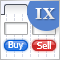图形界面 IX: 进度条和线形图表控件 (第二章)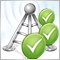评估信号的最简单方式: 交易活动, 回撤/负载, 和 MFE/MAE 分布图表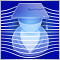神经网络: 智能交易系统自我优化图形界面 IX: 颜色选择器控件 (第一章)